## Background: Representation Learning for NLP

• At a high level, all neural network architectures build representations of input data as vectors/embeddings, which encode useful syntactic and semantic information about the data. These latent or hidden representations can then be used for performing something useful, such as classifying an image or translating a sentence. The neural network learns to build better-and-better representations by receiving feedback, usually via error/loss functions.
• For Natural Language Processing (NLP), conventionally, Recurrent Neural Networks (RNNs) build representations of each word in a sentence in a sequential manner, i.e., one word at a time. Intuitively, we can imagine an RNN layer as a conveyor belt (as shown in the figure below; source), with the words being processed on it autoregressively from left to right. In the end, we get a hidden feature for each word in the sentence, which we pass to the next RNN layer or use for our NLP tasks of choice. Chris Olah’s legendary blog for recaps on LSTMs and representation learning for NLP is highly recommend to develop a background in this area
• Initially introduced for machine translation, Transformers have gradually replaced RNNs in mainstream NLP. The architecture takes a fresh approach to representation learning: Doing away with recurrence entirely, Transformers build features of each word using an attention mechanism (which had also been experimented in the world of RNNs as “Augmented RNNs”) to figure out how important all the other words in the sentence are w.r.t. to the aforementioned word. Knowing this, the word’s updated features are simply the sum of linear transformations of the features of all the words, weighted by their importance (as shown in the figure below; source). Back in 2017, this idea sounded very radical, because the NLP community was so used to the sequential–one-word-at-a-time–style of processing text with RNNs. As recommended reading, Lilian Weng’s Attention? Attention! offers a great overview on various attention types and their pros/cons.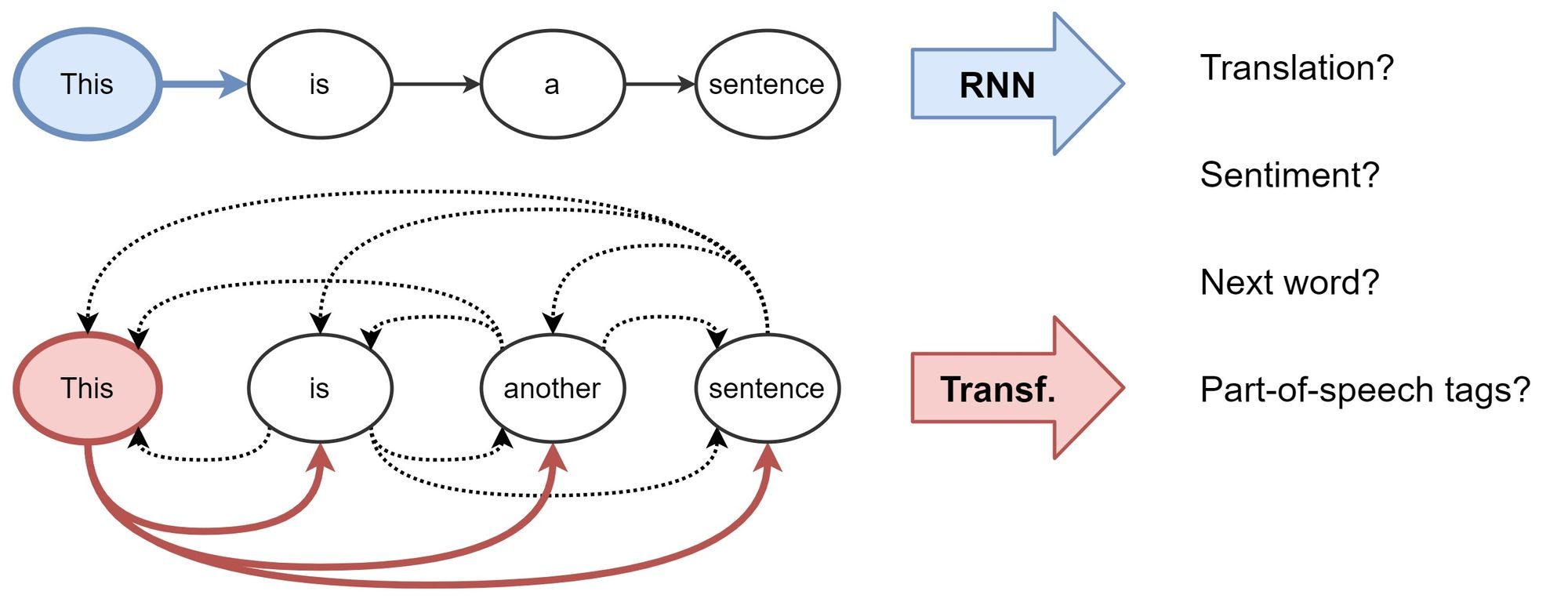## Enter the Transformer

• History:
• LSTMs, GRUs and other flavors of RNNs were the essential building blocks of NLP models for two decades since 1990s.
• CNNs were the essential building blocks of vision (and some NLP) models for three decades since the 1980s.
• In 2017, Transformers (proposed in the “Attention Is All You Need” paper) demonstrated that recurrence and/or convolutions are not essential for building high-performance natural language models.
• In 2020, Vision Transformer (ViT) (An Image is Worth 16x16 Words: Transformers for Image Recognition at Scale) demonstrated that convolutions are not essential for building high-performance vision models.
• The most advanced architectures in use before Transformers gained a foothold in the field were RNNs with LSTMs/GRUs. These architectures, however, suffered from the following drawbacks:
• They struggle with really long sequences (despite using LSTM and GRU units).
• They are fairly slow, as their sequential nature doesn’t allow any kind of parallel computing.
• At the time, LSTM-based recurrent models were the de-facto choice for language modeling. Here’s a timeline of some relevant events:
• ELMo (LSTM-based): 2018
• ULMFiT (LSTM-based): 2018
• Initially introduced for machine translation by Vaswani et al. (2017), the vanilla Transformer model utilizes an encoder-decoder architecture, which is able to perform sequence transduction with a sophisticated attention mechanism. As such, compared to prior recurrent architectures, Transformers possess fundamental differences in terms of how they work:
• They work on the entire sequence calculating attention across all word-pairs, which let them learn long-range dependencies.
• Some parts of the architecture can be processed in parallel, making training much faster.
• Owing to their unique self-attention mechanism, transformer models offer a great deal of representational capacity/expressive power.
• These performance and parallelization benefits led to Transformers gradually replacing RNNs in mainstream NLP. The architecture takes a fresh approach to representation learning: Doing away with recurrence entirely, Transformers build features of each word using an attention mechanism to figure out how important all the other words in the sentence are w.r.t. the aforementioned word. As such, the word’s updated features are simply the sum of linear transformations of the features of all the words, weighted by their importance.
• Back in 2017, this idea sounded very radical, because the NLP community was so used to the sequential – one-word-at-a-time – style of processing text with RNNs. The title of the paper probably added fuel to the fire! For a recap, Yannic Kilcher made an excellent video overview.
• However, Transformers did not become a overnight success until GPT and BERT immensely popularized them. Here’s a timeline of some relevant events:
• Attention is all you need: 2017
• Transformers revolutionizing the world of NLP, Speech, and Vision: 2018 onwards
• GPT (Transformer-based): 2018
• BERT (Transformer-based): 2018
• Today, transformers are not just limited to language tasks but are used in vision, speech, and so much more. The following plot (source) shows the transformers family tree with prevalent models: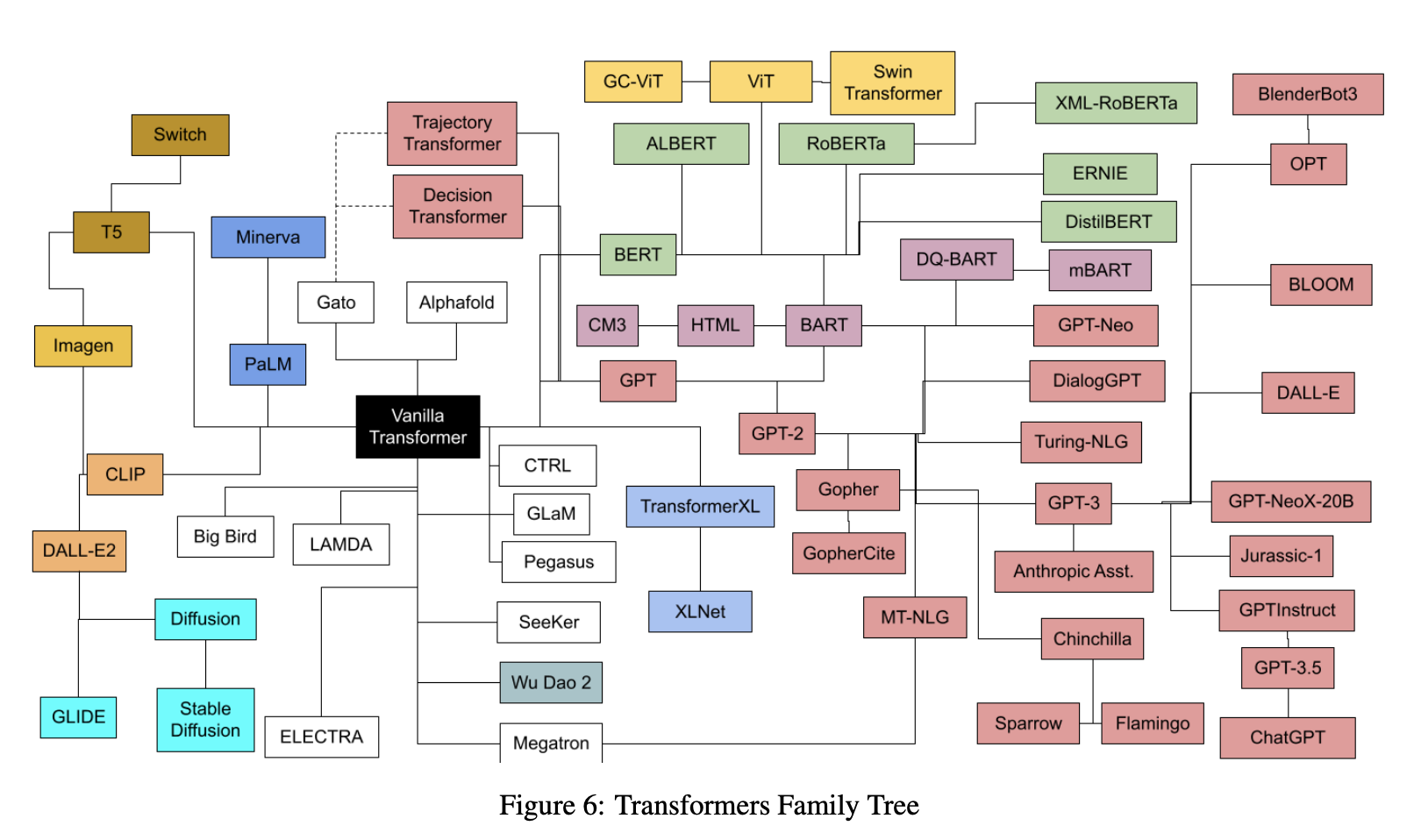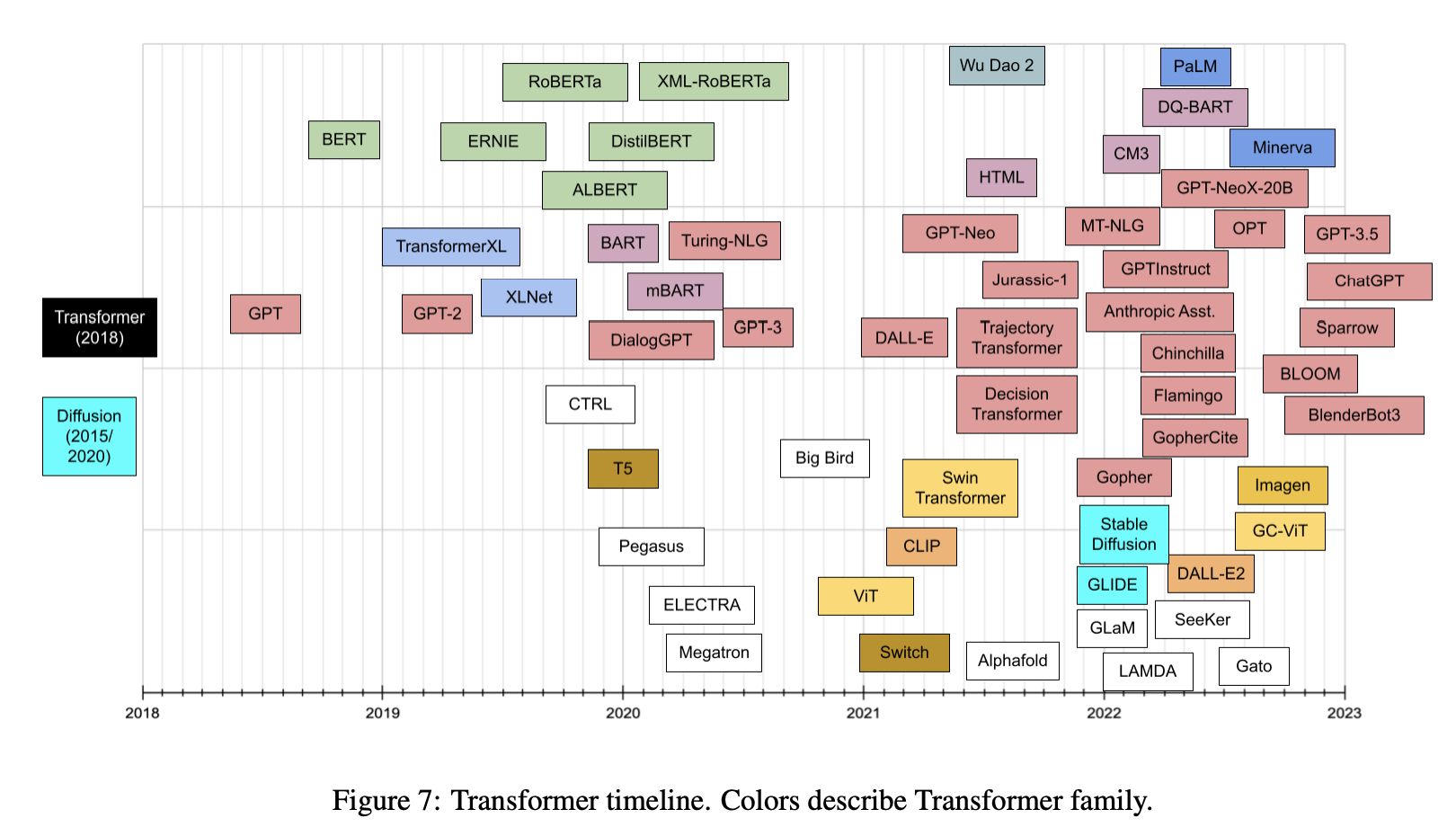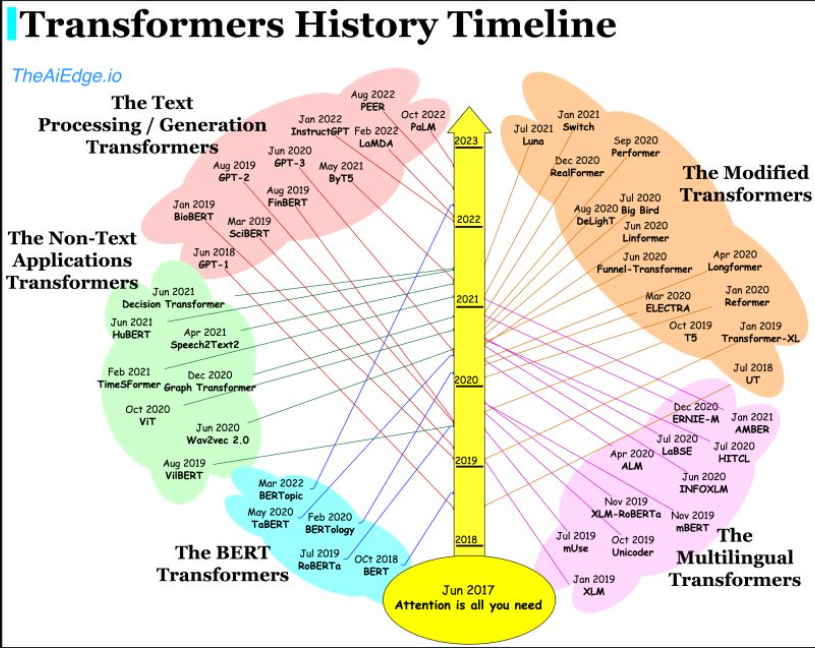• Lastly, the plot below (source) shows the timeline vs. number of parameters for prevalent transformer models: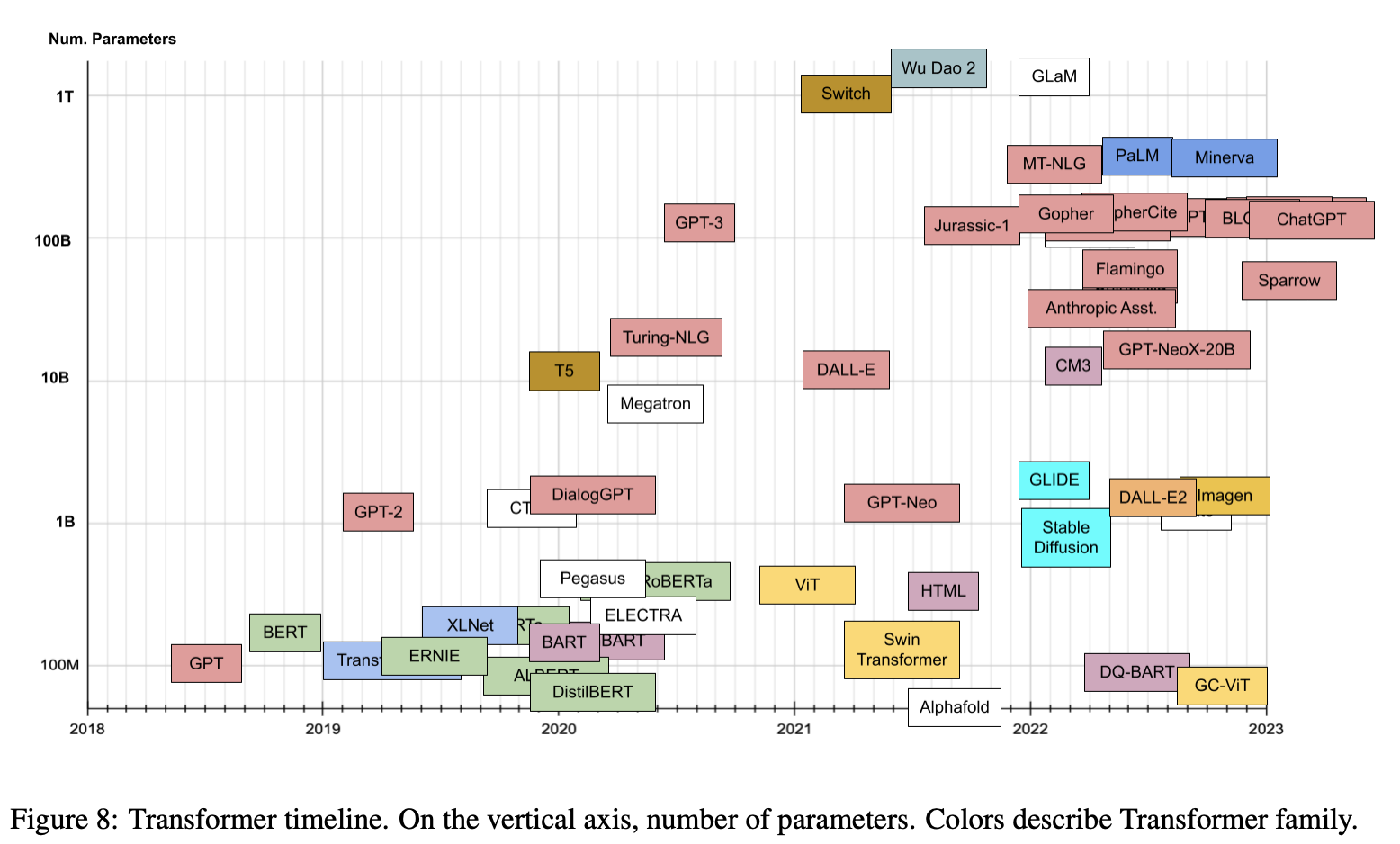## Transformers vs. Recurrent and Convolutional Architectures: An Overview

### Language

• In a vanilla language model, for example, nearby words would first get grouped together. The transformer, by contrast, runs processes so that every element in the input data connects, or pays attention, to every other element. This is referred to as “self-attention.” This means that as soon as it starts training, the transformer can see traces of the entire data set.
• Before transformers came along, progress on AI language tasks largely lagged behind developments in other areas. Infact, in this deep learning revolution that happened in the past 10 years or so, natural language processing was a latecomer and NLP was, in a sense, behind computer vision, per the computer scientist Anna Rumshisky of the University of Massachusetts, Lowell.
• However, with the arrival of Transformers, the field of NLP has received a much-needed push and has churned model after model that have beat the state-of-the-art in various NLP tasks.
• As an example, to understand the difference between vanilla language models (based on say, a recurrent architecture such as RNNs, LSTMs or GRUs) vs. transformers, consider these sentences: “The owl spied a squirrel. It tried to grab it with its talons but only got the end of its tail.” The structure of the second sentence is confusing: What do those “it”s refer to? A vanilla language model that focuses only on the words immediately around the “it”s would struggle, but a transformer connecting every word to every other word could discern that the owl did the grabbing, and the squirrel lost part of its tail.

### Vision

In CNNs, you start off being very local and slowly get a global perspective. A CNN recognizes an image pixel by pixel, identifying features like edges, corners, or lines by building its way up from the local to the global. But in transformers, owing to self-attention, even the very first attention layer models global contextual information, making connections between distant image locations (just as with language). If we model a CNN’s approach as starting at a single pixel and zooming out, a transformer slowly brings the whole fuzzy image into focus.

• CNNs work by repeatedly applying filters on local patches of the input data, generating local feature representations (or “feature maps”) and incrementally increase their receptive field and build up to global feature representations. It is because of convolutions that photo apps can organize your library by faces or tell an avocado apart from a cloud. Prior to the transformer architecture, CNNs were thus considered indispensable to vision tasks.
• With the Vision Transformer (ViT), the architecture of the model is nearly identical to that of the first transformer proposed in 2017, with only minor changes allowing it to analyze images instead of words. Since language tends to be discrete, a lot of adaptations were to discretize the input image to make transformers work with visual input. Exactly mimicing the language approach and performing self-attention on every pixel would be prohibitively expensive in computing time. Instead, ViT divides the larger image into square units, or patches (akin to tokens in NLP). The size is arbitrary, as the tokens could be made larger or smaller depending on the resolution of the original image (the default is 16x16 pixels). But by processing pixels in groups, and applying self-attention to each, the ViT could quickly churn through enormous training data sets, spitting out increasingly accurate classifications.
• In Do Vision Transformers See Like Convolutional Neural Networks?, Raghu et al. sought to understand how self-attention powers transformers in vision-based tasks.

• As discussed in the Enter the Transformer section, other architectures are “one trick ponies” while multimodal learning requires handling of modalities with different patterns within a streamlined architecture with a reasonably high relational inductive bias to even remotely reach human-like intelligence. In other words, we needs a single versatile architecture that seamlessly transitions between senses like reading/seeing, speaking, and listening.
• The potential to offer a universal architecture that can be adopted for multimodal tasks (that requires simultaneously handling multiple types of data, such as raw images, video and language) is something that makes the transformer architecture unique and popular.
• Because of the siloed approach with earlier architectures where each type of data had its own specialized model, this was a difficult task to accomplish. However, transformers offer an easy way to combine multiple input sources. For example, multimodal networks might power a system that reads a person’s lips in addition to listening to their voice using rich representations of both language and image information.
• With cross-attention where the query, key and value vectors are derived from different sources, transformers are able to lend themselves as a powerful tool for multimodal learning.
• The transformer thus offers be a big step toward achieving a kind of “convergence” for neural net architectures, resulting in a universal approach to processing data from multiple modalities.

## Breaking down the Transformer

• Before we pop open the hood of the Transformer and go through each component one by one, let’s first setup a background in underlying concepts such as one-hot vectors, dot product, matrix multiplication, embedding generation, and attention.

### Background

#### One-hot encoding

##### Overview
• Computers process numerical data. However, in most practical scenarios, the input data is not naturally numeric, for e.g., images (we model intensity values as pixels), speech (we model the audio signal as an oscillogram/spectrogram). Our first step is to convert all the words to numbers so we can do math on them.
• One hot encoding is a process by which categorical variables are converted into a form that could be provided to ML algorithms to do a better job in prediction.
##### Idea
• So, you’re playing with ML models and you encounter this “one-hot encoding” term all over the place. You see the sklearn documentation for one-hot encoder and it says “encode categorical integer features using a one-hot aka one-of-K scheme.” To demystify that, let’s look at what one-hot encoding actually is, through an example.
##### Example: Basic Dataset
• Suppose the dataset is as follows:

  ╔════════════╦═════════════════╦════════╗
║ CompanyName Categoricalvalue ║ Price  ║
╠════════════╬═════════════════╣════════║
║ VW         ╬      1          ║ 20000  ║
║ Acura      ╬      2          ║ 10011  ║
║ Honda      ╬      3          ║ 50000  ║
║ Honda      ╬      3          ║ 10000  ║
╚════════════╩═════════════════╩════════╝

• The categorical value represents the numerical value of the entry in the dataset. For example: if there were to be another company in the dataset, it would have been given categorical value as 4. As the number of unique entries increases, the categorical values also proportionally increases.
• The previous table is just a representation. In reality, the categorical values start from 0 goes all the way up to $$N-1$$ categories.
• As you probably already know, the categorical value assignment can be done using sklearn’s LabelEncoder.
• Now let’s get back to one-hot encoding: Say we follow instructions as given in the sklearn’s documentation for one-hot encoding and follow it with a little cleanup, we end up with the following:

  ╔════╦══════╦══════╦════════╗
║ VW ║ Acura║ Honda║ Price  ║
╠════╬══════╬══════╬════════║
║ 1  ╬ 0    ╬ 0    ║ 20000  ║
║ 0  ╬ 1    ╬ 0    ║ 10011  ║
║ 0  ╬ 0    ╬ 1    ║ 50000  ║
║ 0  ╬ 0    ╬ 1    ║ 10000  ║
╚════╩══════╩══════╩════════╝

• where 0 indicates non-existent while 1 indicates existent.
• Before we proceed further, could you think of one reason why just label encoding is not sufficient to provide to the model for training? Why do you need one-hot encoding?
• Problem with label encoding is that it assumes higher the categorical value, better the category. Specifically, what this form of organization presupposes is VW > Acura > Honda based on the categorical values. Say supposing your model internally calculates average, then accordingly we get, 1+3 = 4/2 = 2. This implies that: Average of VW and Honda is Acura. This is definitely a recipe for disaster. This model’s prediction would have a lot of errors.
• This is why we use one-hot encoder to perform “binarization” of the category and include it as a feature to train the model.
• As another example: Suppose you have flower feature which can take values daffodil, lily, and rose. One hot encoding converts flower feature to three features, is_daffodil, is_lily, and is_rose which all are binary.
##### Example: NLP
• Inspired by Brandon Rohrer’s Transformers From Scratch, let’s consider another example in the context of natural language processing. Imagine that our goal is to create the computer that processes text, say a Machine Translation system that translates computer commands from one language to another. Such a model would ingest the input text and convert (or transduce) a sequence of sounds to a sequence of words.
• We start by choosing our vocabulary, the collection of symbols that we are going to be working with in each sequence. In our case, there will be two different sets of symbols, one for the input sequence to represent vocal sounds and one for the output sequence to represent words.
• For now, let’s assume we’re working with English. There are tens of thousands of words in the English language, and perhaps another few thousand to cover computer-specific terminology. That would give us a vocabulary size that is the better part of a hundred thousand. One way to convert words to numbers is to start counting at one and assign each word its own number. Then a sequence of words can be represented as a list of numbers.
• For example, consider a tiny language with a vocabulary size of three: files, find, and my. Each word could be swapped out for a number, perhaps files = 1, find = 2, and my = 3. Then the sentence “Find my files”, consisting of the word sequence [find, my, files] could be represented instead as the sequence of numbers [2, 3, 1].
• This is a perfectly valid way to convert symbols to numbers, but it turns out that there’s another format that’s even easier for computers to work with, one-hot encoding. In one-hot encoding a symbol is represented by an array of mostly zeros, the same length of the vocabulary, with only a single element having a value of one. Each element in the array corresponds to a separate symbol.
• Another way to think about one-hot encoding is that each word still gets assigned its own number, but now that number is an index to an array. Here is our example above, in one-hot notation.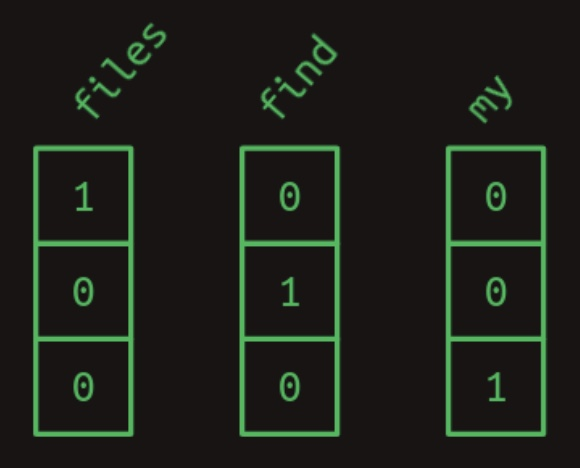• So the phrase find my files becomes a sequence of one-dimensional arrays, which, after squeezing together, looks like a two-dimensional array.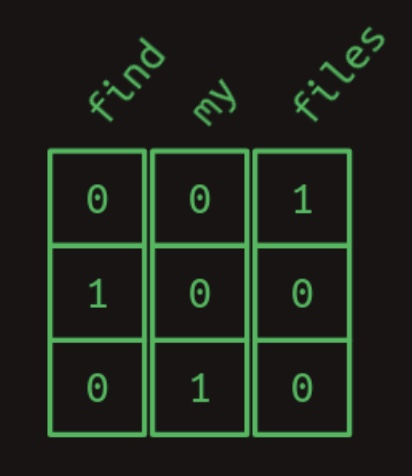• The terms “one-dimensional array” and “vector” are typically used interchangeably (in this article and otherwise). Similarly, “two-dimensional array” and “matrix” can be interchanged as well.

#### Dot product

• One really useful thing about the one-hot representation is that it lets us compute dot product (also referred to as the inner product, scalar product or cosine similarity).
##### Algebraic Definition
• The dot product of two vectors $$\mathbf{a}=\left[a_{1}, a_{2}, \ldots, a_{n}\right]$$ and $$\mathbf{b}=\left[b_{1}, b_{2}, \ldots, b_{n}\right]$$ is defined as:

$\mathbf{a} \cdot \mathbf{b}=\sum_{i=1}^{n} a_{i} b_{i}=a_{1} b_{1}+a_{2} b_{2}+\cdots+a_{n} b_{n}$
• where $\Sigma$ denotes summation and $n$ is the dimension of the vector space.
• For instance, in three-dimensional space, the dot product of vectors $$[1, 3, -5]$$ and $$[4,-2,-1]$$ is:

\begin{aligned} {[1,3,-5] \cdot[4,-2,-1] } &=(1 \times 4)+(3 \times-2)+(-5 \times-1) \\ &=4-6+5 \\ &=3 \end{aligned}
• The dot product can also be written as a product of two vectors, as below.

$\mathbf{a} \cdot \mathbf{b}=\mathbf{a b}^{\top}$
• where $$\mathbf{b}^{\top}$$ denotes the transpose of $$\mathbf{b}$$.
• Expressing the above example in this way, a $$1 \times 3$$ matrix (row vector) is multiplied by a $$3 \times 1$$ matrix (column vector) to get a $$1 \times 1$$ matrix that is identified with its unique entry:

$\left[\begin{array}{lll} 1 & 3 & -5 \end{array}\right]\left[\begin{array}{c} 4 \\ -2 \\ -1 \end{array}\right]=3$
• Key takeaway:

• In summary, to get the dot product of two vectors, multiply their corresponding elements, then add the results. For a visual example of calculating the dot product for two vectors, check out the figure below.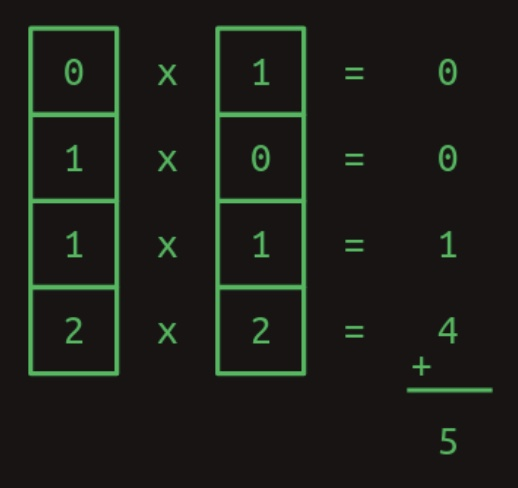##### Geometric Definition
• In Euclidean space, a Euclidean vector is a geometric object that possesses both a magnitude and a direction. A vector can be pictured as an arrow. Its magnitude is its length, and its direction is the direction to which the arrow points. The magnitude of a vector a is denoted by $$\mid \mid a \mid \mid$$. The dot product of two Euclidean vectors $$\mathbf{a}$$ and $$\mathbf{b}$$ is defined by,

$\mathbf{a} \cdot \mathbf{b}=\|\mathbf{a}\|\|\mathbf{b}\| \cos \theta$
• where $$\theta$$ is the angle between $$\mathbf{a}$$ and $$\mathbf{b}$$.
• The above equation establishes the relation between dot product and cosine similarity.

##### Properties of the dot product
• Dot products are especially useful when we’re working with our one-hot word representations owing to it’s properties, some of which are highlighted below.

• The dot product of any one-hot vector with itself is one.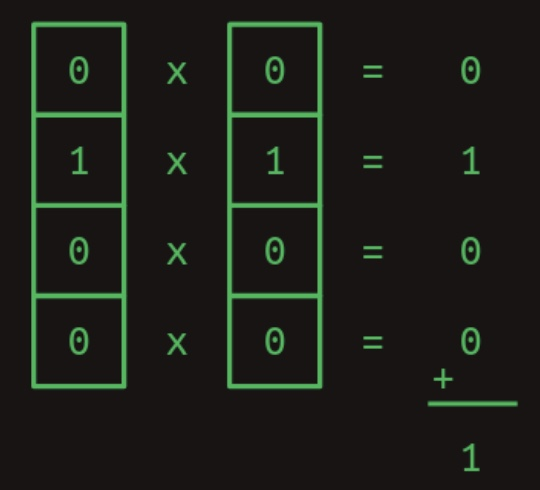• The dot product of any one-hot vector with another one-hot vector is zero.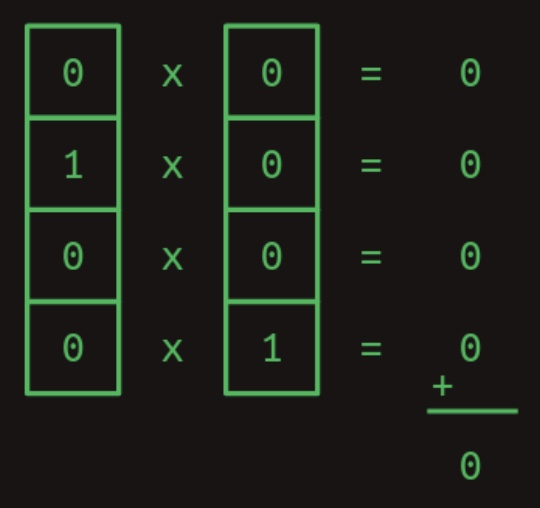• The previous two examples show how dot products can be used to measure similarity. As another example, consider a vector of values that represents a combination of words with varying weights. A one-hot encoded word can be compared against it with the dot product to show how strongly that word is represented. The following figure shows how a similarity score between two vectors is calculated by way of calculating the dot product.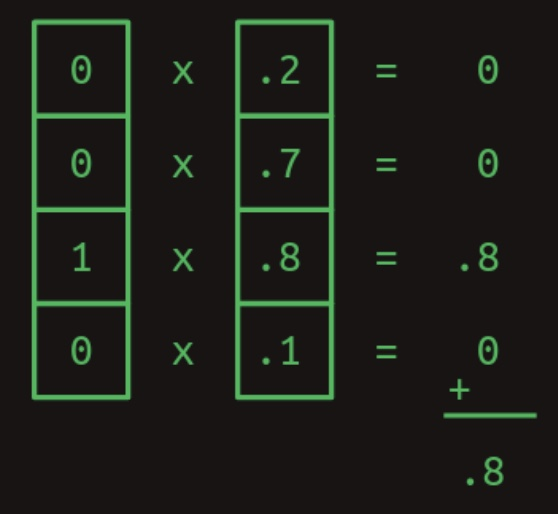#### Matrix multiplication as a series of dot products

• The dot product is the building block of matrix multiplication, a very particular way to combine a pair of two-dimensional arrays. We’ll call the first of these matrices $$A$$ and the second one $$B$$. In the simplest case, when $$A$$ has only one row and $$B$$ has only one column, the result of matrix multiplication is the dot product of the two. The following figure shows the multiplication of a single row matrix and a single column matrix.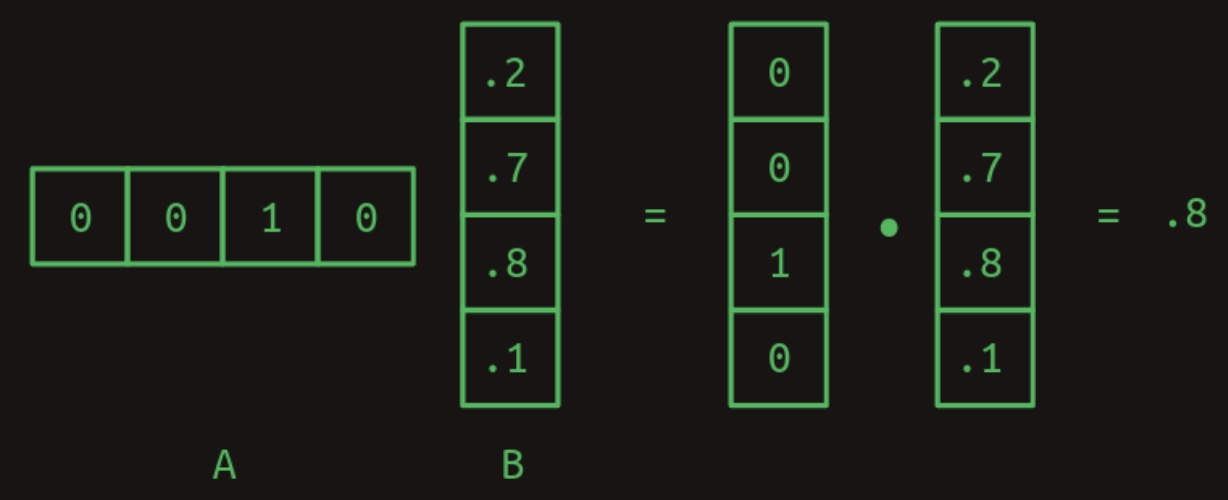• Notice how the number of columns in A and the number of rows in $$B$$ needs to be the same for the two arrays to match up and for the dot product to work out.
• When $$A$$ and $$B$$ start to grow, matrix multiplication starts to increase quadratically in time complexity. To handle more than one row in $$A$$, take the dot product of $$B$$ with each row separately. The answer will have as many rows as A does. The following figure shows the multiplication of a two row matrix and a single column matrix.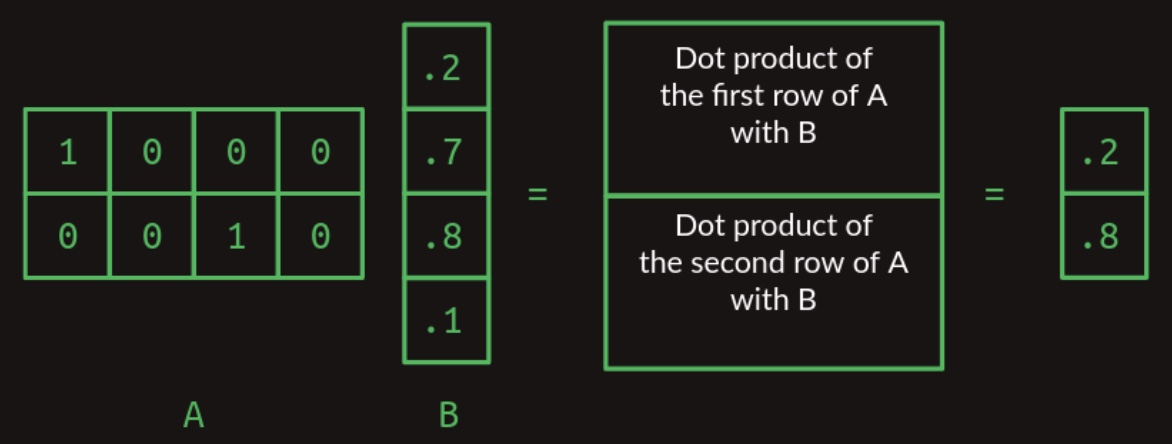• When $$B$$ takes on more columns, take the dot product of each column with $$A$$ and stack the results in successive columns. The following figure shows the multiplication of a one row matrix and a two column matrix: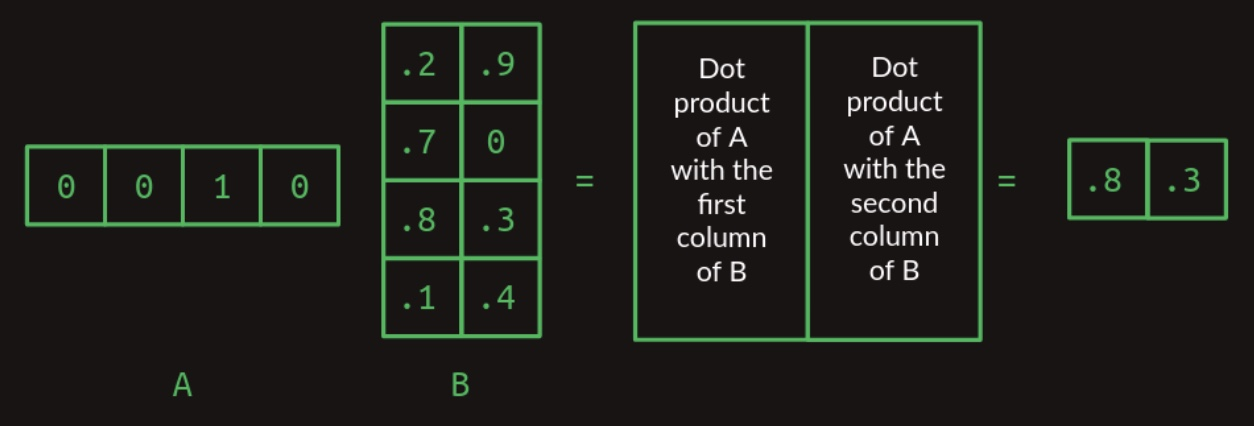• Now we can extend this to mutliplying any two matrices, as long as the number of columns in $$A$$ is the same as the number of rows in $$B$$. The result will have the same number of rows as $$A$$ and the same number of columns as $$B$$. The following figure shows the multiplication of a one three matrix and a two column matrix: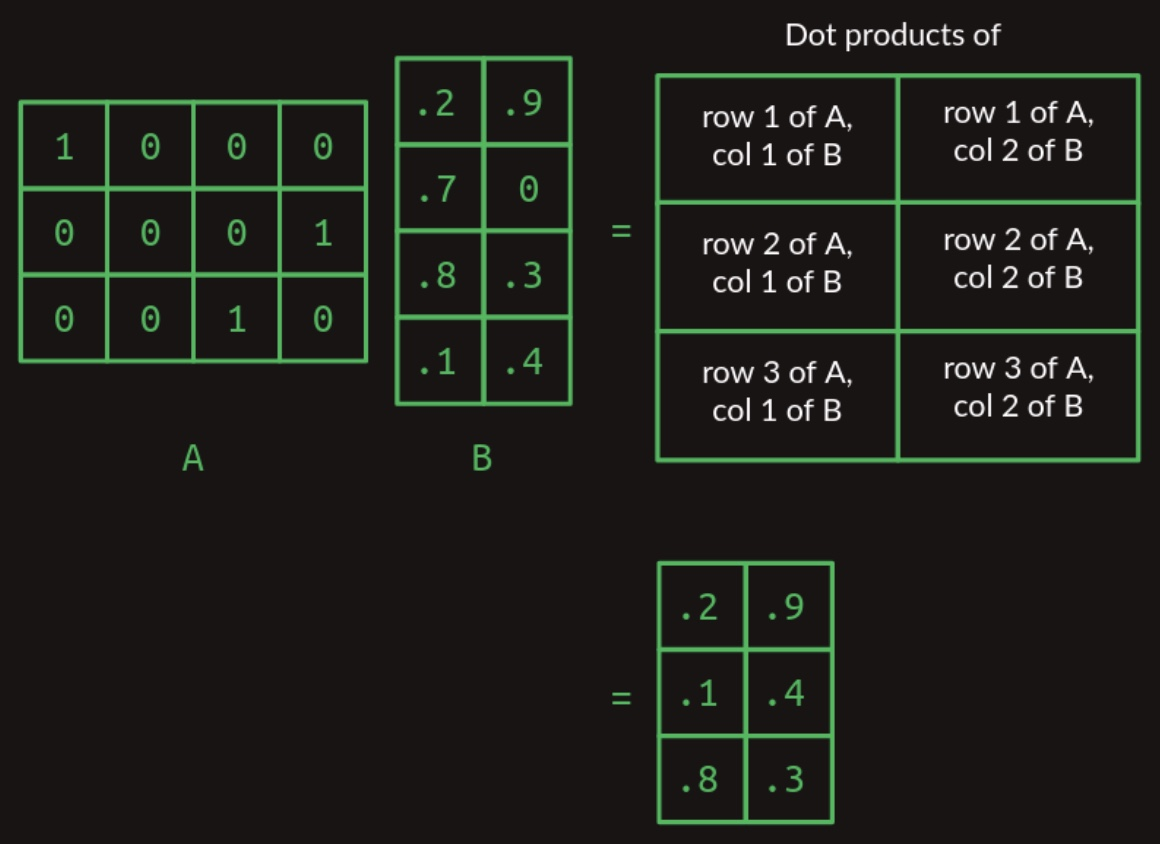##### Matrix multiplication as a table lookup
• In the above section, we saw how matrix multiplication acts as a lookup table.
• The matrix $$A$$ is made up of a stack of one-hot vectors. They have ones in the first column, the fourth column, and the third column, respectively. When we work through the matrix multiplication, this serves to pull out the first row, the fourth row, and the third row of the $$B$$ matrix, in that order. This trick of using a one-hot vector to pull out a particular row of a matrix is at the core of how transformers work.

#### First order sequence model

• We can set aside matrices for a minute and get back to what we really care about, sequences of words. Imagine that as we start to develop our natural language computer interface we want to handle just three possible commands:
Show me my directories please.

• Our vocabulary size is now seven:
{directories, files, me, my, photos, please, show}

• One useful way to represent sequences is with a transition model. For every word in the vocabulary, it shows what the next word is likely to be. If users ask about photos half the time, files 30% of the time, and directories the rest of the time, the transition model will look like this. The sum of the transitions away from any word will always add up to one. The following figure shows a Markov chain transition model.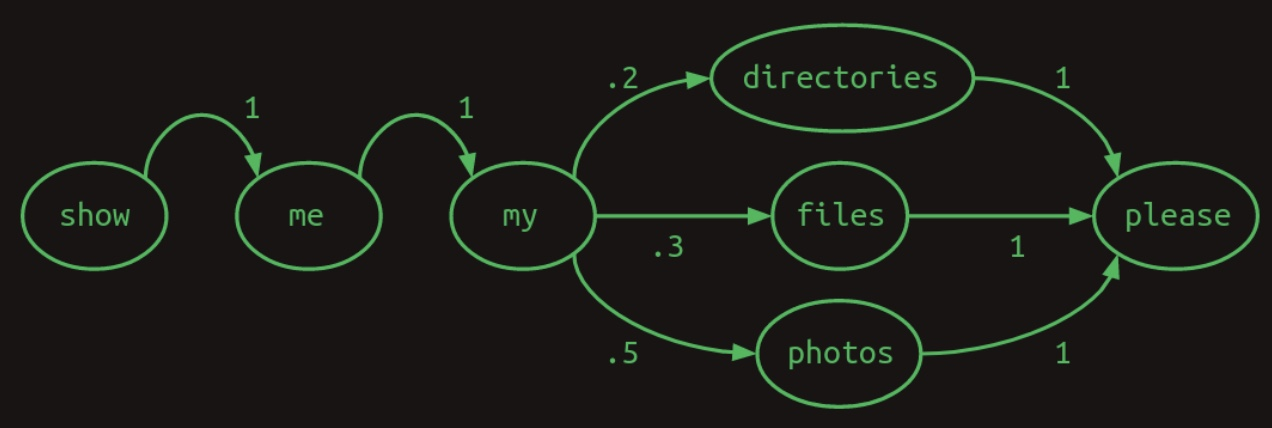• This particular transition model is called a Markov chain, because it satisfies the Markov property that the probabilities for the next word depend only on recent words. More specifically, it is a first order Markov model because it only looks at the single most recent word. If it considered the two most recent words it would be a second order Markov model.

• Our break from matrices is over. It turns out that Markov chains can be expressed conveniently in matrix form. Using the same indexing scheme that we used when creating one-hot vectors, each row represents one of the words in our vocabulary. So does each column. The matrix transition model treats a matrix as a lookup table. Find the row that corresponds to the word you’re interested in. The value in each column shows the probability of that word coming next. Because the value of each element in the matrix represents a probability, they will all fall between zero and one. Because probabilities always sum to one, the values in each row will always add up to one. The following diagram from Brandon Rohrer’s Transformers From Scratch shows a transition matrix: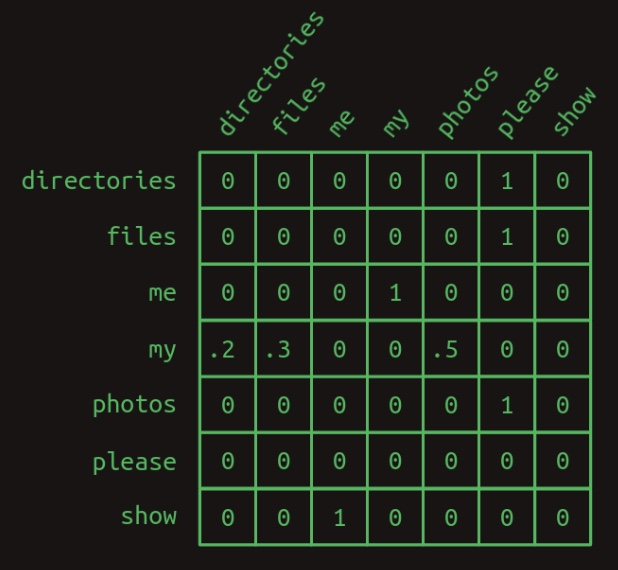• In the transition matrix here we can see the structure of our three sentences clearly. Almost all of the transition probabilities are zero or one. There is only one place in the Markov chain where branching happens. After my, the words directories, files, or photos might appear, each with a different probability. Other than that, there’s no uncertainty about which word will come next. That certainty is reflected by having mostly ones and zeros in the transition matrix.

• We can revisit our trick of using matrix multiplication with a one-hot vector to pull out the transition probabilities associated with any given word. For instance, if we just wanted to isolate the probabilities of which word comes after my, we can create a one-hot vector representing the word my and multiply it by our transition matrix. This pulls out the row the relevant row and shows us the probability distribution of what the next word will be. The following diagram from Brandon Rohrer’s Transformers From Scratch shows a transition probability lookup using a transition matrix: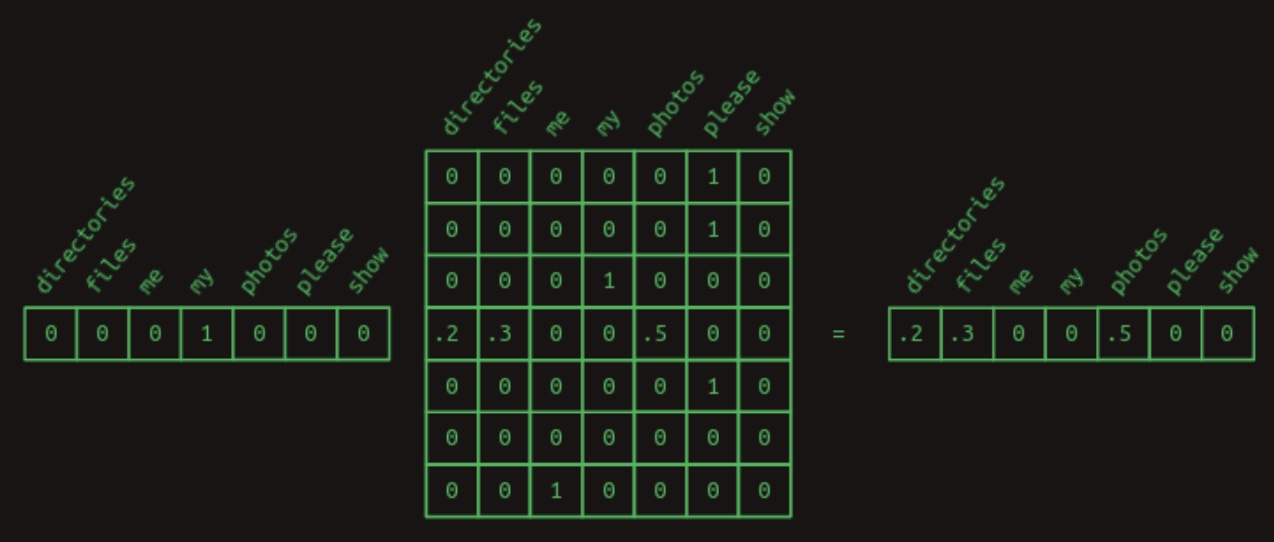#### Second order sequence model

• Predicting the next word based on only the current word is hard. That’s like predicting the rest of a tune after being given just the first note. Our chances are a lot better if we can at least get two notes to go on.
• We can see how this works in another toy language model for our computer commands. We expect that this one will only ever see two sentences, in a 40/60 proportion.
Check whether the battery ran down please.
Check whether the program ran please.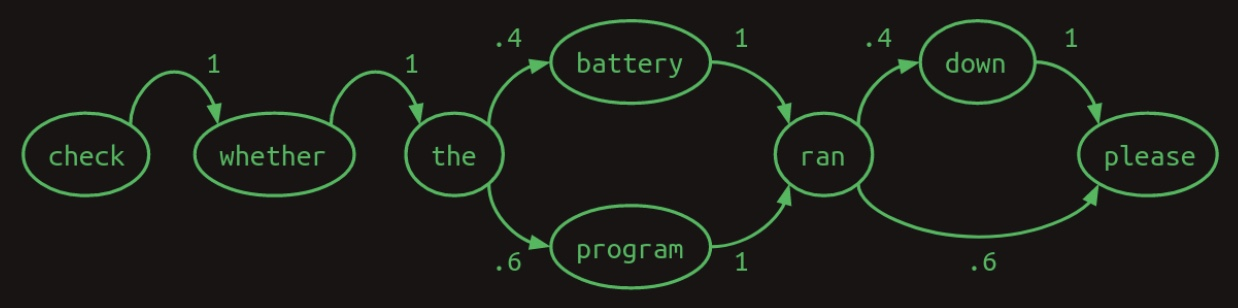• Here we can see that if our model looked at the two most recent words, instead of just one, that it could do a better job. When it encounters battery ran, it knows that the next word will be down, and when it sees program ran the next word will be please. This eliminates one of the branches in the model, reducing uncertainty and increasing confidence. Looking back two words turns this into a second order Markov model. It gives more context on which to base next word predictions. Second order Markov chains are more challenging to draw, but here are the connections that demonstrate their value. The following diagram from Brandon Rohrer’s Transformers From Scratch shows a second order Markov chain.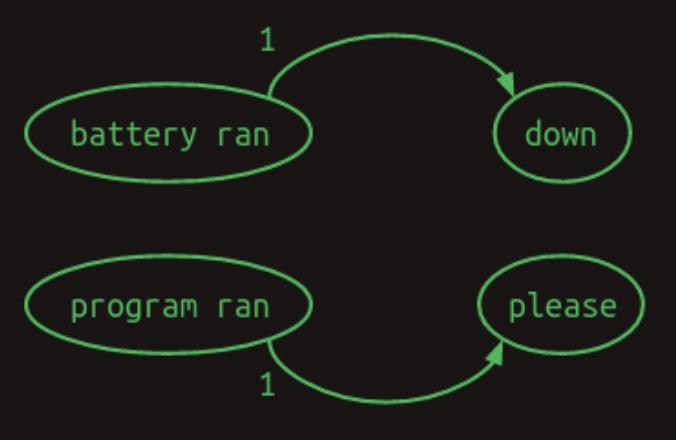• To highlight the difference between the two, here is the first order transition matrix,
• Here’s a first order transition matrix: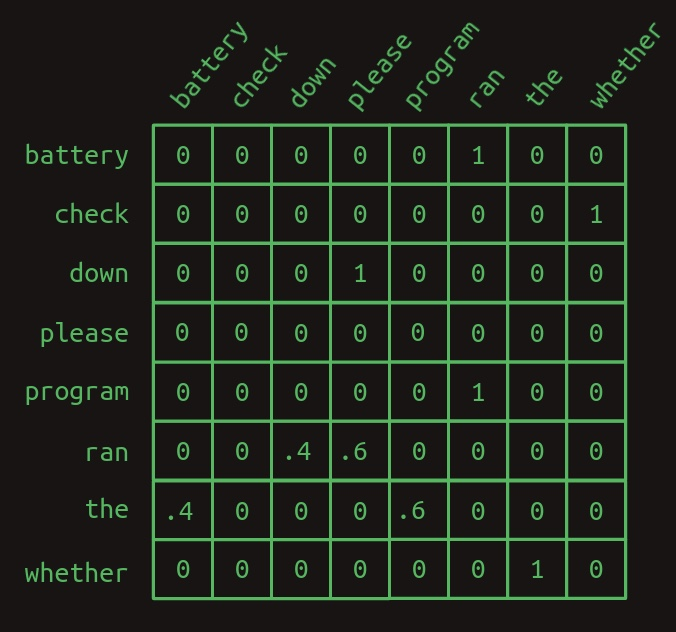• … and here is the second order transition matrix: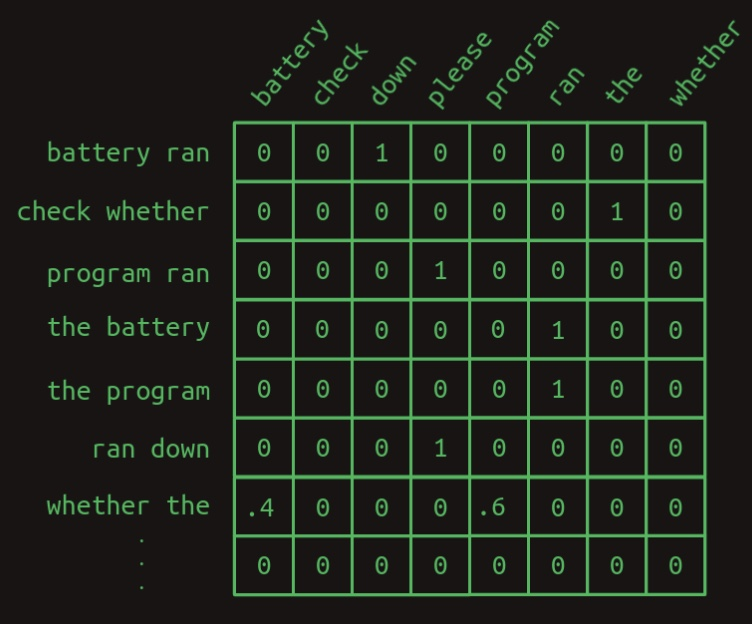• Notice how the second order matrix has a separate row for every combination of words (most of which are not shown here). That means that if we start with a vocabulary size of $$N$$ then the transition matrix has $$N^2$$ rows.
• What this buys us is more confidence. There are more ones and fewer fractions in the second order model. There’s only one row with fractions in it, one branch in our model. Intuitively, looking at two words instead of just one gives more context, more information on which to base a next word guess.

#### Second order sequence model with skips

• A second order model works well when we only have to look back two words to decide what word comes next. What about when we have to look back further? Imagine we are building yet another language model. This one only has to represent two sentences, each equally likely to occur.
Check the program log and find out whether it ran please.
Check the battery log and find out whether it ran down please.

• In this example, in order to determine which word should come after ran, we would have to look back 8 words into the past. If we want to improve on our second order language model, we can of course consider third- and higher order models. However, with a significant vocabulary size this takes a combination of creativity and brute force to execute. A naive implementation of an eighth order model would have $$N^8$$ rows, a ridiculous number for any reasonable vocabulary.
• Instead, we can do something sly and make a second order model, but consider the combinations of the most recent word with each of the words that came before. It’s still second order, because we’re only considering two words at a time, but it allows us to reach back further and capture long range dependencies. The difference between this second-order-with-skips and a full umpteenth-order model is that we discard most of the word order information and combinations of preceding words. What remains is still pretty powerful.
• Markov chains fail us entirely now, but we can still represent the link between each pair of preceding words and the words that follow. Here we’ve dispensed with numerical weights, and instead are showing only the arrows associated with non-zero weights. Larger weights are shown with heavier lines. The following diagram from Brandon Rohrer’s Transformers From Scratch shows a second order sequence model with skips feature voting.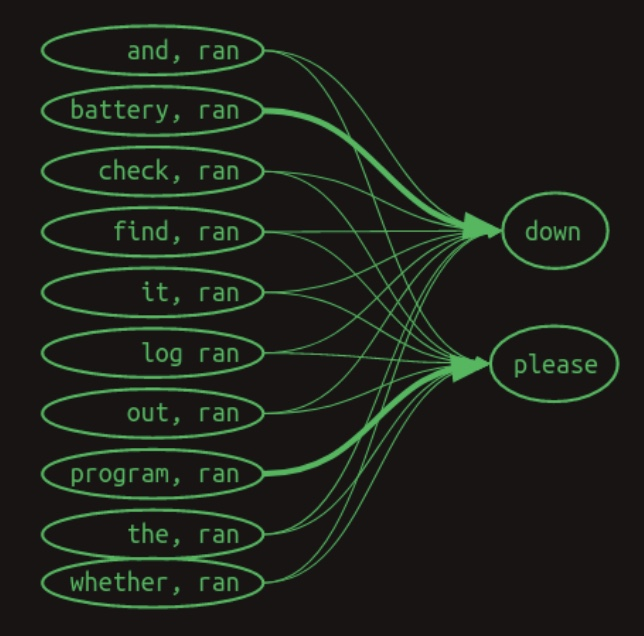• Here’s what it might look like in a second order with skips transition matrix.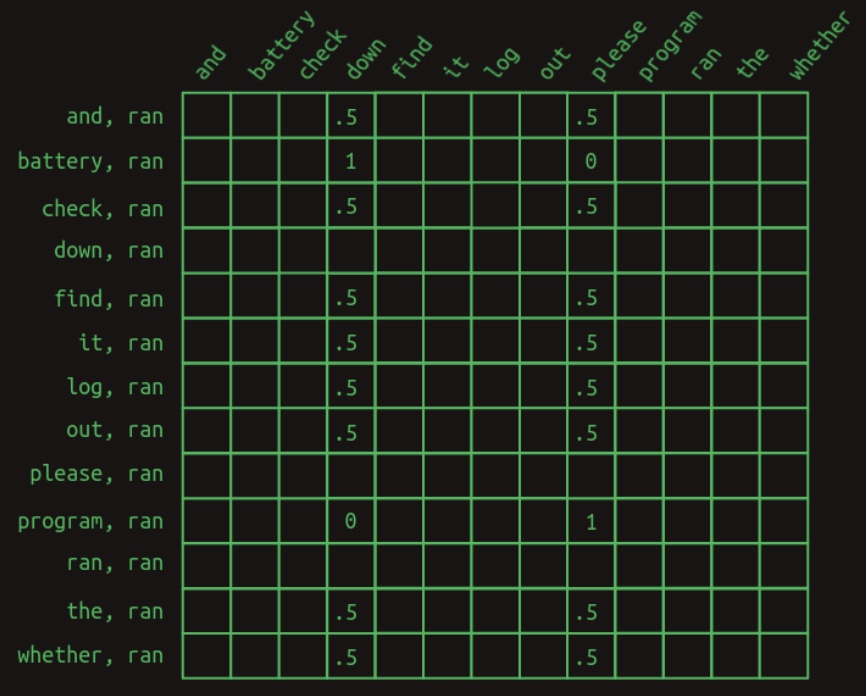• This view only shows the rows relevant to predicting the word that comes after ran. It shows instances where the most recent word (ran) is preceded by each of the other words in the vocabulary. Only the relevant values are shown. All the empty cells are zeros.

• The first thing that becomes apparent is that, when trying to predict the word that comes after ran, we no longer look at just one line, but rather a whole set of them. We’ve moved out of the Markov realm now. Each row no longer represents the state of the sequence at a particular point. Instead, each row represents one of many features that may describe the sequence at a particular point. The combination of the most recent word with each of the words that came before makes for a collection of applicable rows, maybe a large collection. Because of this change in meaning, each value in the matrix no longer represents a probability, but rather a vote. Votes will be summed and compared to determine next word predictions.

• The next thing that becomes apparent is that most of the features don’t matter. Most of the words appear in both sentences, and so the fact that they have been seen is of no help in predicting what comes next. They all have a value of 0.5. The only two exceptions are battery and program. They have some 1 and 0 weights associated with the. The feature battery, ran indicates that ran was the most recent word and that battery occurred somewhere earlier in the sentence. This feature has a weight of 1 associated with down and a weight of 0 associated with please. Similarly, the feature program, ran has the opposite set of weights. This structure shows that it is the presence of these two words earlier in the sentence that is decisive in predicting which word comes next.

• To convert this set of word-pair features into a next word estimate, the values of all the relevant rows need to be summed. Adding down the column, the sequence Check the program log and find out whether it ran generates sums of 0 for all the words, except a 4 for down and a 5 for please. The sequence Check the battery log and find out whether it ran does the same, except with a 5 for down and a 4 for please. By choosing the word with the highest vote total as the next word prediction, this model gets us the right answer, despite having an eight word deep dependency.

• On more careful consideration, this is unsatisfying – the difference between a vote total of 4 and 5 is relatively small. It suggests that the model isn’t as confident as it could be. And in a larger, more organic language model it’s easy to imagine that such a slight difference could be lost in the statistical noise.
• We can sharpen the prediction by weeding out all the uninformative feature votes. With the exception of battery, ran and program, ran. It’s helpful to remember at this point that we pull the relevant rows out of the transition matrix by multiplying it with a vector showing which features are currently active. For this example so far, we’ve been using the implied feature vector shown here. The following diagram from Brandon Rohrer’s Transformers From Scratch shows a feature selection vector.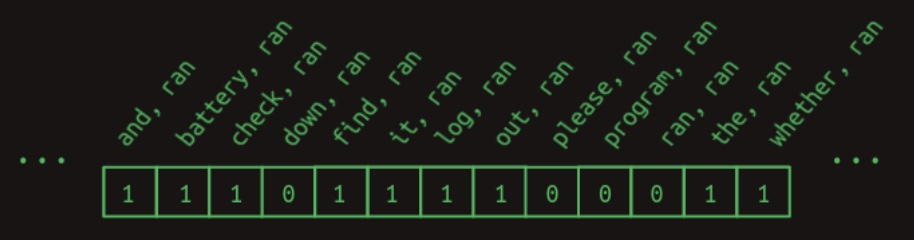• It includes a one for each feature that is a combination of ran with each of the words that come before it. Any words that come after it don’t get included in the feature set. (In the next word prediction problem these haven’t been seen yet, and so it’s not fair to use them predict what comes next.) And this doesn’t include all the other possible word combinations. We can safely ignore these for this example because they will all be zero.
• To improve our results, we can additionally force the unhelpful features to zero by creating a mask. It’s a vector full of ones except for the positions you’d like to hide or mask, and those are set to zero. In our case we’d like to mask everything except for battery, ran and program, ran, the only two features that have been of any help. The following diagram from Brandon Rohrer’s Transformers From Scratch shows a masked feature vector.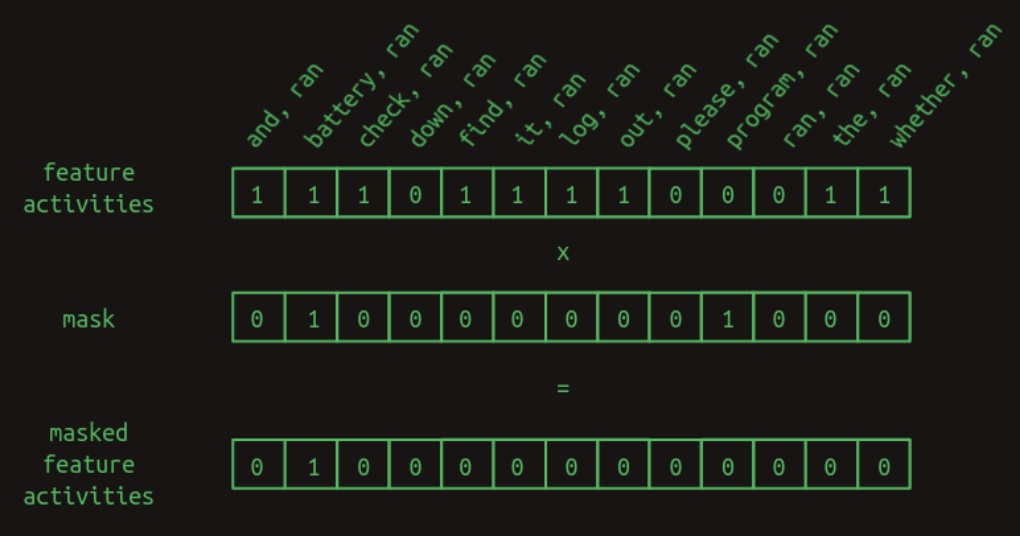• To apply the mask, we multiply the two vectors element by element. Any feature activity value in an unmasked position will be multiplied by one and left unchanged. Any feature activity value in a masked position will be multiplied by zero, and thus forced to zero.
• The mask has the effect of hiding a lot of the transition matrix. It hides the combination of ran with everything except battery and program, leaving just the features that matter. The following diagram from Brandon Rohrer’s Transformers From Scratch shows a masked transition matrix.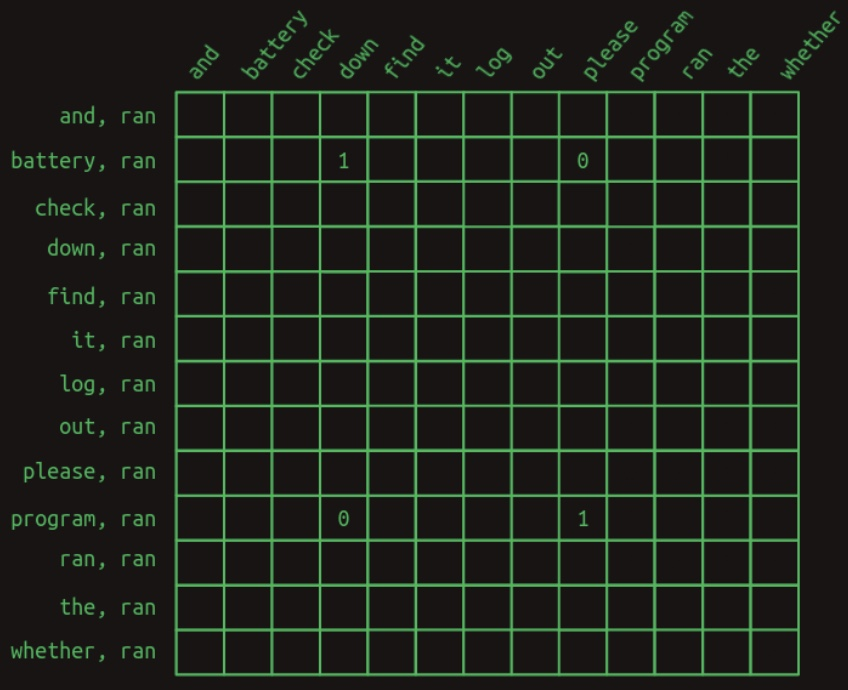• After masking the unhelpful features, the next word predictions become much stronger. When the word battery occurs earlier in the sentence, the word after ran is predicted to be down with a weight of 1 and please with a weight of 0. What was a weight difference of 25 percent has become a difference of infinity percent. There is no doubt what word comes next. The same strong prediction occurs for please when program occurs early on.
• This process of selective masking is the attention called out in the title of the original paper on transformers. So far, what we’ve described is a just an approximation of how attention is implemented in the paper.

Generally speaking, an attention function computes the weights that should be assigned to a particular element of the input to generate the output. In the context of the specific attention function called scaled dot-product attention that Transformers deploy which adopts the query-key-value paradigm from the field of information retrieval, an attention function is the mapping between a query and a set of key-value pairs to an output. The output is computed as a weighted sum of the values, where the weight assigned to each value is computed by a compatibility function (referred to as the “alignment” function in Dazmitry Bahdanau’s original paper from Bengio’s lab that introduced attention) of the query with the corresponding key. While this captures a top-level overview of the important concepts, the details are discussed in the section on Attention.

##### Origins of attention
• As mentioned above, the attention mechanism originally introduced in Bahdanau et al. (2015) served as a foundation upon which the self-attention mechanism in the Transformer paper was based on.
• The following slide from Stanford’s CS25 course shows how the attention mechanism was conceived and is a perfect illustration of why AI/ML is an empirical field, built on intuition.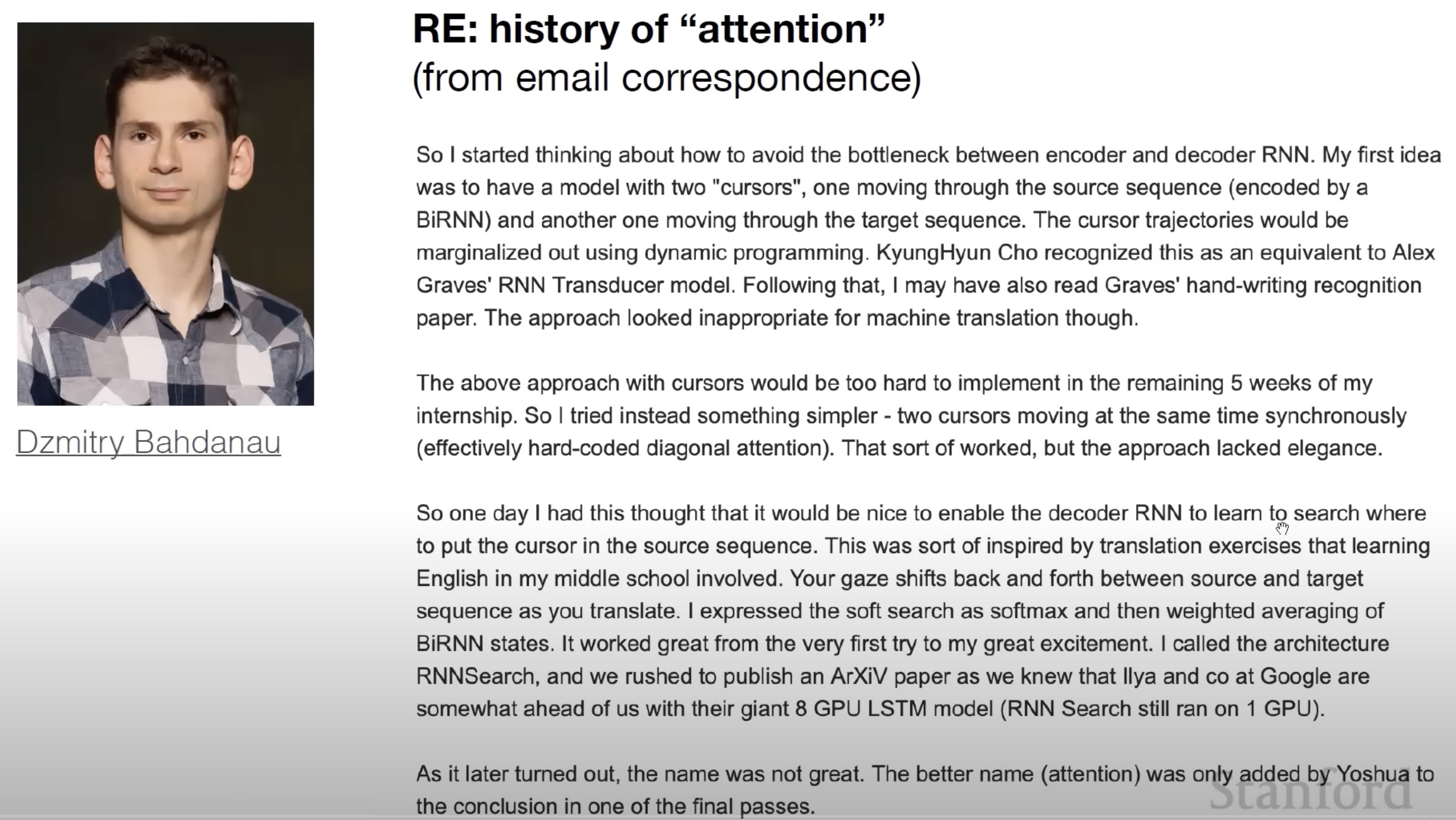#### From Feature Vectors to Transformers

• The selective-second-order-with-skips model is a useful way to think about what transformers do, at least in the decoder side. It captures, to a first approximation, what generative language models like OpenAI’s GPT-3 are doing. It doesn’t tell the complete story, but it represents the central gist of it.
• The next sections cover more of the gap between this intuitive explanation and how transformers are implemented. These are largely driven by three practical considerations:
1. Computers are especially good at matrix multiplications. There is an entire industry around building computer hardware specifically for fast matrix multiplications, with CPUs being good at matrix multiplications owing to it being modeled as a multi-threaded algorithm, GPUs being even faster at it owing to them have massively parallelizable/multi-threaded dedicated cores on-chip that are especially suited. Any computation that can be expressed as a matrix multiplication can be made shockingly efficient. It’s a bullet train. If you can get your baggage into it, it will get you where you want to go real fast.
2. Each step needs to be differentiable. So far we’ve just been working with toy examples, and have had the luxury of hand-picking all the transition probabilities and mask values—the model parameters. In practice, these have to be learned via backpropagation, which depends on each computation step being differentiable. This means that for any small change in a parameter, we can calculate the corresponding change in the model error or loss.
3. The gradient needs to be smooth and well conditioned. The combination of all the derivatives for all the parameters is the loss gradient. In practice, getting backpropagation to behave well requires gradients that are smooth, that is, the slope doesn’t change very quickly as you make small steps in any direction. They also behave much better when the gradient is well conditioned, that is, it’s not radically larger in one direction than another. If you picture a loss function as a landscape, The Grand Canyon would be a poorly conditioned one. Depending on whether you are traveling along the bottom, or up the side, you will have very different slopes to travel. By contrast, the rolling hills of the classic Windows screensaver would have a well conditioned gradient. If the science of architecting neural networks is creating differentiable building blocks, the art of them is stacking the pieces in such a way that the gradient doesn’t change too quickly and is roughly of the same magnitude in every direction.

#### Attention as matrix multiplication

• Feature weights could be straightforward to build by counting how often each word pair/next word transition occurs in training, but attention masks are not. Up to this point, we’ve pulled the mask vector out of thin air. How transformers find the relevant mask matters. It would be natural to use some sort of lookup table, but now we are focusing hard on expressing everything as matrix multiplications.
• We can use the same lookup method we introduced above by stacking the mask vectors for every word into a matrix and using the one-hot representation of the most recent word to pull out the relevant mask.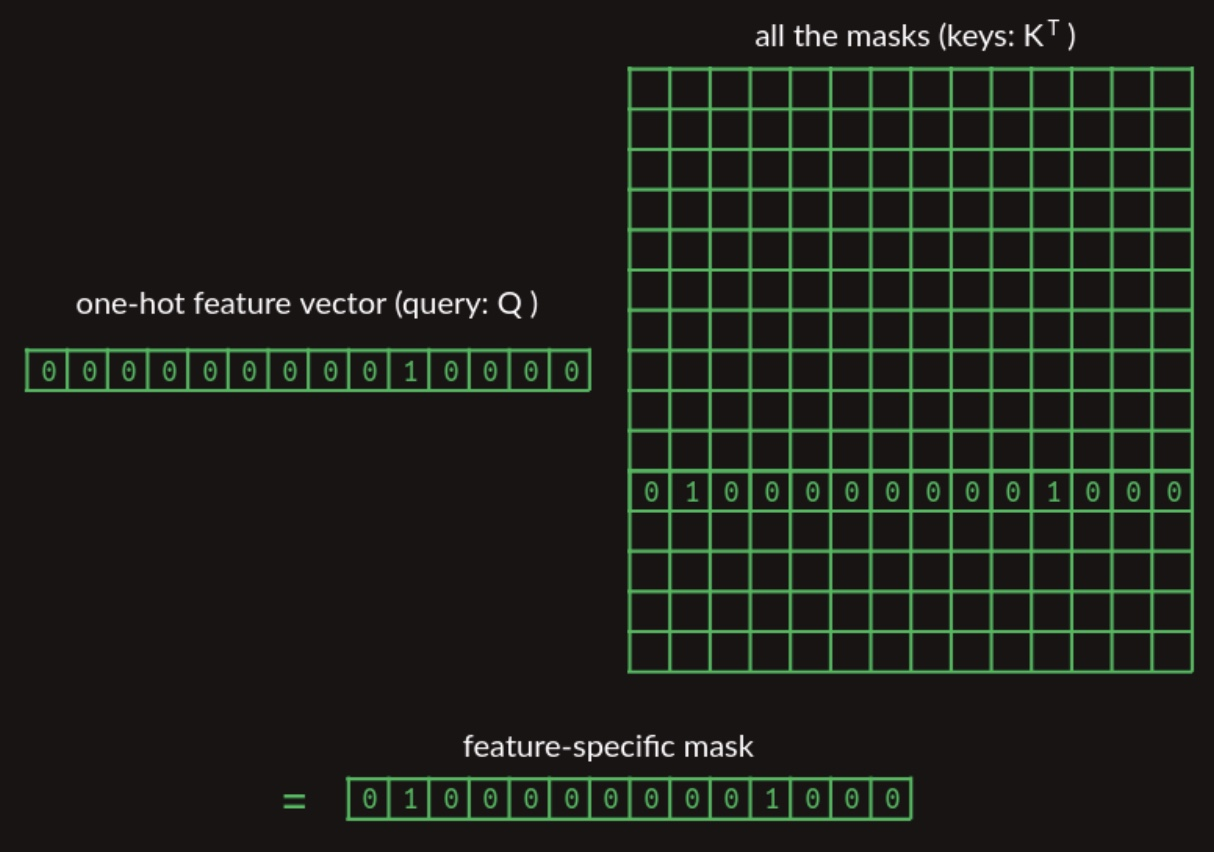• In the matrix showing the collection of mask vectors, we’ve only shown the one we’re trying to pull out, for clarity.
• We’re finally getting to the point where we can start tying into the paper. This mask lookup is represented by the $$QK^T$$ term in the attention equation (below), described in the details are discussed in the section on Single Head Attention Revisited.
$\operatorname{Attention}(Q, K, V)=\operatorname{softmax}\left(\frac{Q K^{T}}{\sqrt{d_{k}}}\right) V$
• The query $$Q$$ represents the feature of interest and the matrix $$K$$ represents the collection of masks. Because it’s stored with masks in columns, rather than rows, it needs to be transposed (with the $$T$$ operator) before multiplying. By the time we’re all done, we’ll make some important modifications to this, but at this level it captures the concept of a differentiable lookup table that transformers make use of.
• More in the section on Attention below.

#### Second order sequence model as matrix multiplications

• Another step that we have been hand wavy about so far is the construction of transition matrices. We have been clear about the logic, but not about how to do it with matrix multiplications.

• Once we have the result of our attention step, a vector that includes the most recent word and a small collection of the words that have preceded it, we need to translate that into features, each of which is a word pair. Attention masking gets us the raw material that we need, but it doesn’t build those word pair features. To do that, we can use a single layer fully connected neural network.

• To see how a neural network layer can create these pairs, we’ll hand craft one. It will be artificially clean and stylized, and its weights will bear no resemblance to the weights in practice, but it will demonstrate how the neural network has the expressivity necessary to build these two word pair features. To keep it small and clean, will focus on just the three attended words from this example, battery, program, ran. The following diagram from Brandon Rohrer’s Transformers From Scratch shows a neural network layer for creating multi-word features.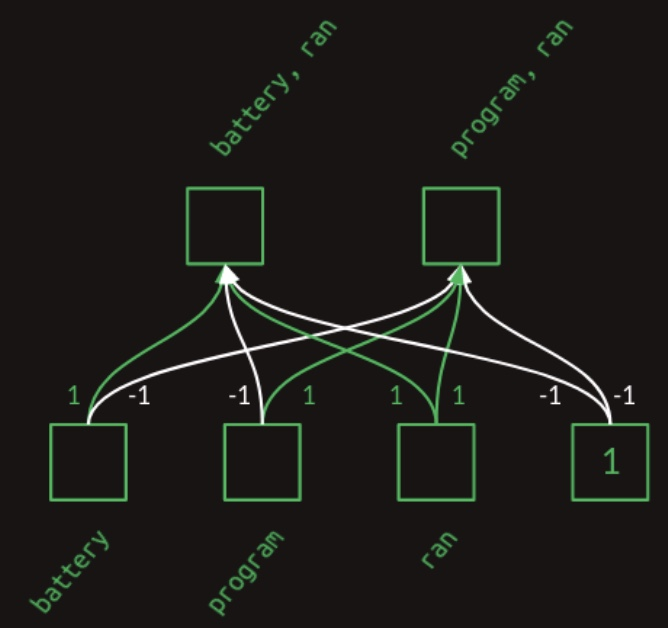• In the layer diagram above, we can see how the weights act to combine the presence and absence of each word into a collection of features. This can also be expressed in matrix form. The following diagram from Brandon Rohrer’s Transformers From Scratch shows a weight matrix for creating multi word features.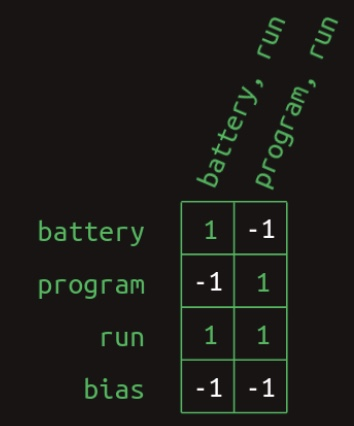• And it can be calculated by a matrix multiplication with a vector representing the collection of words seen so far. The following diagram from Brandon Rohrer’s Transformers From Scratch shows the calculation of the battery, ran feature.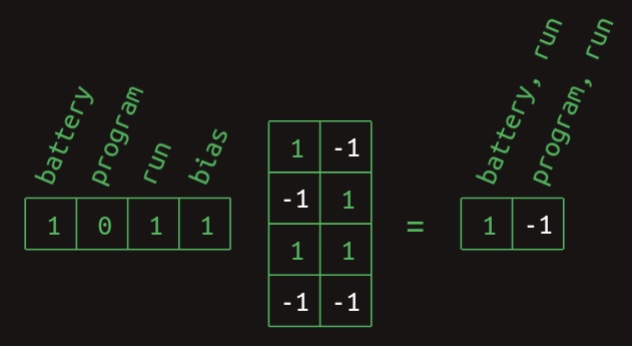• The battery and ran elements are 1 and the program element is 0. The bias element is always 1, a feature of neural networks. Working through the matrix multiplication gives a 1 for the element representing battery, ran and a -1 for the element representing program, ran. The results for the other case are similar. The following diagram from Brandon Rohrer’s Transformers From Scratch shows the calculation of the program, ran feature.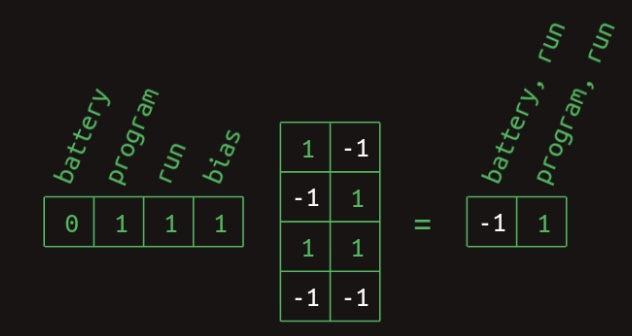• The final step in calculating these word combo features is to apply a rectified linear unit (ReLU) nonlinearity. The effect of this is to substitute any negative value with a zero. This cleans up both of these results so they represent the presence (with a 1) or absence (with a 0) of each word combination feature.

• With those gymnastics behind us, we finally have a matrix multiplication based method for creating multiword features. Although I originally claimed that these consist of the most recent word and one earlier word, a closer look at this method shows that it can build other features too. When the feature creation matrix is learned, rather than hard coded, other structures can be learned. Even in this toy example, there’s nothing to stop the creation of a three-word combination like battery, program, ran. If this combination occurred commonly enough it would probably end up being represented. There wouldn’t be any way to indicated what order the words occurred in (at least not yet), but we could absolutely use their co-occurrence to make predictions. It would even be possible to make use of word combos that ignored the most recent word, like battery, program. These and other types of features are probably created in practice, exposing the over-simiplification we made when we claimed that transformers are a selective-second-order-with-skips sequence model. There’s more nuance to it than that, and now you can see exactly what that nuance is. This won’t be the last time we’ll change the story to incorporate more subtlety.
• In this form, the multiword feature matrix is ready for one more matrix multiplication, the second order sequence model with skips we developed above. All together, the following sequence of feedforward processing steps get applied after attention is applied:
1. Feature creation matrix multiplication,
2. ReLU nonlinearity, and
3. Transition matrix multiplication
• The following equation from the paper shows the steps behind the Feed Forward block in a concise mathematical formulation.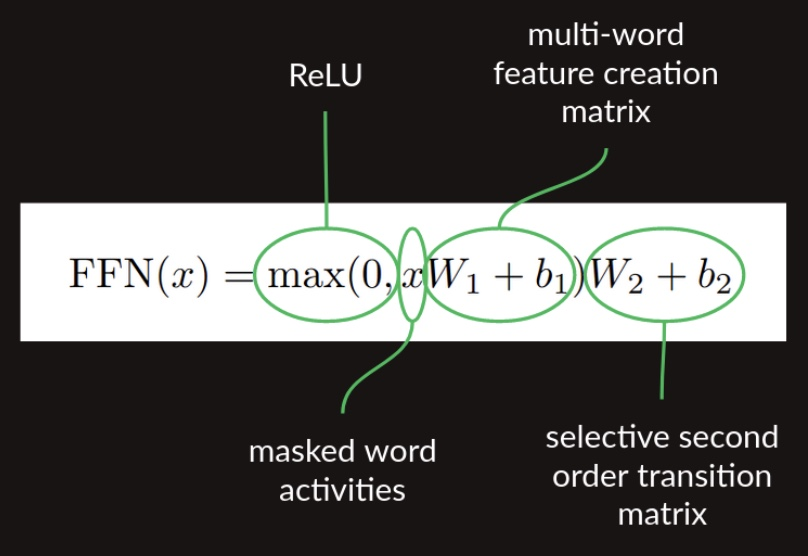• The architecture diagram (below) from the Transformers paper shows these lumped together as the Feed Forward block.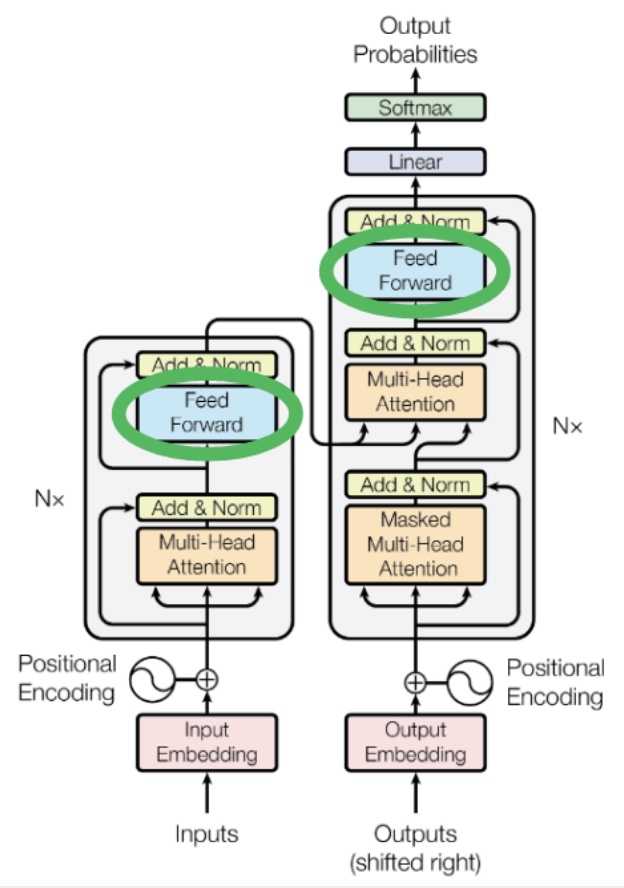#### Sampling a sequence of output words

##### Generating words as a probability distribution over the vocabulary
• So far we’ve only talked about next word prediction. There are a couple of pieces we need to add to get our decoder to generate a long sequence. The first is a prompt, some example text to give the transformer running start and context on which to build the rest of the sequence. It gets fed in to decoder, the column on the right in the image above, where it’s labeled “Outputs (shifted right)”. Choosing a prompt that gives interesting sequences is an art in itself, called prompt engineering. It’s also a great example of humans modifying their behavior to support algorithms, rather than the other way around.
• The decoder is fed a <START> token to generate the first word. The token serves as a signal to tell itself to start decoding using the compact representation of collected information from the encoder (more on this in the section on Cross-Attention). The following animation from Jay Alammar’s The Illustrated Transformer shows: (i) the process of parallel ingestion of tokens by the encoder (leading to the generation of key and value matrices from the last encoder layer), and (ii) the decoder producing the first token (the <START> token is missing from the animation).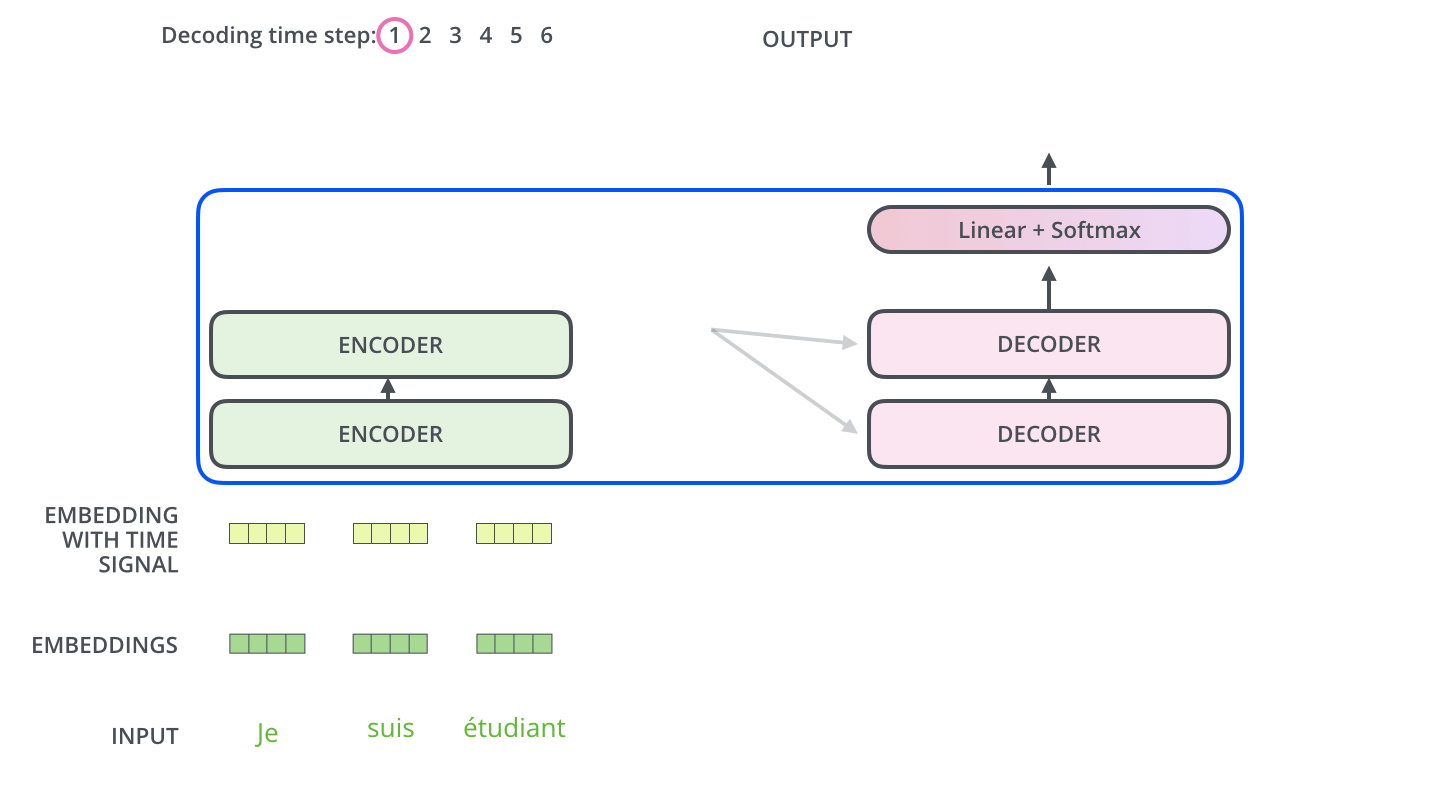• Once the decoder has a partial sequence in the form of a prompt (or the start token) to get started with, it takes a forward pass. The end result is a set of predicted probability distributions of words, one probability distribution for each position in the sequence. The process of de-embedding/decoding which involves going from a vector produced as the output of the decoder stack (bottom) to a series of logits (at the output of the linear layer) to a probability distribution (at the output of the softmax layer) and finally, to an output word as shown below in the below illustration from Jay Alammar’s The Illustrated Transformer.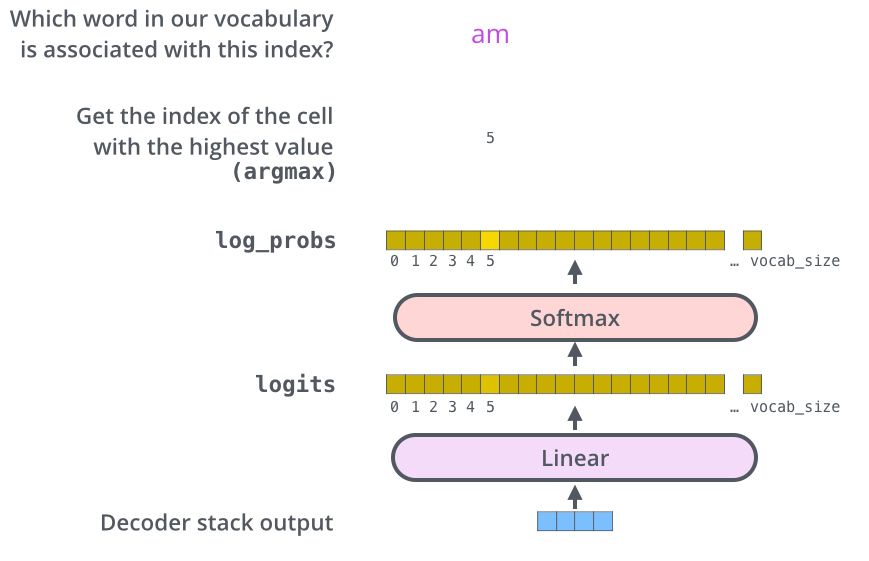##### Role of the Final Linear and Softmax Layer
• The linear layer is a simple fully connected layer that projects the vector produced by the stack of decoders, into a much, much larger vector called a logits vector.
• A typical NLP model knows about 40,000 unique English words (our model’s “output vocabulary”) that it’s learned from its training dataset. This would make the logits vector 40,000 dimensional, with each cell corresponding to the score of a unique word. That is how we interpret the output of the model followed by the linear layer.
• The Softmax layer then turns those scores from (unnormalized) logits/energy values into (normalized) probabilities, which effectively imposes the following constraints on the output: (i) all values are non-negative, i.e, $$\in [0, 1]$$, and (ii) all values add up to 1.0.
• At each position, the distribution shows the predicted probabilities for each next word in the vocabulary. We don’t care about predicted probabilities for existing words in the sequence (since they’re already established) – what we really care about are the predicted probabilities for the next word after the end of the prompt.
• The cell with the highest probability is chosen (more on this in the section on Greedy Decoding), and the word associated with it is produced as the output for this time step.
##### Greedy Decoding
• There are several ways to go about choosing what that word should be, but the most straightforward is called greedy decoding, which involves picking the word with the highest probability.
• The new next word then gets added to the sequence and fed in as input to the decoder, and the process is repeated until you either reach an <EOS> token or once you sample a fixed number of tokens. The following animation from Jay Alammar’s The Illustrated Transformer shows the decoder auto-regressively generating the next token (by absorbing the previous tokens).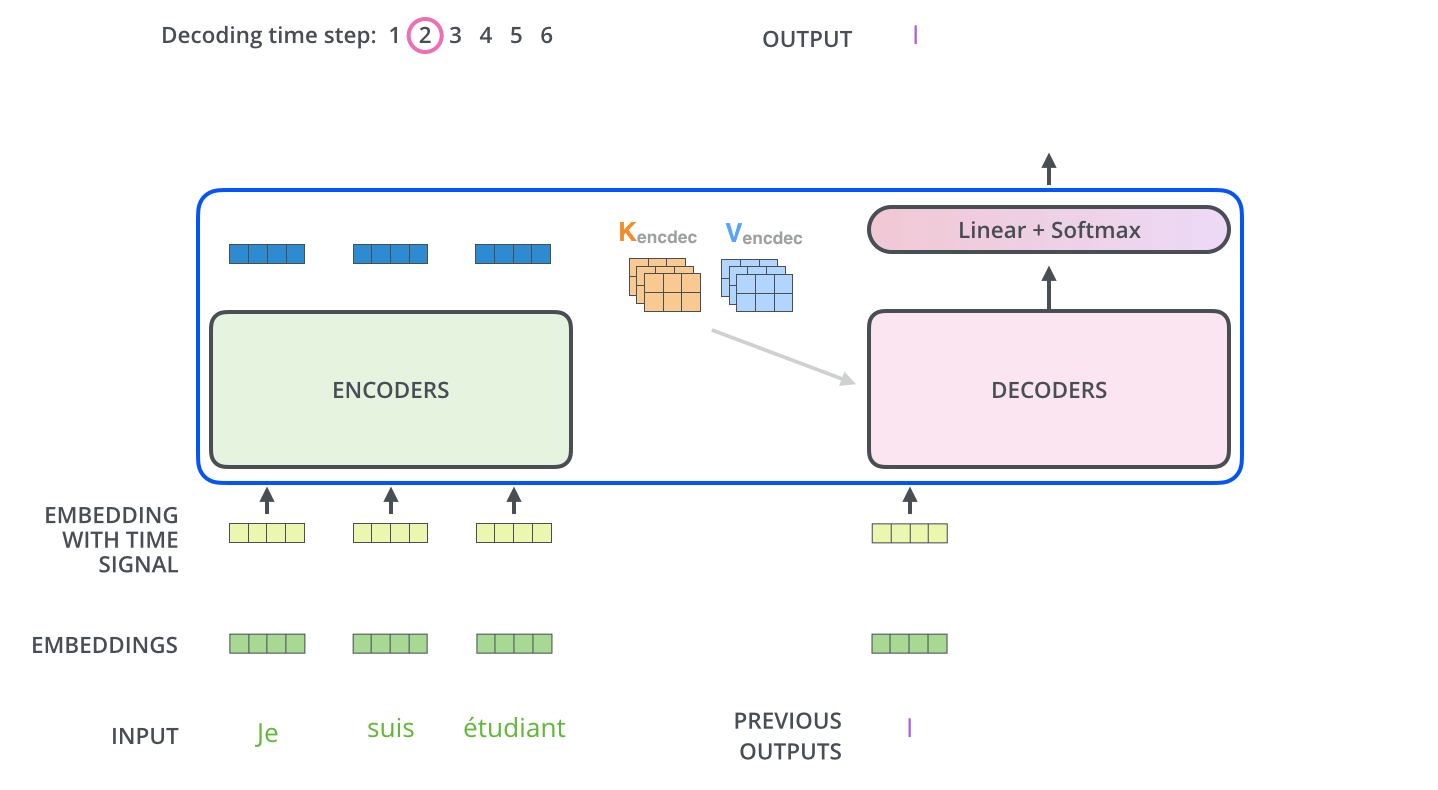• The one piece we’re not quite ready to describe in detail is yet another form of masking, ensuring that when the transformer makes predictions it only looks behind, not ahead. It’s applied in the block labeled “Masked Multi-Head Attention”. We’ll revisit this later in the section on Single Head Attention Revisited to understand how it is implemented.

### Transformer Core

#### Embeddings

• As we’ve described them so far, transformers are too big. For a vocabulary size N of say 50,000, the transition matrix between all pairs of words and all potential next words would have 50,000 columns and 50,000 squared (2.5 billion) rows, totaling over 100 trillion elements. That is still a stretch, even for modern hardware.
• It’s not just the size of the matrices that’s the problem. In order to build a stable transition language model, we would have to provide training data illustrating every potential sequence several times at least. That would far exceed the capacity of even the most ambitious training data sets.
• Fortunately, there is a workaround for both of these problems, embeddings.
• In a one-hot representation of a language, there is one vector element for each word. For a vocabulary of size $$N$$ that vector is an N-dimensional space. Each word represents a point in that space, one unit away from the origin along one of the many axes. A crude representation of a high dimensional space is as below.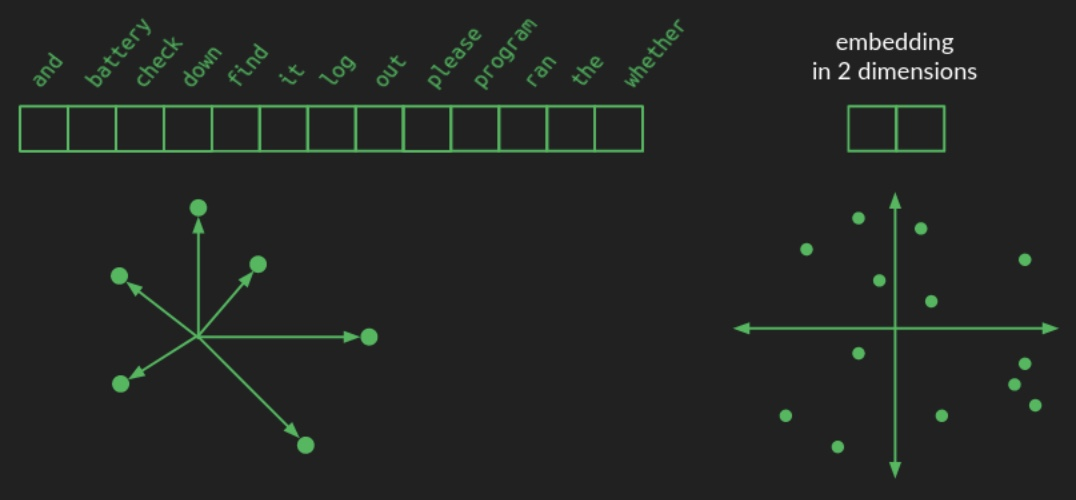• In an embedding, those word points are all taken and rearranged into a lower-dimensional space. In linear algebra terminology, this refers to the projecting data points. The picture above shows what they might look like in a 2-dimensional space for example. Now, instead of needing N numbers to specify a word, we only need 2. These are the $$(x, y)$$ coordinates of each point in the new space. Here’s what a 2-dimensional embedding might look like for our toy example, together with the coordinates of a few of the words.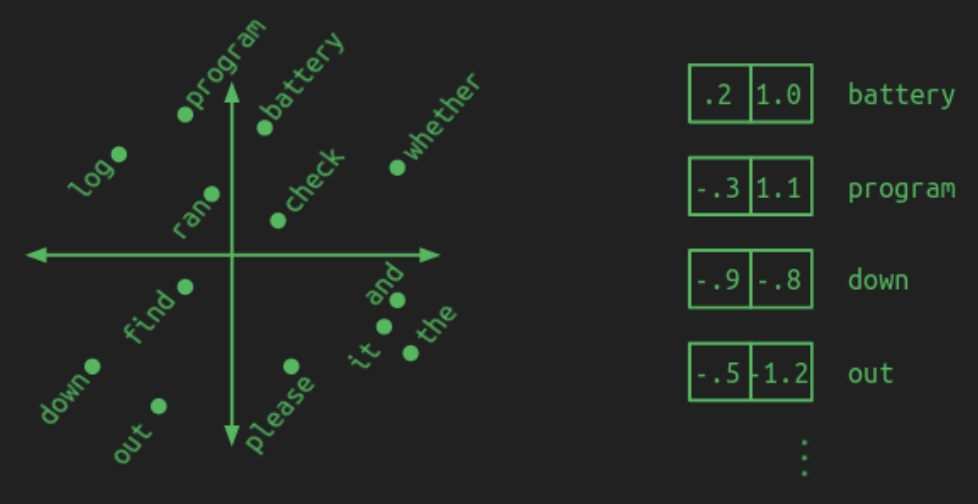• A good embedding groups words with similar meanings together. A model that works with an embedding learns patterns in the embedded space. That means that whatever it learns to do with one word automatically gets applied to all the words right next to it. This has the added benefit of reducing the amount of training data needed. Each example gives a little bit of learning that gets applied across a whole neighborhood of words.

• The illustration shows that by putting important components in one area (battery, log, program), prepositions in another (down, out), and verbs near the center (check, find, ran). In an actual embedding the groupings may not be so clear or intuitive, but the underlying concept is the same. Distance is small between words that behave similarly.

• An embedding reduces the number of parameters needed by a tremendous amount. However, the fewer the dimensions in the embedded space, the more information about the original words gets discarded. The richness of a language still requires quite a bit of space to lay out all the important concepts so that they don’t step on each other’s toes. By choosing the size of the embedded space, we get to trade off computational load for model accuracy.

• It will probably not surprise you to learn that projecting words from their one-hot representation to an embedded space involves a matrix multiplication. Projection is what matrices do best. Starting with a one-hot matrix that has one row and $$N$$ columns, and moving to an embedded space of two dimensions, the projection matrix will have $$N$$ rows and two columns, as shown here. The following diagram from Brandon Rohrer’s Transformers From Scratch shows a projection matrix describing an embedding.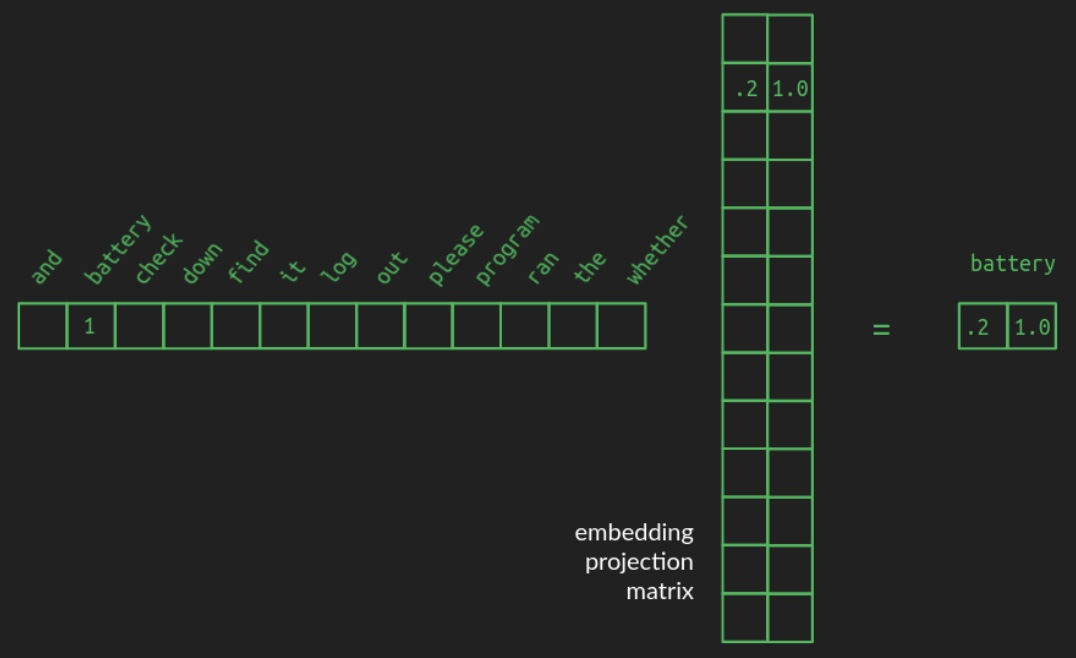• This example shows how a one-hot vector, representing for example battery, pulls out the row associated with it, which contains the coordinates of the word in the embedded space. In order to make the relationship clearer, the zeros in the one-hot vector are hidden, as are all the other rows that don’t get pulled out of the projection matrix. The full projection matrix is dense, each row containing the coordinates of the word it’s associated with.

• Projection matrices can convert the original collection of one-hot vocabulary vectors into any configuration in a space of whatever dimensionality you want. The biggest trick is finding a useful projection, one that has similar words grouped together, and one that has enough dimensions to spread them out. There are some decent pre-computed embeddings for common langauges, like English. Also, like everything else in the transformer, it can be learned during training.

• The architecture diagram from the Transformers paper shows where the embeddings are generated: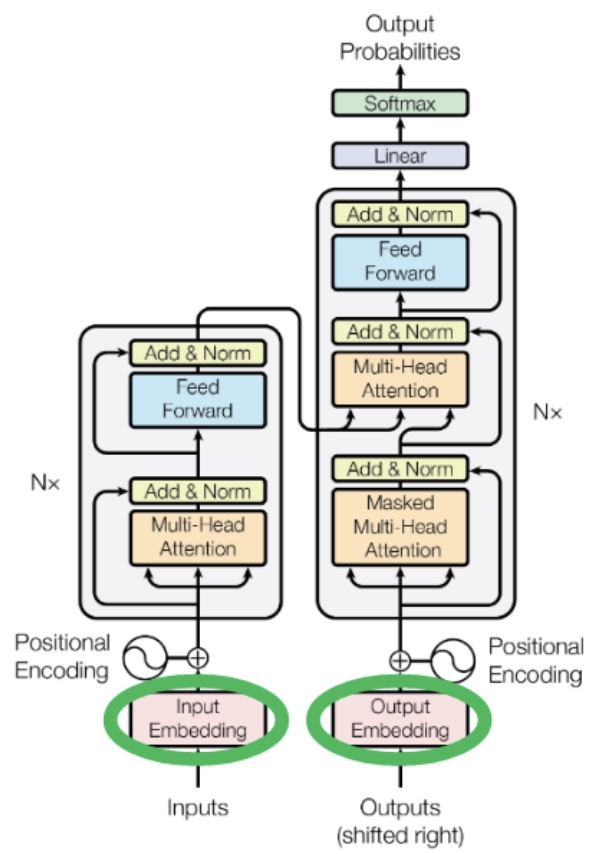#### Positional encoding

In contrast to recurrent and convolutional neural networks, the Transformer architecture does not explicitly model relative or absolute position information in its structure.

• Up to this point, we’ve assumed that the positions of words are ignored, at least for any words coming before the very most recent word. Now we get to fix that using positional embeddings, which offer a gateway to embed spatial information as an input to the transformer.

• There are several ways that position information could be introduced into our embedded representation of words, but the way it was done in the original transformer was to add a circular wiggle by using sinusoidal positional embeddings. Newer positional encoding schemes that utilize sophisticated schemes such as Rotary Position Embeddings, which encode absolute positional information with a rotation matrix and naturally incorporate explicit relative position dependency in the self-attention formulation, have been recently proposed.
• The following diagram from Brandon Rohrer’s Transformers From Scratch shows that positional encoding introduces a circular wiggle, owing to the addition of sinusoidal positional embeddings: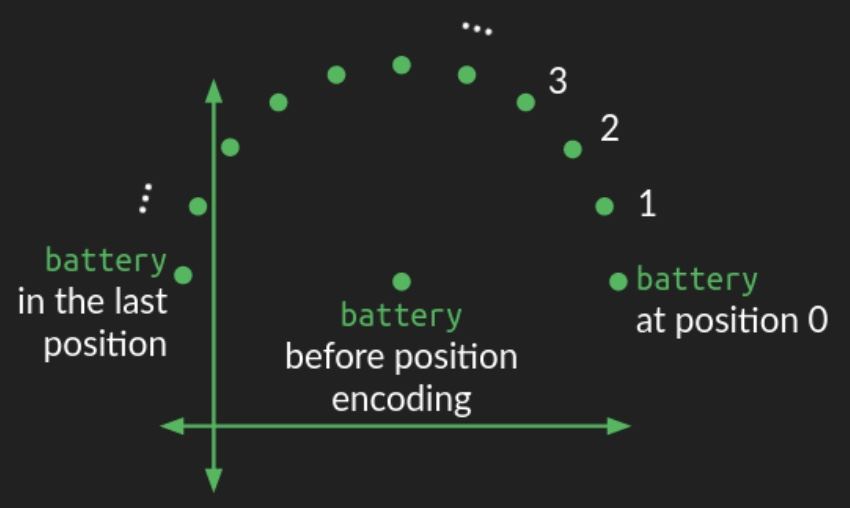• The position of the word in the embedding space acts as the center of a circle. A perturbation is added to it, depending on where it falls in the order of the sequence of words. For each position, the word is moved the same distance but at a different angle, resulting in a circular pattern as you move through the sequence. Words that are close to each other in the sequence have similar perturbations, but words that are far apart are perturbed in different directions.

• Since a circle is a two dimensional figure, representing a circular wiggle requires modifying two dimensions of the embedding space. If the embedding space consists of more than two dimensions (which it almost always does), the circular wiggle is repeated in all the other pairs of dimensions, but with different angular frequency, that is, it sweeps out a different number of rotations in each case. In some dimension pairs, the wiggle will sweep out many rotations of the circle. In other pairs, it will only sweep out a small fraction of a rotation. The combination of all these circular wiggles of different frequencies gives a good representation of the absolute position of a word within the sequence.

• In the architecture diagram from the Transformers paper, these blocks show the generation of the position encoding and its addition to the embedded words: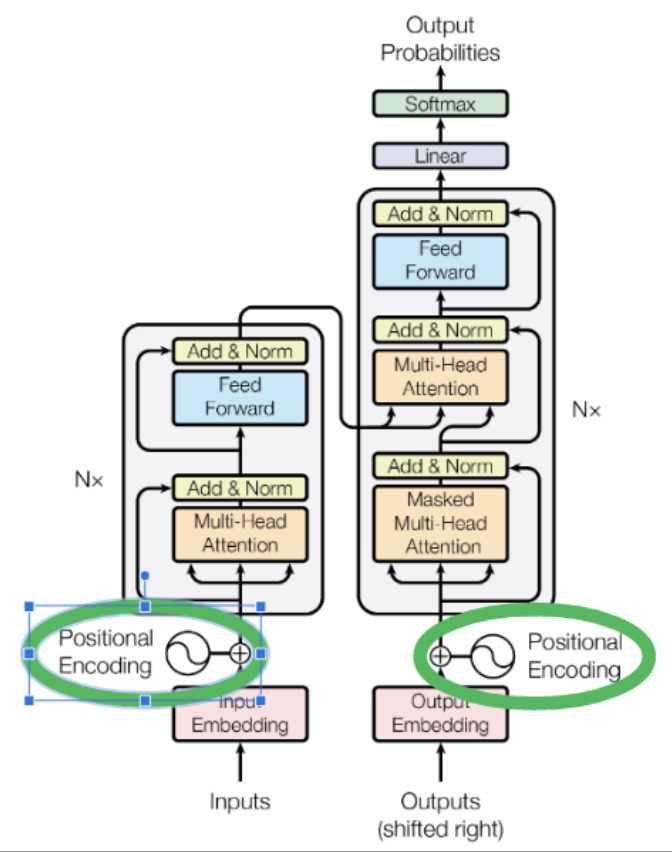#### Decoding output words / De-embeddings

• Embedding words makes them vastly more efficient to work with, but once the party is over, they need to be converted back to words from the original vocabulary. De-embedding is done the same way embeddings are done, with a projection from one space to another, that is, a matrix multiplication.

• The de-embedding matrix is the same shape as the embedding matrix, but with the number of rows and columns flipped. The number of rows is the dimensionality of the space we’re converting from. In the example we’ve been using, it’s the size of our embedding space, two. The number of columns is the dimensionality of the space we’re converting to — the size of the one-hot representation of the full vocabulary, 13 in our example. The following diagram shows the de-embedding transform: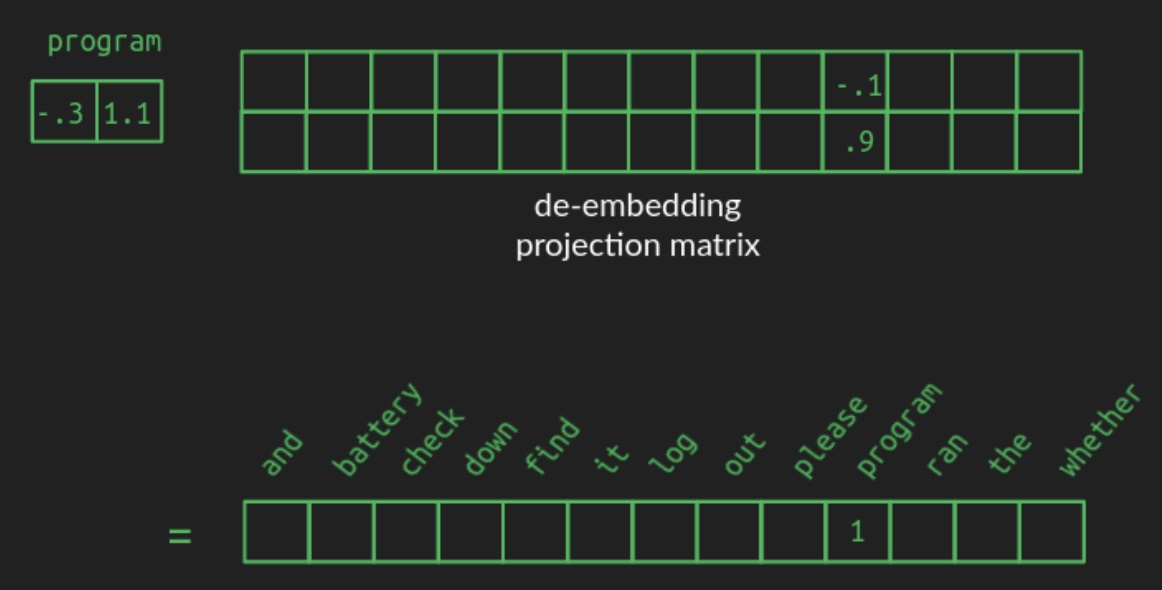• The values in a good de-embedding matrix aren’t as straightforward to illustrate as those from the embedding matrix, but the effect is similar. When an embedded vector representing, say, the word program is multiplied by the de-embedding matrix, the value in the corresponding position is high. However, because of how projection to higher dimensional spaces works, the values associated with the other words won’t be zero. The words closest to program in the embedded space will also have medium-high values. Other words will have near zero value. And there will likely be a lot of words with negative values. The output vector in vocabulary space will no longer be one-hot or sparse. It will be dense, with nearly all values non-zero. The following diagram shows the representative dense result vector from de-embedding: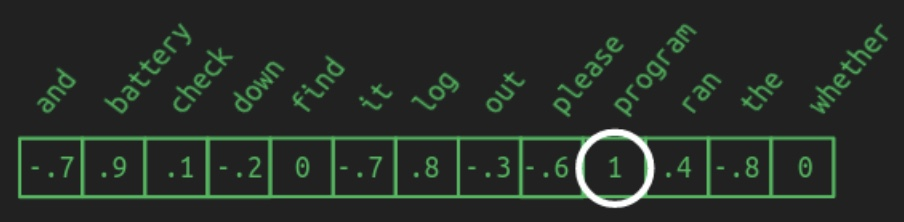• We can recreate the one-hot vector by choosing the word associated with the highest value. This operation is also called argmax, the argument (element) that gives the maximum value. This is how to do greedy sequence completion, as mentioned in the section on sampling a sequence of output words. It’s a great first pass, but we can do better.

• If an embedding maps very well to several words, we might not want to choose the best one every time. It might be only a tiny bit better choice than the others, and adding a touch of variety can make the result more interesting. Also, sometimes it’s useful to look several words ahead and consider all the directions the sentence might go before settling on a final choice. In order to do these, we have to first convert our de-embedding results to a probability distribution.

#### Attention

• Now that we’ve made peace with the concepts of projections (matrix multiplications) and spaces (vector sizes), we can revisit the core attention mechanism with renewed vigor. It will help clarify the algorithm if we can be more specific about the shape of our matrices at each stage. There is a short list of important numbers for this.
• $$N$$: vocabulary size; 13 in our example. Typically in the tens of thousands.
• $$n$$: maximum sequence length; 12 in our example. Something like a few hundred in the paper (they don’t specify.) 2048 in GPT-3.
• $$d_{model}$$: number of dimensions in the embedding space used throughout the model (512 in the paper).
• The original input matrix is constructed by getting each of the words from the sentence in their one-hot representation, and stacking them such that each of the one-hot vectors is its own row. The resulting input matrix has $$n$$ rows and $$N$$ columns, which we can abbreviate as $$[n \times N]$$.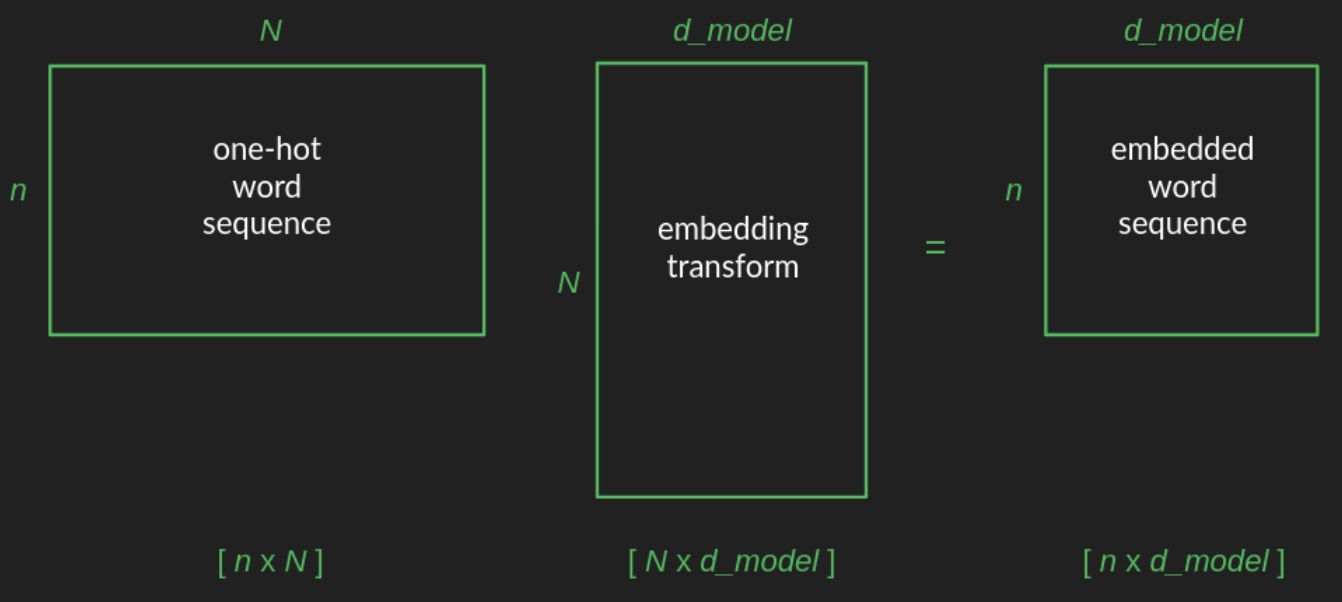• As we illustrated before, the embedding matrix has $$N$$ rows and $$d_{model}$$ columns, which we can abbreviate as $$[N \times d_{model}]$$. When multiplying two matrices, the result takes its number of rows from the first matrix, and its number of columns from the second. That gives the embedded word sequence matrix a shape of $$[n \times d_{model}]$$.
• We can follow the changes in matrix shape through the transformer as a way to track what’s going on (c.f. figure below; source). After the initial embedding, the positional encoding is additive, rather than a multiplication, so it doesn’t change the shape of things. Then the embedded word sequence goes into the attention layers, and comes out the other end in the same shape. (We’ll come back to the inner workings of these in a second.) Finally, the de-embedding restores the matrix to its original shape, offering a probability for every word in the vocabulary at every position in the sequence.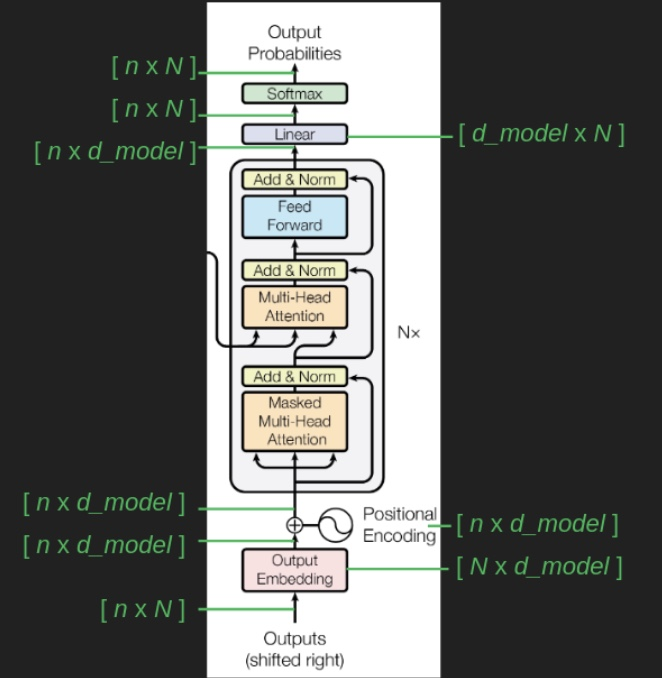##### Why attention? Contextualized Word Embeddings
###### History
• Bag of words was the first technique invented to create a machine-representation of text. By counting the frequency of words in a piece of text, one could extract its “characteristics”. The following table (source) shows an example of the data samples (reviews) per row and the vocabulary of the model (unique words) across columns.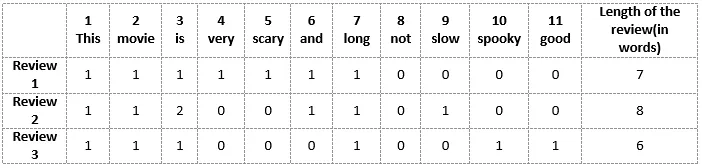• However, this suggests that when all words are considered equally important, significant words like “crisis” which carry important meaning in the text can be drowned out by insignificant words like “and”, “for”, or “the” which add little information but are commonly used in all types of text.
• To address this issue, TF-IDF (Term Frequency-Inverse Document Frequency) assigns weights to each word based on its frequency across all documents. The more frequent the word is across all documents, the less weight it carries.
• However, this method is limited in that it treats each word independently and does not account for the fact that the meaning of a word is highly dependent on its context. As a result, it can be difficult to accurately capture the meaning of the text. This limitation was addressed with the use of deep learning techniques.
###### Enter Word2Vec: Neural Word Embeddings
• Word2Vec revolutionized embeddings by using a neural network to transform texts into vectors.
• Two popular approaches are the Continuous Bag of Words (CBOW) and Skip-gram models, which are trained using raw text data in an unsupervised manner. These models learn to predict the center word given context words or the context words given the center word, respectively. The resulting trained weights encode the meaning of each word relative to its context.
• The following figure (source) visualizes CBOW where the target word is predicted based on the context using a neural network: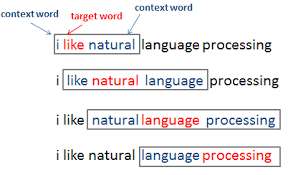• However, Word2Vec and similar techniques (such as GloVe, FastText, etc.) have their own limitations. After training, each word is assigned a unique embedding. Thus, polysemous words (i.e, words with multiple distinct meanings in different contexts) cannot be accurately encoded using this method. As an example:

“The man was accused of robbing a bank.” “The man went fishing by the bank of the river.”

• As another example:

“Time flies like an arrow.” “Fruit flies like a banana.”

• This limitation gave rise to contextualized word embeddings.
###### Contextualized Word Embeddings
• Transformers, owing to their self-attention mechanism, are able to encode a word using its context. This, in turn, offers the ability to learn contextualized word embeddings.
• Note that while Transformer-based architectures (e.g., BERT) learn contextualized word embeddings, prior work (ELMo) originally proposed this concept.
• As indicated in the prior section, contextualized word embeddings help distinguish between multiple meanings of the same word, in case of polysemous words.
• The process begins by encoding each word as an embedding (i.e., a vector that represents the word and that LLMs can operate with). A basic one is one-hot encoding, but we typically use embeddings that encode meaning (the Transformer architecture begins with a randomly-initialized nn.Embedding instance that is learnt during the course of training). However, note that the embeddings at this stage are non-contextual, i.e., they are fixed per word and do not incorporate context surrounding the word.
• As we will see in the section on Single Head Attention Revisited, self-attention transforms the embedding to a weighted combination of the embeddings of all the other words in the text. This represents the contextualized embedding that packs in the context surrounding the word.
• Considering the example of the word bank above, the embedding for bank in the first sentence would have contributions (and would thus be influenced significantly) from words like “accused”, “robbing”, etc. while the one in the second sentence would utilize the embeddings for “fishing”, “river”, etc. In case of the word flies, the embedding for flies in the first sentence will have contributions from words like “go”, “soars”, “pass”, “fast”, etc. while the one in the second sentence would depend on contributions from “insect”, “bug”, etc.
• The following figure (source) shows an example for the word flies, and computing the new embeddings involves a linear combination of the representations of the other words, with the weight being proportional to the relationship (say, similarity) of other words compared to the current word. In other words, the output is computed as a weighted sum of the values, where the weight assigned to each value is computed by a compatibility function of the query with the corresponding key (also called the “alignment” function in Bengio’s original paper that introduced attention in the context of neural networks).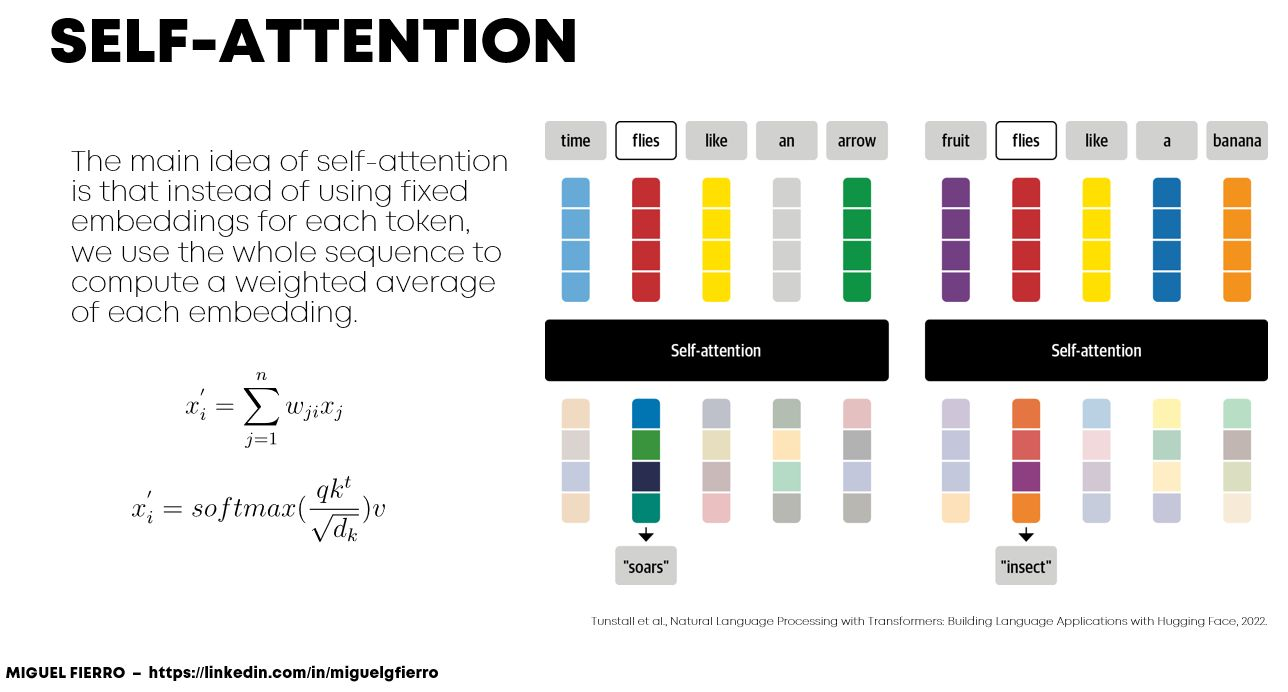##### Types of Attention: Additive, Multiplicative (Dot-product), and Scaled
• The Transformer is based on “Scaled Dot-Product Attention”.
• The two most commonly used attention functions are additive attention (Neural Machine Translation by Jointly Learning to Align and Translate), and dot-product (multiplicative) attention. Dot-product attention is identical to their algorithm, except for the scaling factor of $$\frac{1}{\sqrt{d_{k}}}$$. Additive attention computes the compatibility function using a feed-forward network with a single hidden layer. While the two are similar in theoretical complexity, dot-product attention is much faster and more space-efficient in practice, since it can be implemented using highly optimized matrix multiplication code.
• While for small values of $$d_{k}$$ the two mechanisms perform similarly, additive attention outperforms dot product attention without scaling for larger values of $$d_{k}$$ (Massive Exploration of Neural Machine Translation Architectures). We suspect that for large values of $$d_{k}$$, the dot products grow large in magnitude, pushing the softmax function into regions where it has extremely small gradients (To illustrate why the dot products get large, assume that the components of $$q$$ and $$k$$ are independent random variables with mean 0 and variance 1. Then their dot product, $$q \cdot k=\sum_{i=1}^{d_{k}} q_{i} k_{i}$$, has mean 0 and variance $$d_{k}$$.). To counteract this effect, we scale the dot products by $$\frac{1}{\sqrt{d_{k}}}$$.
##### Attention calculation
• Let’s develop an intuition about the architecture using the language of mathematical symbols and vectors.
• We update the hidden feature $$h$$ of the $$i^{th}$$ word in a sentence $$\mathcal{S}$$ from layer $$\ell$$ to layer $$\ell+1$$ as follows:

$h_{i}^{\ell+1}=\text { Attention }\left(Q^{\ell} h_{i}^{\ell}, K^{\ell} h_{j}^{\ell}, V^{\ell} h_{j}^{\ell}\right)$
• i.e.,
$\begin{array}{c} h_{i}^{\ell+1}=\sum_{j \in \mathcal{S}} w_{i j}\left(V^{\ell} h_{j}^{\ell}\right) \\ \text { where } w_{i j}=\operatorname{softmax}_{j}\left(Q^{\ell} h_{i}^{\ell} \cdot K^{\ell} h_{j}^{\ell}\right) \end{array}$
• where $$j \in \mathcal{S}$$ denotes the set of words in the sentence and $$Q^{\ell}, K^{\ell}, V^{\ell}$$ are learnable linear weights (denoting the Query, Key and Value for the attention computation, respectively).
• From Eugene Yan’s Some Intuition on Attention and the Transformer blog, to build intuition around the concept of attention, let’s draw a parallel from a real life scenario and reason about the concept of key-value attention:

Imagine yourself in a library. You have a specific question (query). Books on the shelves have titles on their spines (keys) that suggest their content. You compare your question to these titles to decide how relevant each book is, and how much attention to give each book. Then, you get the information (value) from the relevant books to answer your question.

• Since the queries, keys, and values are all drawn from the same source, we refer to this attention as self-attention (we use “attention” and “self-attention” interchangeably in this topic). Self-attention forms the core component of Transformers. Also, given the use of the dot-product to ascertain similarity between the query and key vectors, the attention mechanism is also called dot-product self-attention.
• Note that one of the benefits of self-attention over recurrence is that it’s highly parallelizable. In other words, the attention mechanism is performed in parallel for each word in the sentence to obtain their updated features in one shot. This is a big advantage for Transformers over RNNs, which update features word-by-word. In other words, Transformer-based deep learning models don’t require sequential data to be processed in order, allowing for much more parallelization and reduced training time on GPUs than RNNs.
• We can understand the attention mechanism better through the following pipeline (source):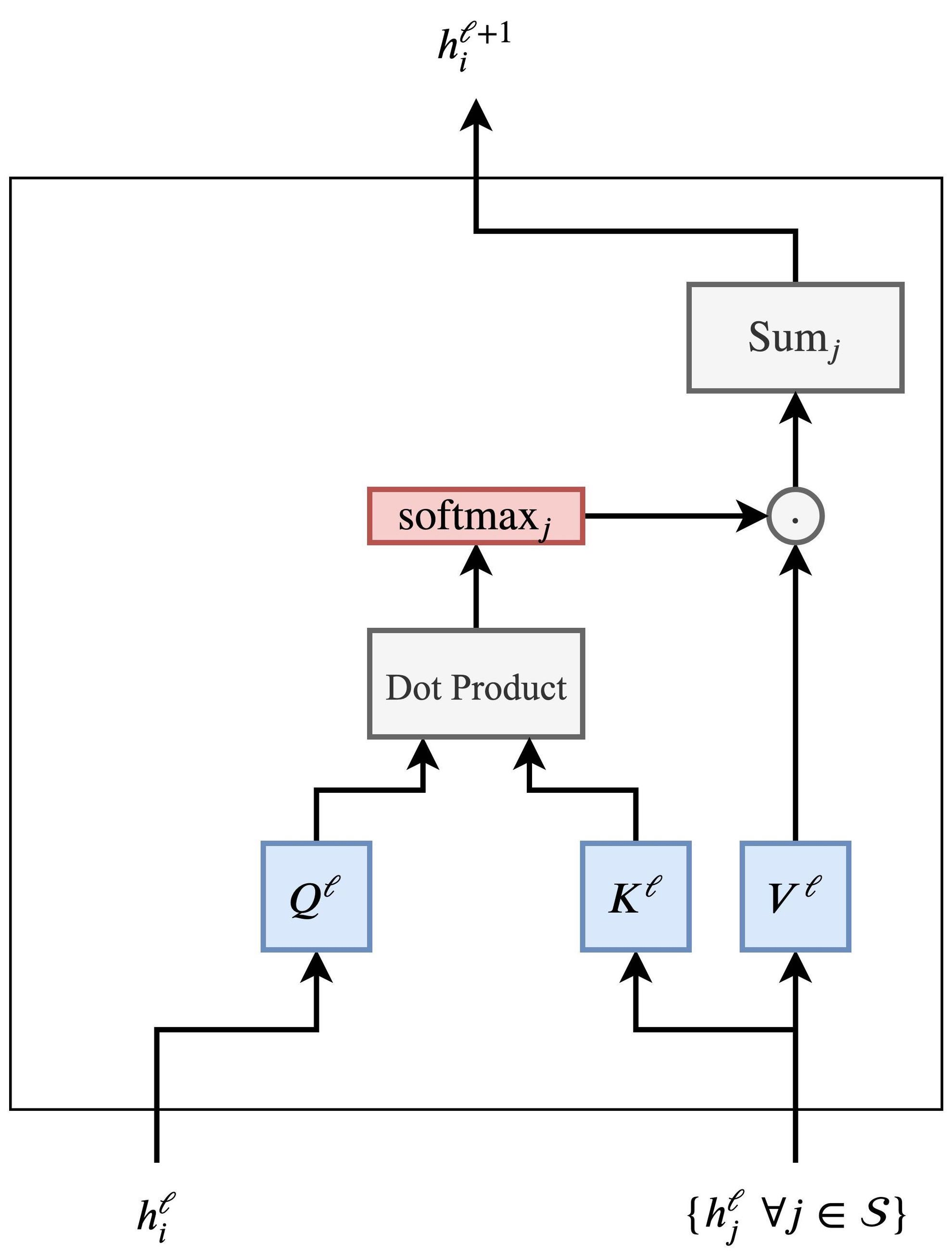• Taking in the features of the word $$h_{i}^{\ell}$$ and the set of other words in the sentence $$\left\{h_{j}^{\ell} \forall j \in \mathcal{S}\right\}$$, we compute the attention weights $$w_{i j}$$ for each pair $$(i, j)$$ through the dot-product, followed by a softmax across all $$j$$’s.
• Finally, we produce the updated word feature $$h_{i}^{\ell+1}$$ for word $$i$$ by summing over all $$\left\{h_{j}^{\ell}\right\}$$’s weighted by their corresponding $$w_{i j}$$. Each word in the sentence parallelly undergoes the same pipeline to update its features.
• We already walked through a conceptual illustration of attention in Attention As Matrix Multiplication above. The actual implementation is a little messier, but our earlier intuition is still helpful. The queries and the keys are no longer easy to inspect and interpret because they are all projected down onto their own idiosyncratic subspaces. In our conceptual illustration, one row in the queries matrix represents one point in the vocabulary space, which, thanks the one-hot representation, represents one and only one word. In their embedded form, one row in the queries matrix represents one point in the embedded space, which will be near a group of words with similar meanings and usage. The conceptual illustration mapped one query word to a set of keys, which in turn filtered out all the values that are not being attended to. Each attention head in the actual implempentation maps a query word to a point in yet another lower-dimensional embedded space. The result of this that that attention becomes a relationship between word groups, rather than between individual words. It takes advantage of semantic similarities (closeness in the embedded space) to generalize what it has learned about similar words.

• Following the shape of the matrices through the attention calculation helps to track what it’s doing (source):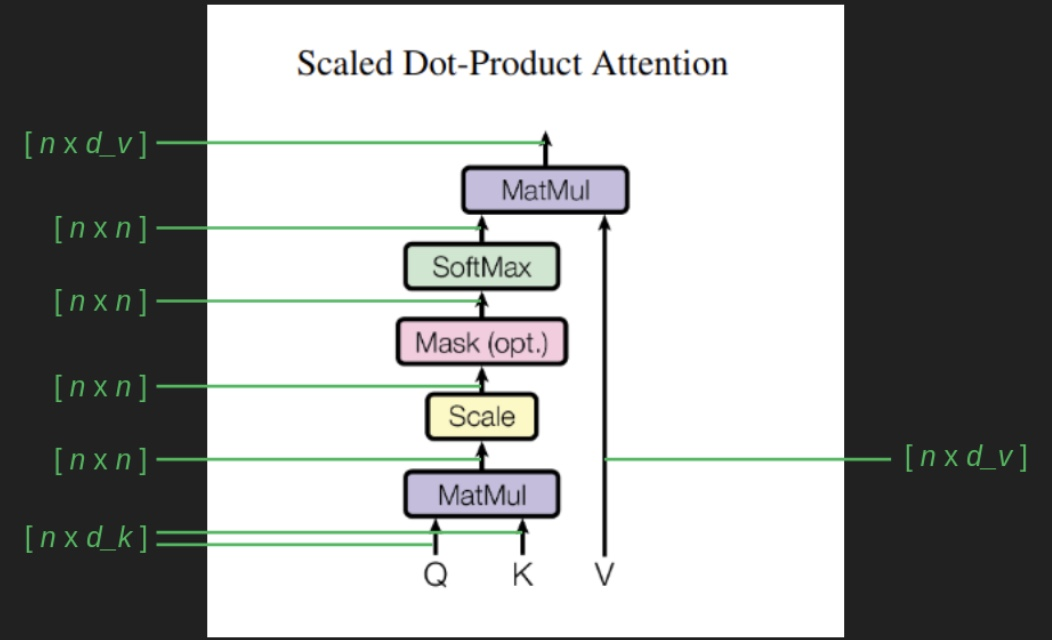• The queries and keys matrices, $$Q$$ and $$K$$, both come in with shape $$[n \times d_k]$$. Thanks to $$K$$ being transposed before multiplication, the result of $$Q K^T$$ gives a matrix of $$[n \times d_k] * [d_k \times n] = [n \times n]$$. Dividing every element of this matrix by the square root of $$d_k$$ has been shown to keep the magnitude of the values from growing wildly, and helps backpropagation to perform well. The softmax, as we mentioned, shoehorns the result into an approximation of an argmax, tending to focus attention one element of the sequence more than the rest. In this form, the $$[n \times n]$$ attention matrix roughly maps each element of the sequence to one other element of the sequence, indicating what it should be watching in order to get the most relevant context for predicting the next element. It is a filter that finally gets applied to the values matrix $$V$$, leaving only a collection of the attended values. This has the effect of ignoring the vast majority of what came before in the sequence, and shines a spotlight on the one prior element that is most useful to be aware of.
$\operatorname{Attention}(Q, K, V)=\operatorname{softmax}\left(\frac{Q K^{T}}{\sqrt{d_{k}}}\right) V$

The attention function can be described as mapping a query and a set of key-value pairs to an output, where the query, keys, values, and output are all vectors. The output is computed as a weighted sum of the values, where the weight assigned to each value is computed by a compatibility function of the query with the corresponding key (also called the “alignment” function in Bengio’s original paper that introduced attention in the context of neural networks).

• One tricky part about understanding this set of calculations is keeping in mind that it is calculating attention for every element of our input sequence, for every word in our sentence, not just the most recent word. It’s also calculating attention for earlier words. We don’t really care about these because their next words have already been predicted and established. It’s also calculating attention for future words. These don’t have much use yet, because they are too far out and their immediate predecessors haven’t yet been chosen. But there are indirect paths through which these calculations can effect the attention for the most recent word, so we include them all. It’s just that when we get to the end and calculate word probabilities for every position in the sequence, we throw away most of them and only pay attention to the next word.

• The masking block enforces the constraint that, at least for this sequence completion task, we can’t look into the future. It avoids introducing any weird artifacts from imaginary future words. It is crude and effective – manually set the attention paid to all words past the current position to negative infinity to prevent attention to subsequent positions. In The Annotated Transformer, an immeasurably helpful companion to the paper showing line by line Python implementation, the mask matrix is visualized. Purple cells show where attention is disallowed. Each row corresponds to an element in the sequence. The first row is allowed to attend to itself (the first element), but to nothing after. The last row is allowed to attend to itself (the final element) and everything that comes before. The Mask is an $$[n \times n]$$ matrix. It is applied not with a matrix multiplication, but with a more straightforward element-by-element multiplication. This has the effect of manually going in to the attention matrix and setting all of the purple elements from the mask to negative infinity. The following diagram shows an attention mask for sequence completion (source):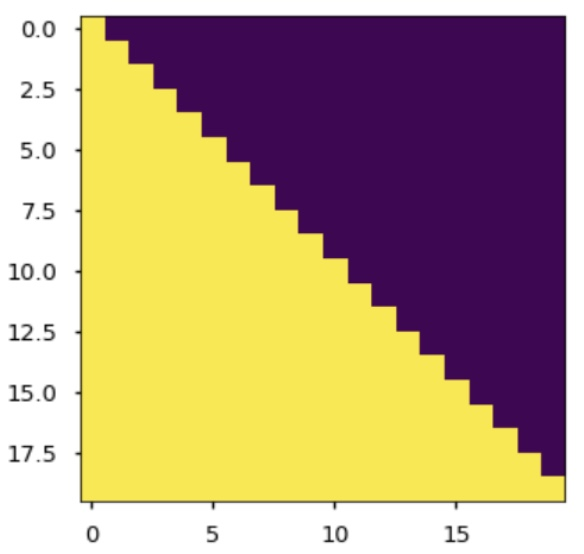• Another important difference in how attention is implemented is that it makes use of the order in which words are presented to it in the sequence, and represents attention not as a word-to-word relationship, but as a position-to-position relationship. This is evident in its $$[n \times n]$$ shape. It maps each element from the sequence, indicated by the row index, to some other element(s) of the sequence, indicated by the column index. This helps us to visualize and interpret what it is doing more easily, since it is operating in the embedding space. We are spared the extra step of finding nearby word in the embedding space to represent the relationships between queries and keys.
###### Putting it all together
• The following infographic (source) provides a quick overview of the constituent steps to calculate attention.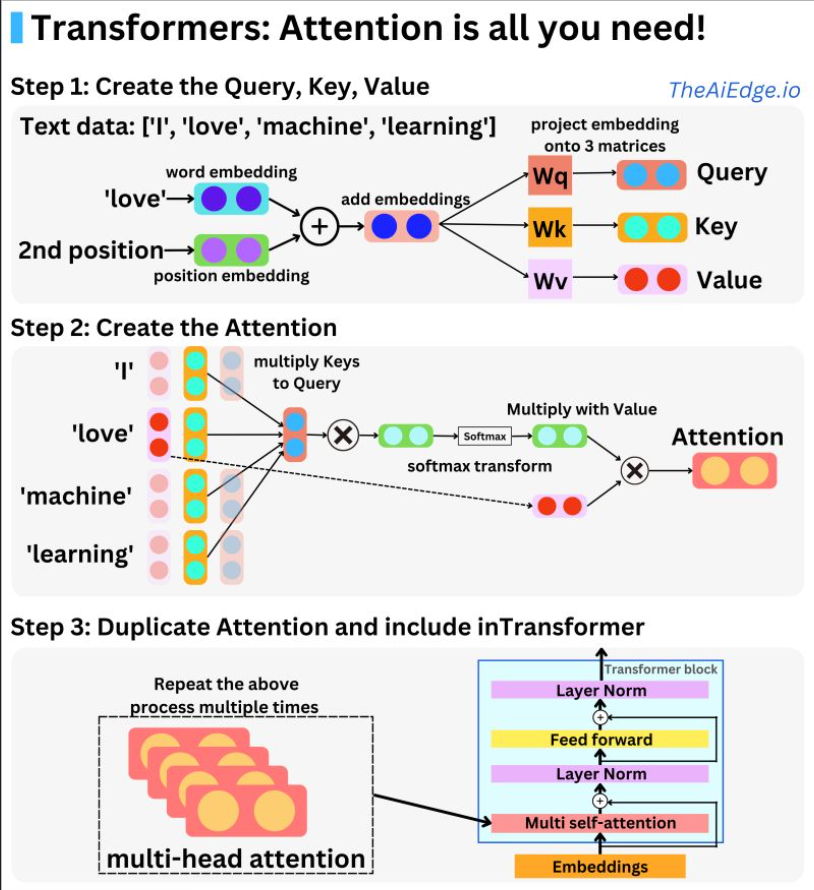• As indicated in the section on Contextualized Word Embeddings, Attention enables contextualized word embeddings by allowing the model to selectively focus on different parts of the input sequence when making predictions. Put simply, the attention mechanism allows the transformer to dynamically weigh the importance of different parts of the input sequence based on the current task and context.
• In an attention-based model like the transformer, the word embeddings are combined with attention weights that are learned during training. These weights indicate how much attention should be given to each word in the input sequence when making predictions. By dynamically adjusting the attention weights, the model can focus on different parts of the input sequence and better capture the context in which a word appears. As the paper states, the attention mechanism is what has revolutionized Transformers to what we see them to be today.
• Upon encoding a word as an embedding vector, we can also encode the position of that word in the input sentence as a vector (positional embeddings), and add it to the word embedding. This way, the same word at a different position in a sentence is encoded differently.
• The attention mechanism works with the inclusion of three vectors: key, query, value. Attention is the mapping between a query and a set of key-value pairs to an output. We start off by taking a dot product of query and key vectors to understand how similar they are. Next, the Softmax function is used to normalize the similarities of the resulting query-key vectors. The output is computed as a weighted sum of the values, where the weight assigned to each value is computed by a compatibility function of the query with the corresponding key. (source)
• Thus, the basis behind the concept of attention is: “How much attention a word should pay to another word in the input to understand the meaning of the sentence?”
• As indicated in the section on Attention Calculation, one of the benefits of self-attention over recurrence is that it’s highly parallelizable. In other words, the attention mechanism is performed in parallel for each word in the sentence to obtain their updated features in one shot. Furthermore, learning long-term/long-range dependencies in sequences is another benefit.
##### Averaging is equivalent to uniform attention
• On a side note, it is worthwhile noting that the averaging operation is equivalent to uniform attention with the weights being all equal to $$\frac{1}{n}$$, where $$n$$ is the number of words in the input sequence. In other words, averaging is simply a special case of attention.
##### Activation Functions
• The transformer does not use an activation function following the multi-head attention layer, but does use the ReLU activation post the two fully-connected layers that form the feed-forward network.
• The reason behind this goes back to the purpose of self-attention. The measure between word-vectors is generally computed through cosine-similarity because in the dimensions word tokens exist, it’s highly unlikely for two words to be collinear even if they are trained to be closer in value if they are similar. However, two trained tokens will have higher cosine-similarity if they are semantically closer to each other than two completely unrelated words.
• This fact is exploited by the self-attention mechanism; after several of these matrix multiplications, the dissimilar words will zero out or become negative due to the dot product between them, and the similar words will stand out in the resulting matrix.
• Thus, self attention can be viewed as a weighted average, where less similar words become averaged out faster (toward the zero vector, on average), thereby achieving groupings of important and unimportant words (i.e. attention). The weighting happens through the dot product. If input vectors were normalized, the weights would be exactly the cosine similarities.
• The important thing to take into consideration is that within the self-attention mechanism, there are no inherent parameters; those linear operations are just there to capture the relationship between the different vectors by using the properties of the vectors used to represent them, leading to attention weights.
##### Calculating $$Q$$, $$K$$, and $$V$$ matrices in the Transformer architecture
• Each word is embedded into a vector of size 512 and is fed into the bottom-most encoder. The abstraction that is common to all the encoders is that they receive a list of vectors each of the size 512 – in the bottom encoder that would be the word embeddings, but in other encoders, it would be the output of the encoder that’s directly below. The size of this list is hyperparameter we can set – basically it would be the length of the longest sentence in our training dataset.
• In the self-attention layers, multiplying the input vector (which is the word embedding for the first block of the encoder/decoder stack, while the output of the previous block for subsequent blocks) by the attention weights matrix (which are the $$Q$$, $$K$$, and $$V$$ matrices stacked horizontally) and adding a bias vector afterwards results in a contcatenated key, value, and query vector for this token. This long vector is split to form the $$q$$, $$k$$, and $$v$$ vectors for this token (which actually respresent the concatenated output for multiple attention heads and is thus, further reshaped into $$q$$, $$k$$, and $$v$$ outputs for each attention head — more on this in the section on Multi-head Attention). From Jay Alammar’s: The Illustrated GPT-2: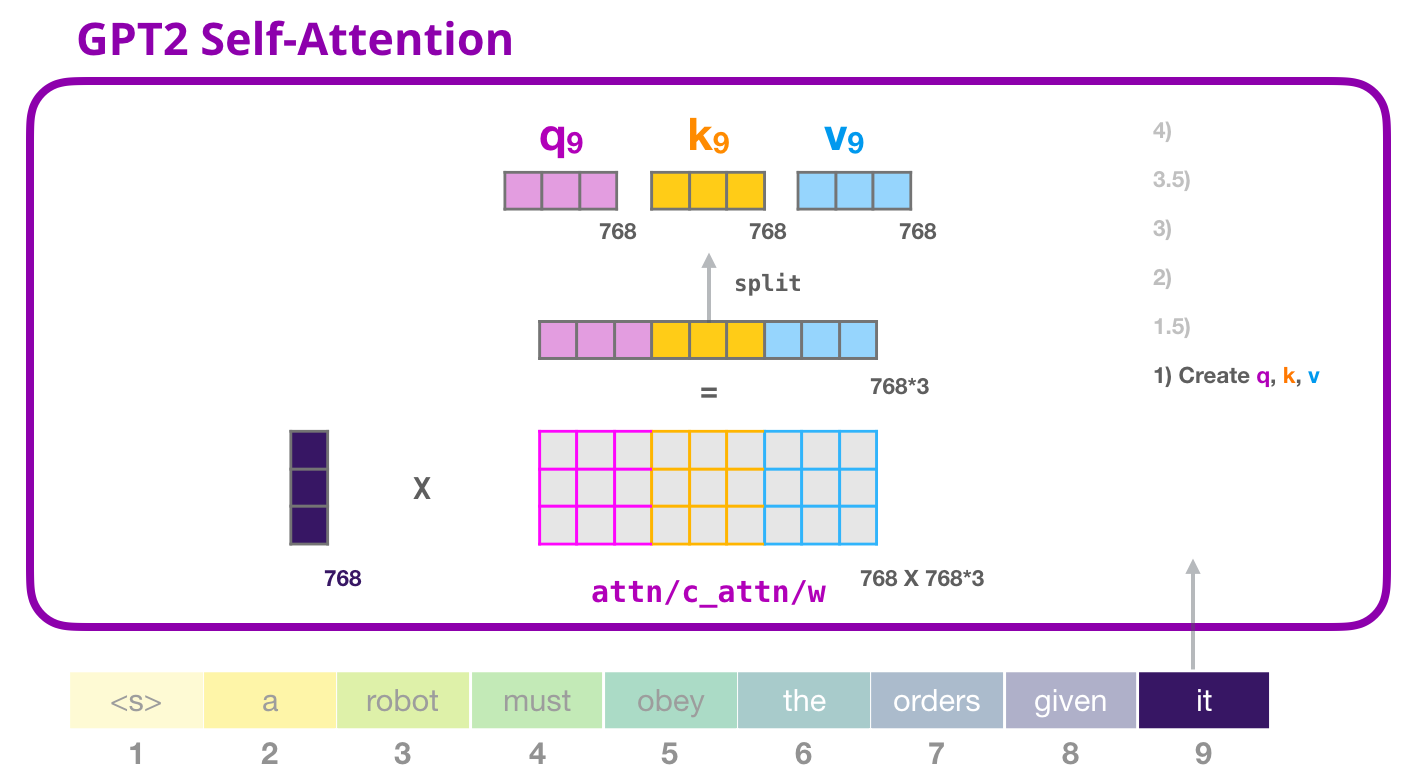##### Optimizing Performance with the KV Cache
• Using a KV cache is one of the most commonly-used tricks for speeding up inference with LLMs. Here’s exactly how it works.
• Autoregressive decoding process: When we perform inference with an LLM, it follows an autoregressive decoding process. Put simply, this means that we i) start with a sequence of textual tokens, ii) predict the next token, iii) add this token to our input, and iv) repeat until generation is finished.
• Causal self-attention: Self-attention within a language model is causal, meaning that each token only considers itself and prior tokens when computing its representation (i.e., NOT future tokens). As such, representations for each token do not change during autoregressive decoding! We need to compute the representation for each new token, but other tokens remain fixed (i.e., because they don’t depend on tokens that follow them).
• Caching self-attention values: When we perform self-attention, we project our sequence of tokens using three separate, linear projections: key projection, value projection, and query projection. Then, we executed self-attention using the resulting matrices! The KV-cache simply stores the results of the key and value projections for future decoding iterations so that we don’t recompute them every time!
• Why not cache the query? We might immediately be wondering why the key and value projections are cached, but not the query. This is simply because the rest of the entries in the query matrix are only needed to compute the representations of prior tokens in the sequence. For computing the representation of the most recently added token, we only need access to the most recent row in the query matrix.
• Updates to the KV cache: Throughout autoregressive decoding, we have the key and value projections cached. Each time we get a new token in our input, we simply compute the new rows as part of self-attention and add them to the KV cache. Then, we can use the query projection for the new token and the updated key and value projections to perform the rest of the forward pass.
• Important note: Here, we have considered single-headed self-attention for simplicity. However, it’s important to note that the same exact process applies to the multi-headed self-attention used by LLMs (detailed in the Multi-Head Attention section below). We just perform the exact same process in parallel across multiple attention heads.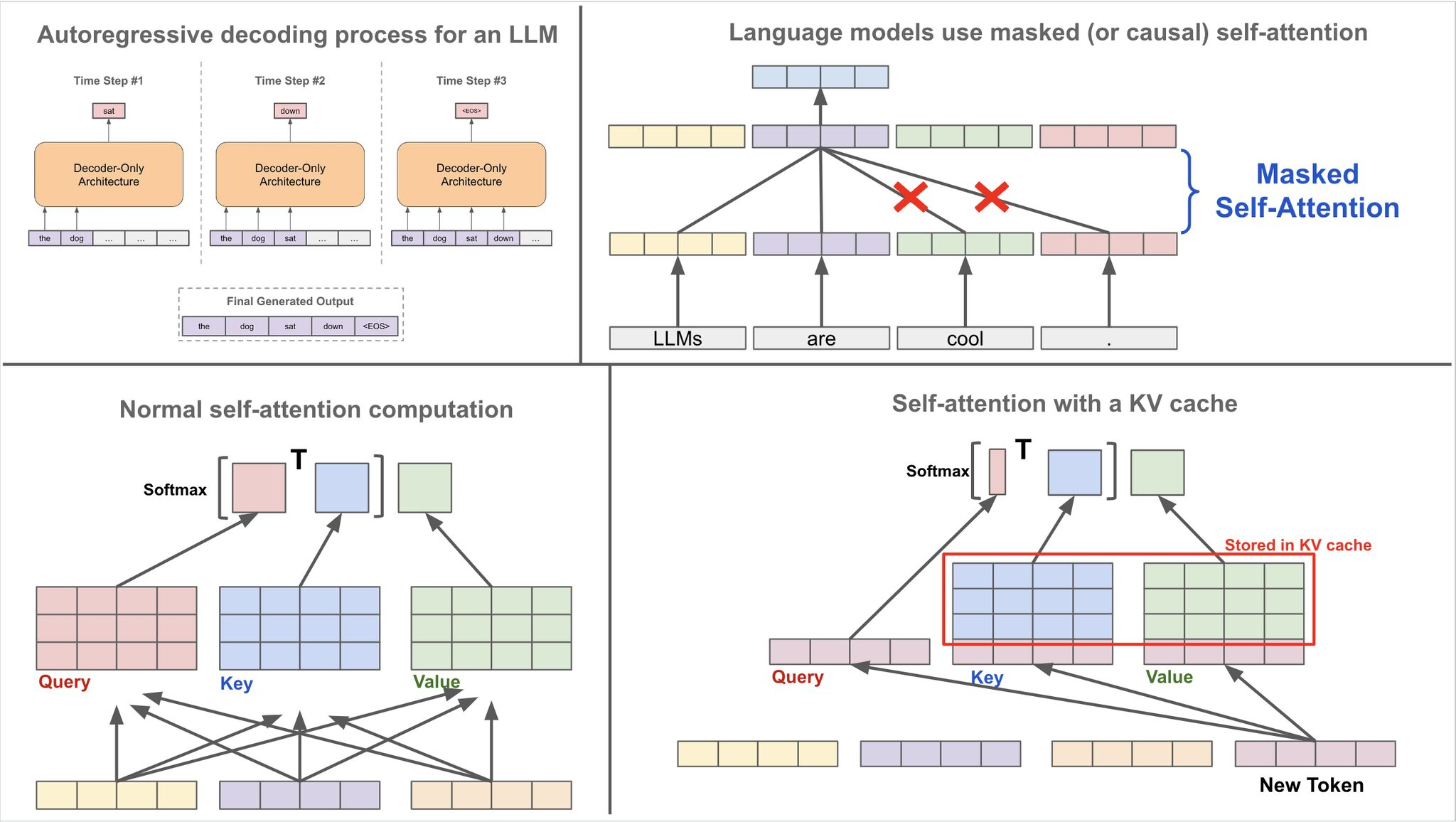##### Applications of Attention in Transformers
• From the paper, the Transformer uses multi-head attention in three different ways:
• The encoder contains self-attention layers. In a self-attention layer, all of the keys, values, and queries are derived from the same source, which is the word embedding for the first block of the encoder stack, while the output of the previous block for subsequent blocks. Each position in the encoder can attend to all positions in the previous block of the encoder.
• Similarly, self-attention layers in the decoder allow each position in the decoder to attend to all positions in the decoder up to and including that position. We need to prevent leftward information flow in the decoder to preserve the auto-regressive property. We implement this inside of scaled dot-product attention by masking out all values (by setting to a very low value, such as $$−\infty$$) in the input of the softmax which correspond to illegal connections.
• In “encoder-decoder attention” layers, the queries come from the previous decoder layer, and the memory keys and values come from the output of the encoder. This allows every position in the decoder to attend over all positions in the input sequence. This mimics the typical encoder-decoder attention mechanisms in sequence-to-sequence models such as Neural Machine Translation by Jointly Learning to Align and Translate, Google’s neural machine translation system: Bridging the gap between human and machine translation, and Convolutional Sequence to Sequence Learning.

• Let’s confront some of the simplistic assumptions we made during our first pass through explaining the attention mechanism. Words are represented as dense embedded vectors, rather than one-hot vectors. Attention isn’t just 1 or 0, on or off, but can also be anywhere in between. To get the results to fall between 0 and 1, we use the softmax trick again. It has the dual benefit of forcing all the values to lie in our [0, 1] attention range, and it helps to emphasize the highest value, while aggressively squashing the smallest. It’s the differential almost-argmax behavior we took advantage of before when interpreting the final output of the model.
• An complicating consequence of putting a softmax function in attention is that it will tend to focus on a single element. This is a limitation we didn’t have before. Sometimes it’s useful to keep several of the preceding words in mind when predicting the next, and the softmax just robbed us of that. This is a problem for the model.
• To address the above issues, the Transformer paper refined the self-attention layer by adding a mechanism called “multi-headed” attention. This improves the performance of the attention layer in two ways:
• It expands the model’s ability to focus on different positions. It would be useful if we’re translating a sentence like “The animal didn’t cross the street because it was too tired”, we would want to know which word “it” refers to.
• It gives the attention layer multiple “representation subspaces”. As we’ll see next, with multi-headed attention we have not only one, but multiple sets of $$Q, K, V$$ weight matrices (the Transformer uses eight attention heads, so we end up with eight sets for each encoder/decoder). Each of these sets is randomly initialized. Then, after training, each set is used to project the input embeddings (or vectors from lower encoders/decoders) into a different representation subspace.
• Further, getting the straightforward dot-product attention mechanism to work can be tricky. Bad random initializations of the learnable weights can de-stabilize the training process.
• Multiple heads lets the the transformer consider several previous words simultaneously when predicting the next. It brings back the power we had before we pulled the softmax into the picture.
• To fix the aforementioned issues, we can run multiple ‘heads’ of attention in parallel and concatenate the result (with each head now having separate learnable weights).
• To accomplish multi-head attention, self attention is simply conducted multiple times on different parts of the $$Q, K, V$$ matrices (each part corresponding to each attention head). Each $$q$$, $$k$$, and $$v$$ vector generated at the output contains concatenated output corresponding to contains each attention head. To obtain the output corresponding to each attention heads, we simply reshape the long $$q$$, $$k$$, and $$v$$ self-attention vectors into a matrix (with each row corresponding to the output of each attention head). From Jay Alammar’s: The Illustrated GPT-2: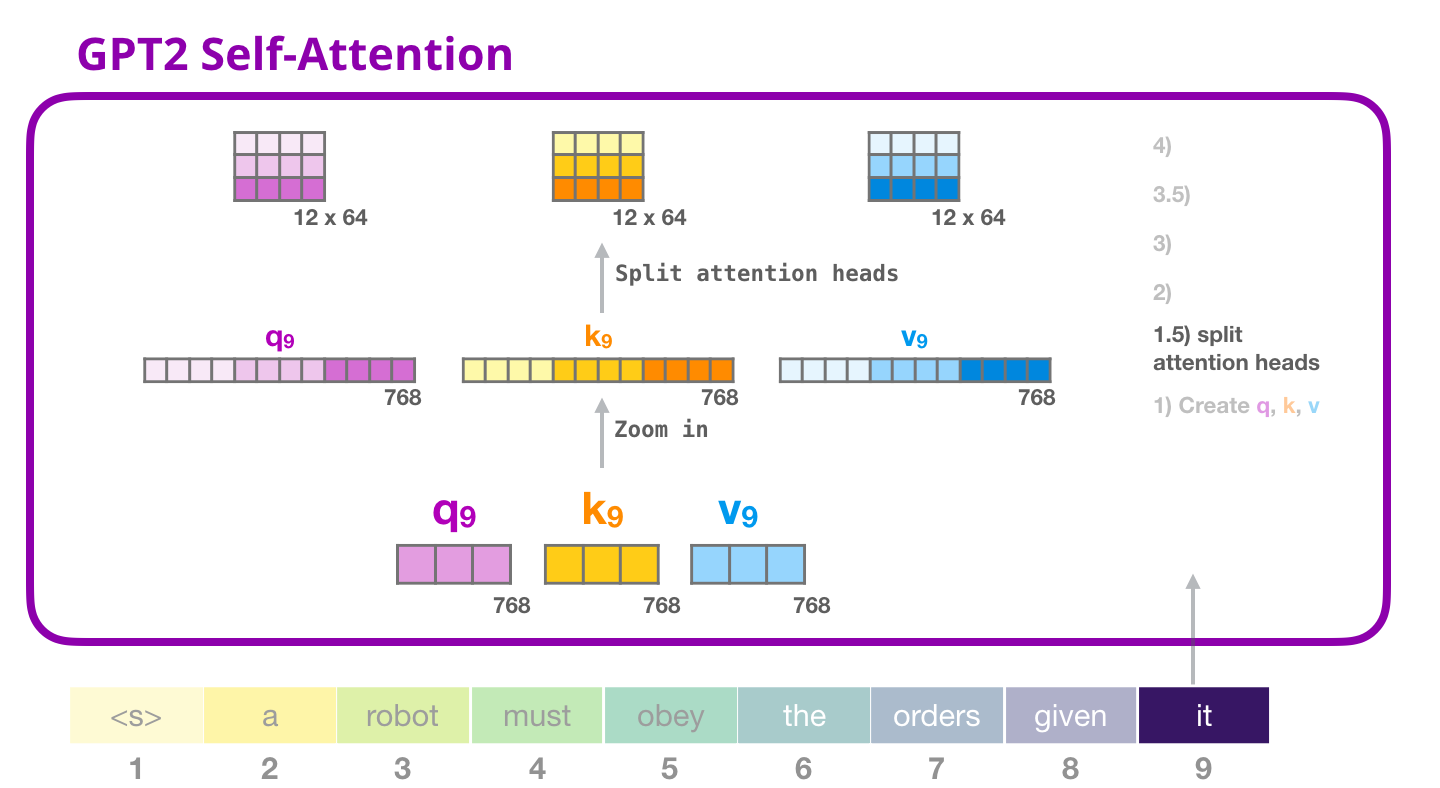• Mathematically,

$\begin{array}{c} h_{i}^{\ell+1}=\text {Concat }\left(\text {head }_{1}, \ldots, \text { head}_{K}\right) O^{\ell} \\ \text { head }_{k}=\text {Attention }\left(Q^{k, \ell} h_{i}^{\ell}, K^{k, \ell} h_{j}^{\ell}, V^{k, \ell} h_{j}^{\ell}\right) \end{array}$
• where $$Q^{k, \ell}, K^{k, \ell}, V^{k, \ell}$$ are the learnable weights of the $$k^{\prime}$$-th attention head and $$O^{\ell}$$ is a downprojection to match the dimensions of $$h_{i}^{\ell+1}$$ and $$h_{i}^{\ell}$$ across layers.
• Multiple heads allow the attention mechanism to essentially ‘hedge its bets’, looking at different transformations or aspects of the hidden features from the previous layer. More on this in the section on Why Multiple Heads of Attention? Why Attention?.

• Unfortunately, multi-head attention really increases the computational load. Computing attention was already the bulk of the work, and we just multiplied it by however many heads we want to use. To get around this, we can re-use the trick of projecting everything into a lower-dimensional embedding space. This shrinks the matrices involved which dramatically reduces the computation time.
• To see how this plays out, we can continue looking at matrix shapes. Tracing the matrix shape through the branches and weaves of the multi-head attention blocks requires three more numbers.
• $$d_k$$: dimensions in the embedding space used for keys and queries (64 in the paper).
• $$d_v$$: dimensions in the embedding space used for values (64 in the paper).
• $$h$$: the number of heads (8 in the paper).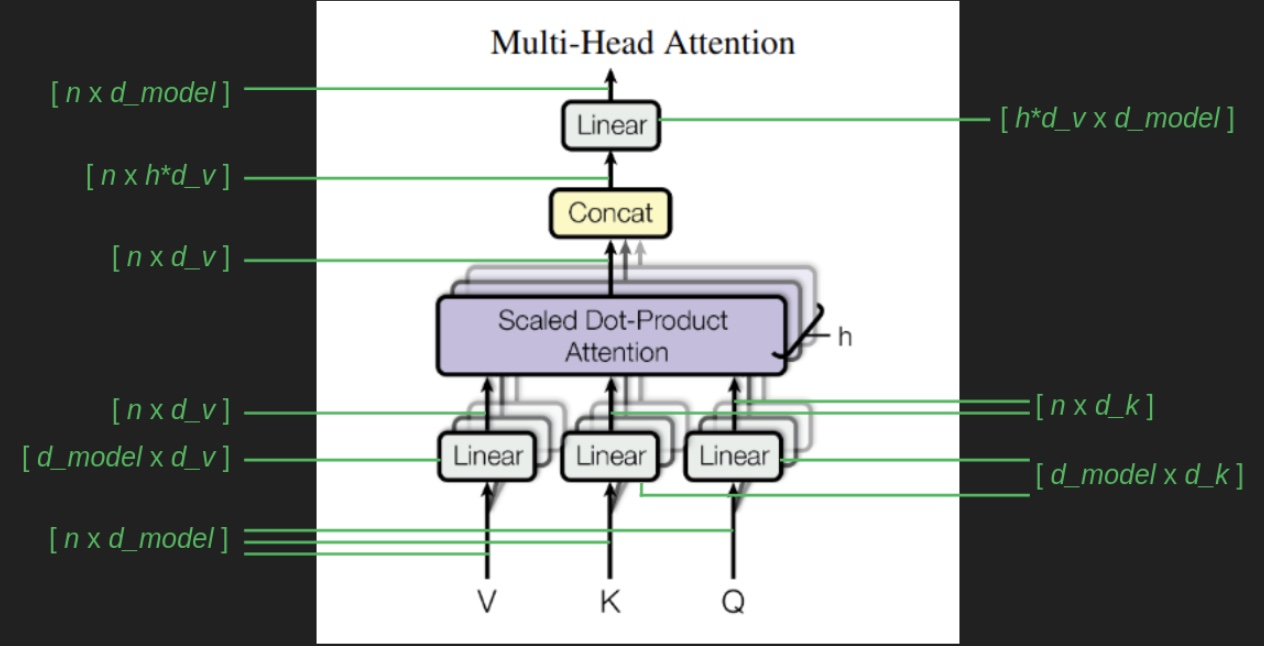• The $$[n \times d_{model}]$$ sequence of embedded words serves as the basis for everything that follows. In each case there is a matrix, $$W_v$$, $$W_q$$,, and $$W_k$$, (all shown unhelpfully as “Linear” blocks in the architecture diagram) that transforms the original sequence of embedded words into the values matrix, $$V$$, the queries matrix, $$Q$$, and the keys matrix, $$K$$. $$K$$ and $$Q$$ have the same shape, $$[n \times d_k]$$, but $$V$$ can be different, $$[n \times d_v]$$. It confuses things a little that $$d_k$$ and $$d_v$$ are the same in the paper, but they don’t have to be. An important aspect of this setup is that each attention head has its own $$W_v$$, $$W_q$$, and $$W_k$$ transforms. That means that each head can zoom in and expand the parts of the embedded space that it wants to focus on, and it can be different than what each of the other heads is focusing on.

• The result of each attention head has the same shape as $$V$$. Now we have the problem of h different result vectors, each attending to different elements of the sequence. To combine these into one, we exploit the powers of linear algebra, and just concatenate all these results into one giant $$[n \times h * d_v]$$ matrix. Then, to make sure it ends up in the same shape it started, we use one more transform with the shape $$[h * d_v \times d_{model}]$$.

• Here’s all of the that from the paper, stated tersely.

\begin{aligned} \operatorname{MultiHead}(Q, K, V) &=\operatorname{Concat}\left(\operatorname{head}_{1}, \ldots, \text { head }_{\mathrm{h}}\right) W^{O} \\ \text { where head } &=\operatorname{Attention}\left(Q W_{i}^{Q}, K W_{i}^{K}, V W_{i}^{V}\right) \end{aligned}
• where the projections are parameter matrices $$W_{i}^{Q} \in \mathbb{R}^{d_{\text {model }} \times d_{k}}, W_{i}^{K} \in \mathbb{R}^{d_{\text {model }} \times d_{k}}, W_{i}^{V} \in \mathbb{R}^{d_{\text {model }} \times d_{v}}$$ and $$W^{O} \in \mathbb{R}^{h d_{v} \times d_{\text {model }}}$$.
##### Why have multiple attention heads?
• Per Eugene Yan’s Some Intuition on Attention and the Transformer blog, multiple heads lets the model consider multiple words simultaneously. Because we use the softmax function in attention, it amplifies the highest value while squashing the lower ones. As a result, each head tends to focus on a single element.
• Consider the sentence: “The chicken crossed the road carelessly”. The following words are relevant to “crossed” and should be attended to:
• The “chicken” is the subject doing the crossing.
• The “road” is the object being crossed.
• The crossing is done “carelessly”.
• If we had a single attention head, we might only focus on a single word, either “chicken”, “road”, or “crossed”. Multiple heads let us attend to several words. It also provides redundancy, where if any single head fails, we have the other attention heads to rely on.

#### Cross-Attention

• The final step in getting the full transformer up and running is the connection between the encoder and decoder stacks, the cross attention block. We’ve saved it for last and, thanks to the groundwork we’ve laid, there’s not a lot left to explain.

• Cross-attention works just like self-attention with the exception that the key matrix $$K$$ and value matrix $$V$$ are based on the output of the encoder stack (i.e., the final encoder layer), rather than the output of the previous decoder layer. The query matrix $$Q$$ is still calculated from the results of the previous decoder layer. This is the channel by which information from the source sequence makes its way into the target sequence and steers its creation in the right direction. It’s interesting to note that the same embedded source sequence (output from the final layer in the encoder stack) is provided to every layer of the decoder, supporting the notion that successive layers provide redundancy and are all cooperating to perform the same task. The following diagram highlights the cross-attention piece within the transformer architecture.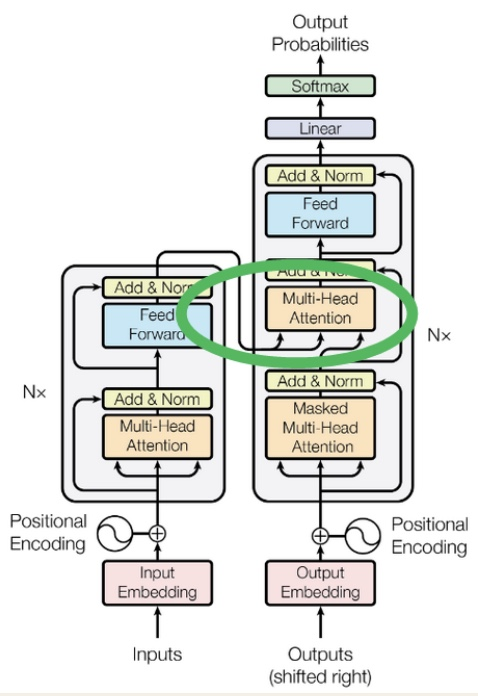#### Skip connections

• Attention is the most fundamental part of what transformers do. It’s the core mechanism, and we have now traversed it had a pretty concrete level. Everything from here on out is the plumbing necessary to make it work well. It’s the rest of the harness that lets attention pull our heavy workloads.

• One piece we haven’t explained yet are skip connections. These occur around the Multi-Head Attention blocks, and around the element wise Feed Forward blocks in the blocks labeled “Add and Norm”. In skip connections, a copy of the input is added to the output of a set of calculations. The inputs to the attention block are added back in to its output. The inputs to the element-wise feed forward block are added to its outputs. The following diagram shows the Transformer architecture showing add and norm blocks.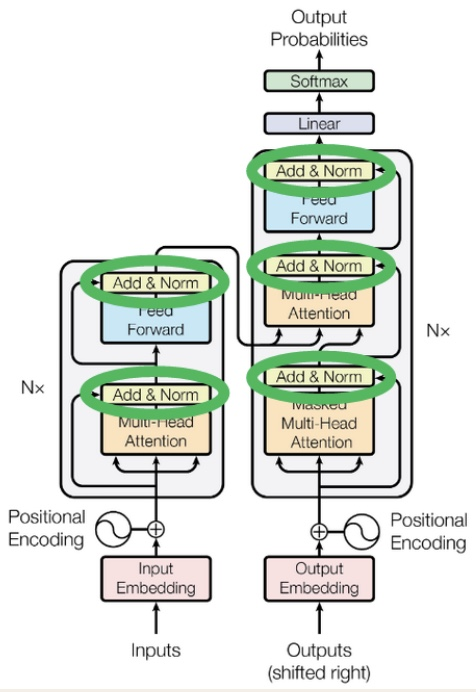• Skip connections serve two purposes:
1. They help keep the gradient smooth, which is a big help for backpropagation. Attention is a filter, which means that when it’s working correctly it will block most of what tries to pass through it. The result of this is that small changes in a lot of the inputs may not produce much change in the outputs if they happen to fall into channels that are blocked. This produces dead spots in the gradient where it is flat, but still nowhere near the bottom of a valley. These saddle points and ridges are a big tripping point for backpropagation. Skip connections help to smooth these out. In the case of attention, even if all of the weights were zero and all the inputs were blocked, a skip connection would add a copy of the inputs to the results and ensure that small changes in any of the inputs will still have noticeable changes in the result. This keeps gradient descent from getting stuck far away from a good solution. Skip connections have become popular because of how they improve performance since the days of the ResNet image classifier. They are now a standard feature in neural network architectures. The figure below (source) shows the effect that skip connections have by comparing a ResNet with and without skip connections. The slopes of the loss function hills are are much more moderate and uniform when skip connections are used. If you feel like taking a deeper dive into how the work and why, there’s a more in-depth treatment in this post. The following diagram shows the comparison of loss surfaces with and without skip connections.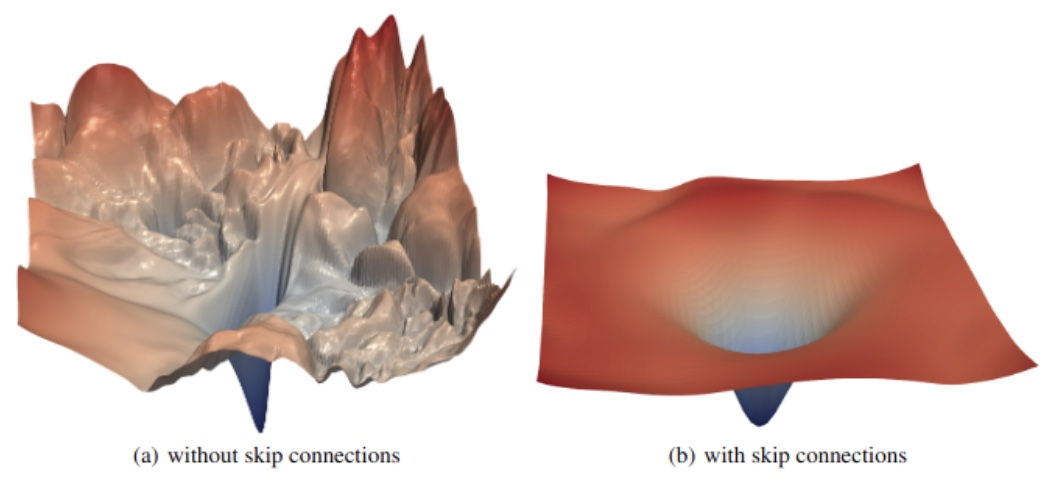2. The second purpose of skip connections is specific to transformers —- preserving the original input sequence. Even with a lot of attention heads, there’s no guarantee that a word will attend to its own position. It’s possible for the attention filter to forget entirely about the most recent word in favor of watching all of the earlier words that might be relevant. A skip connection takes the original word and manually adds it back into the signal, so that there’s no way it can be dropped or forgotten. This source of robustness may be one of the reasons for transformers’ good behavior in so many varied sequence completion tasks.
##### Why have skip connections?
• Per Eugene Yan’s Some Intuition on Attention and the Transformer blog, because attention acts as a filter, it blocks most information from passing through. As a result, a small change to the inputs of the attention layer may not change the outputs, if the attention score is tiny or zero. This can lead to flat gradients or local optima.
• Skip connections help dampen the impact of poor attention filtering. Even if an input’s attention weight is zero and the input is blocked, skip connections add a copy of that input to the output. This ensures that even small changes to the input can still have noticeable impact on the output. Furthermore, skip connections preserve the input sentence: There’s no guarantee that a context word will attend to itself in a transformer. Skip connections ensure this by taking the context word vector and adding it to the output.

#### Layer normalization

• Normalization is a step that pairs well with skip connections. There’s no reason they necessarily have to go together, but they both do their best work when placed after a group of calculations, like attention or a feed forward neural network.
• The short version of layer normalization is that the values of the matrix are shifted to have a mean of zero and scaled to have a standard deviation of one. The following diagram shows several distributions being normalized.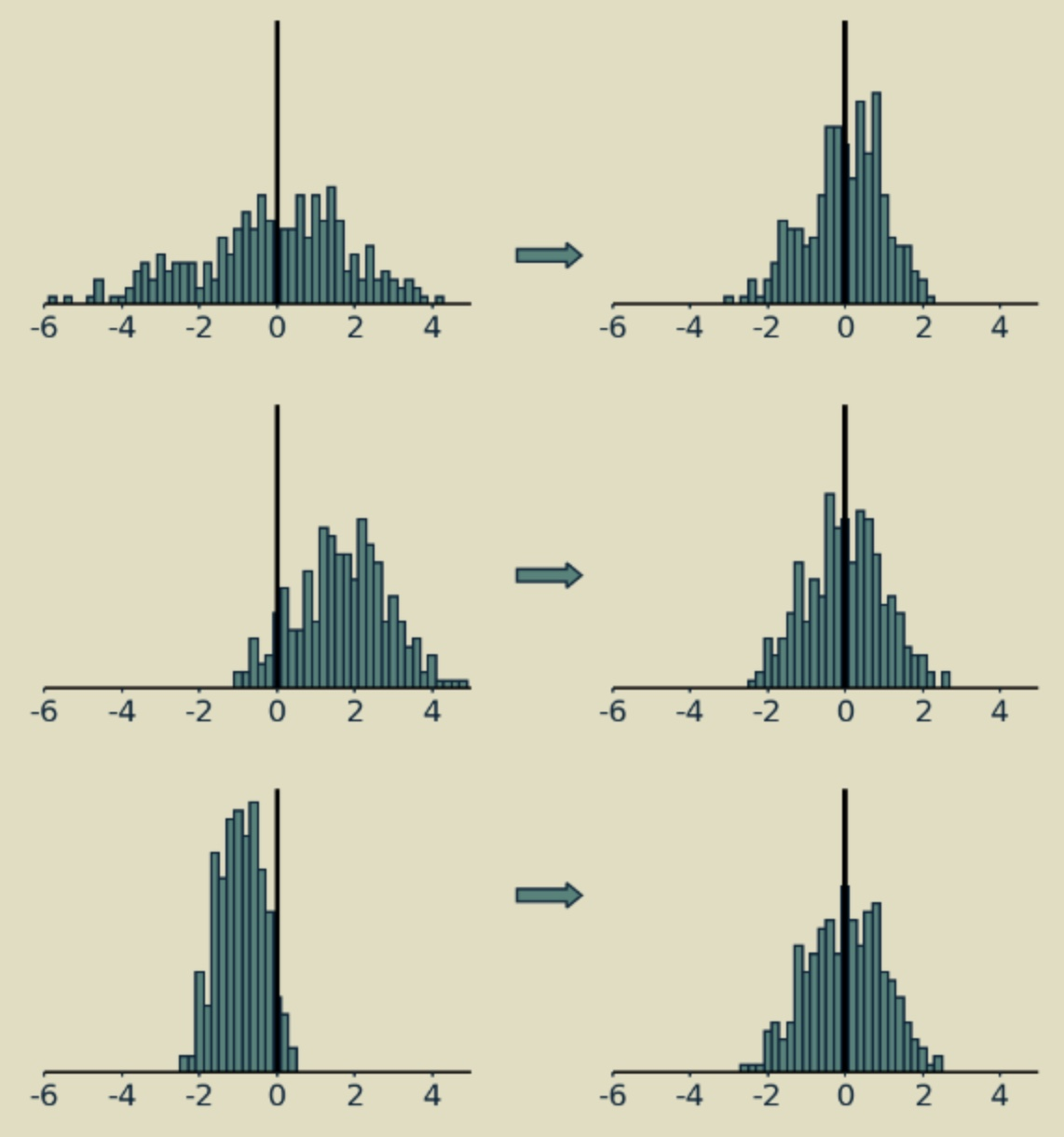• The longer version is that in systems like transformers, where there are a lot of moving pieces and some of them are something other than matrix multiplications (such as softmax operators or rectified linear units), it matters how big values are and how they’re balanced between positive and negative. If everything is linear, you can double all your inputs, and your outputs will be twice as big, and everything will work just fine. Not so with neural networks. They are inherently nonlinear, which makes them very expressive but also sensitive to signals’ magnitudes and distributions. Normalization is a technique that has proven useful in maintaining a consistent distribution of signal values each step of the way throughout many-layered neural networks. It encourages convergence of parameter values and usually results in much better performance.
• To understand the different types of normalization techniques, please refer Normalization Methods which includes batch normalization, a close cousin of the layer normalization used in transformers.

#### Softmax

• The argmax function is “hard” in the sense that the highest value wins, even if it is only infinitesimally larger than the others. If we want to entertain several possibilities at once, it’s better to have a “soft” maximum function, which we get from softmax. To get the softmax of the value $$x$$ in a vector, divide the exponential of $$x$$, $$e^x$$, by the sum of the exponentials of all the values in the vector. This converts the (unnormalized) logits/energy values into (normalized) probabilities $$\in [0, 1]$$, with all summing up to 1.

• The softmax is helpful here for three reasons. First, it converts our de-embedding results vector from an arbitrary set of values to a probability distribution. As probabilities, it becomes easier to compare the likelihood of different words being selected and even to compare the likelihood of multi-word sequences if we want to look further into the future.

• Second, it thins the field near the top. If one word scores clearly higher than the others, softmax will exaggerate that difference (owing to the “exponential” operation), making it look almost like an argmax, with the winning value close to one and all the others close to zero. However, if there are several words that all come out close to the top, it will preserve them all as highly probable, rather than artificially crushing close second place results, which argmax is susceptible to. You might be thinking what the difference between standard normalization and softmax is – after all, both rescale the logits between 0 and 1. By using softmax, we are effectively “approximating” argmax as indicated earlier while gaining differentiability. Rescaling doesn’t weigh the max significantly higher than other logits, whereas softmax does due to its “exponential” operation. Simply put, softmax is a “softer” argmax.

• Third, softmax is differentiable, meaning we can calculate how much each element of the results will change, given a small change in any of the input elements. This allows us to use it with backpropagation to train our transformer.

• Together the de-embedding transform (shown as the Linear block below) and a softmax function complete the de-embedding process. The following diagram shows the de-embedding steps in the architecture diagram (source: Transformers paper).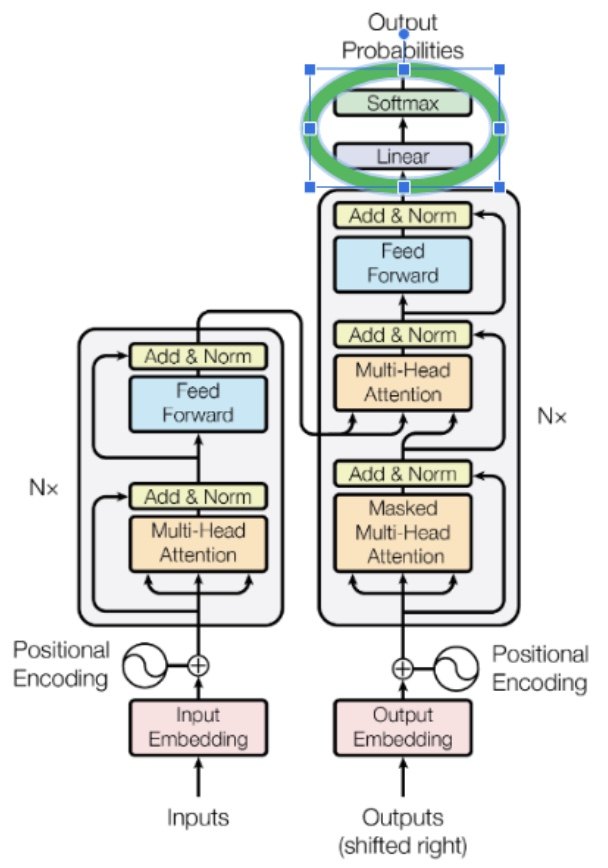#### Stacking Transformer Layers

• While we were laying the foundations above, we showed that an attention block and a feed forward block with carefully chosen weights were enough to make a decent language model. Most of the weights were zeros in our examples, a few of them were ones, and they were all hand picked. When training from raw data, we won’t have this luxury. At the beginning the weights are all chosen randomly, most of them are close to zero, and the few that aren’t probably aren’t the ones we need. It’s a long way from where it needs to be for our model to perform well.
• Stochastic gradient descent through backpropagation can do some pretty amazing things, but it relies a lot on trial-and-error. If there is just one way to get to the right answer, just one combination of weights necessary for the network to work well, then it’s unlikely that it will find its way. But if there are lots of paths to a good solution, chances are much better that the model will get there.
• Having a single attention layer (just one multi-head attention block and one feed forward block) only allows for one path to a good set of transformer parameters. Every element of every matrix needs to find its way to the right value to make things work well. It is fragile and brittle, likely to get stuck in a far-from-ideal solution unless the initial guesses for the parameters are very very lucky.
• The way transformers sidestep this problem is by having multiple attention layers, each using the output of the previous one as its input. The use of skip connections make the overall pipeline robust to individual attention blocks failing or giving wonky results. Having multiples means that there are others waiting to take up the slack. If one should go off the rails, or in any way fail to live up to its potential, there will be another downstream that has another chance to close the gap or fix the error. The paper showed that more layers resulted in better performance, although the improvement became marginal after 6.
• Another way to think about multiple layers is as a conveyor belt assembly line. Each attention block and feedforward block has the chance to pull inputs off the line, calculate useful attention matrices and make next word predictions. Whatever results they produce, useful or not, get added back onto the conveyer, and passed to the next layer. The following diagram shows the transformer redrawn as a conveyor belt: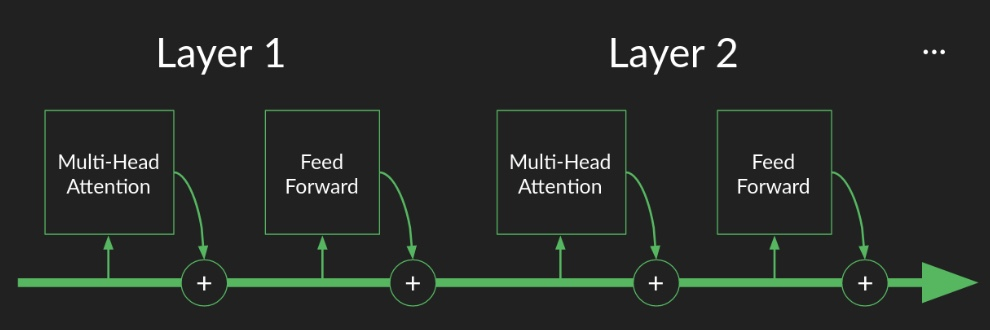• This is in contrast to the traditional description of many-layered neural networks as “deep”. Thanks to skip connections, successive layers don’t provide increasingly sophisticated abstraction as much as they provide redundancy. Whatever opportunities for focusing attention and creating useful features and making accurate predictions were missed in one layer can always be caught by the next. Layers become workers on the assembly line, where each does what it can, but doesn’t worry about catching every piece, because the next worker will catch the ones they miss.
##### Why have multiple attention layers?
• Per Eugene Yan’s Some Intuition on Attention and the Transformer blog, multiple attention layers builds in redundancy (on top of having multiple attention heads). If we only had a single attention layer, that attention layer would have to do a flawless job—this design could be brittle and lead to suboptimal outcomes. We can address this via multiple attention layers, where each one uses the output of the previous layer with the safety net of skip connections. Thus, if any single attention layer messed up, the skip connections and downstream layers can mitigate the issue.
• Stacking attention layers also broadens the model’s receptive field. The first attention layer produces context vectors by attending to interactions between pairs of words in the input sentence. Then, the second layer produces context vectors based on pairs of pairs, and so on. With more attention layers, the Transformer gains a wider perspective and can attend to multiple interaction levels within the input sentence.

#### Transformer Encoder and Decoder

• The Transformer model has two parts: encoder and decoder. Both encoder and decoder are mostly identical (with a few differences) and are comprised of a stack of transformer blocks. Each block is comprised of a combination of multi-head attention blocks, positional feedforward layers, residual connections and layer normalization blocks.
• The attention layers from the encoder and decoder have the following differences:
• The encoder only has self-attention blocks while the decoder has a cross-attention encoder-decoder layer sandwiched between the self-attention layer and the feedforward neural network.
• Also, the self-attention blocks are masked to ensure causal predictions (i.e., the prediction of token $$N$$ only depends on the previous $$N - 1$$ tokens, and not on the future ones).
• Each of the encoding/decoding blocks contains many stacked encoders/decoder transformer blocks. The Transformer encoder is a stack of six encoders, while the decoder is a stack of six decoders. The initial layers capture more basic patterns (broadly speaking, basic syntactic patterns), whereas the last layers can detect more sophisticated ones, similar to how convolutional networks learn to look for low-level features such as edges and blobs of color in the initial layers while the mid layers focus on learning high-level features such as object shapes and textures the later layers focus on detecting the entire objects themselves (using textures, shapes and patterns learnt from earlier layers as building blocks).
• The six encoders and decoders are identical in structure but do not share weights. Check weights shared by different parts of a transformer model for a detailed discourse on weight sharing opportunities within the Transformer layers.
• For more on the pros and cons of the encoder and decoder stack, refer Autoregressive vs. Autoencoder Models.
##### Decoder stack

The decoder, which follows the auto-regressive property, i.e., consumes the tokens generated so far to generate the next one, is used standalone for generation tasks, such as tasks in the domain of natural language generation (NLG), for e.g., such as summarization, translation, or abstractive question answering. Decoder models are typically trained with an objective of predicting the next token, i.e., “autoregressive blank infilling”.

• As we laid out in the section on Sampling a Sequence of Output Words, the decoder can complete partial sequences and extend them as far as you want. OpenAI created the generative pre-training (GPT) family of models to do just this, by training on a predicting-the-next-token objective. The architecture they describe in this report should look familiar. It is a transformer with the encoder stack and all its connections surgically removed. What remains is a 12 layer decoder stack. The following diagram from the GPT-1 paper Improving Language Understanding by Generative Pre-Training shows the architecture of the GPT family of models: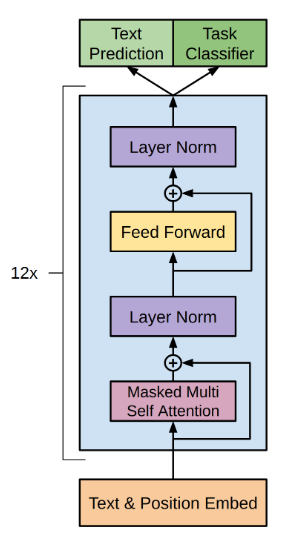• Any time you come across a generative/auto-regressive model, such as GPT-X, LLaMA, Copilot, etc., you’re probably seeing the decoder half of a transformer in action.
##### Encoder stack

The encoder, is typically used standalone for content understanding tasks, such as tasks in the domain of natural language understanding (NLU) that involve classification, for e.g., sentiment analysis, or extractive question answering. Encoder models are typically trained with a “fill in the blanks”/”blank infilling” objective – reconstructing the original data from masked/corrupted input (i.e., by randomly sampling tokens from the input and replacing them with [MASK] elements, or shuffling sentences in random order if it’s the next sentence prediction task). In that sense, an encoder can be thought of as an auto-encoder which seeks to denoise a partially corrupted input, i.e., “Denoising Autoencoder” (DAE) and aim to recover the original undistorted input.

• Almost everything we’ve learned about the decoder applies to the encoder too. The biggest difference is that there’s no explicit predictions being made at the end that we can use to judge the rightness or wrongness of its performance. Instead, the end product of an encoder stack is an abstract representation in the form of a sequence of vectors in an embedded space. It has been described as a pure semantic representation of the sequence, divorced from any particular language or vocabulary, but this feels overly romantic to me. What we know for sure is that it is a useful signal for communicating intent and meaning to the decoder stack.
• Having an encoder stack opens up the full potential of transformers instead of just generating sequences, they can now translate (or transform) the sequence from one language to another. Training on a translation task is different than training on a sequence completion task. The training data requires both a sequence in the language of origin, and a matching sequence in the target language. The full language of origin is run through the encoder (no masking this time, since we assume that we get to see the whole sentence before creating a translation) and the result, the output of the final encoder layer is provided as an input to each of the decoder layers. Then sequence generation in the decoder proceeds as before, but this time with no prompt to kick it off.

• Any time you come across an encoder model that generates semantic embeddings, such as BERT, ELMo, etc., you’re likely seeing the encoder half of a transformer in action.

#### Putting it all together: The Transformer Architecture

• The Transformer architecture combines the individual encoder/decoder models. The encoder takes the input and encodes it into fixed-length query, key, and vector tensors (analogous to the fixed-length context vector in the original paper by Bahdanau et al. (2015)) that introduced attention. These tensors are passed onto the decoder which decodes it into the output sequence.
• The encoder (left) and decoder (right) of the transformer is shown below: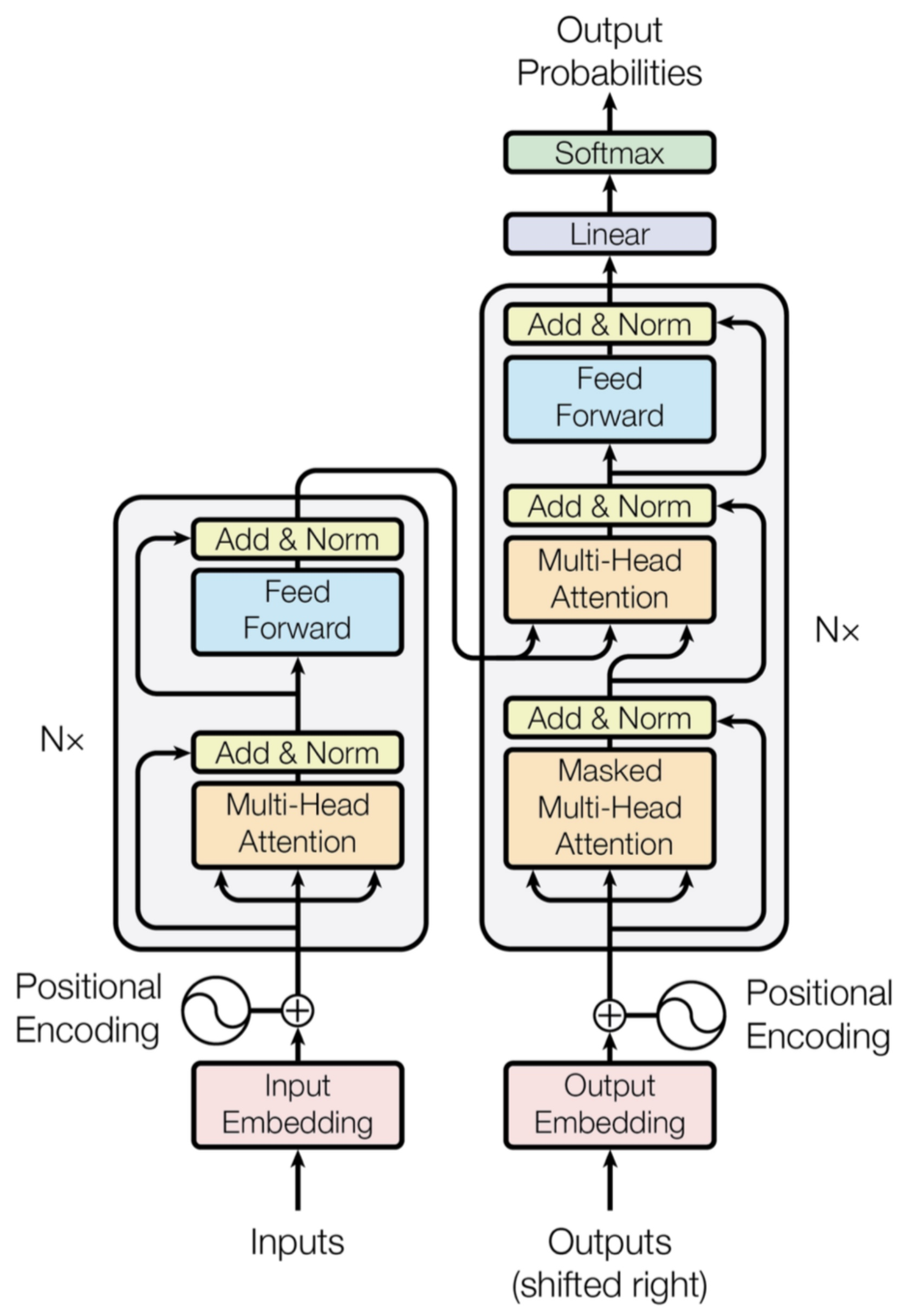• Note that the multi-head attention in the encoder is the scaled dot-product multi-head self attention, while that in the initial layer in the decoder is the masked scaled dot-product multi-head self attention and the middle layer (which enables the decoder to attend to the encoder) is the scaled dot-product multi-head cross attention.

• Re-drawn vectorized versions from DAIR.AI are as follows: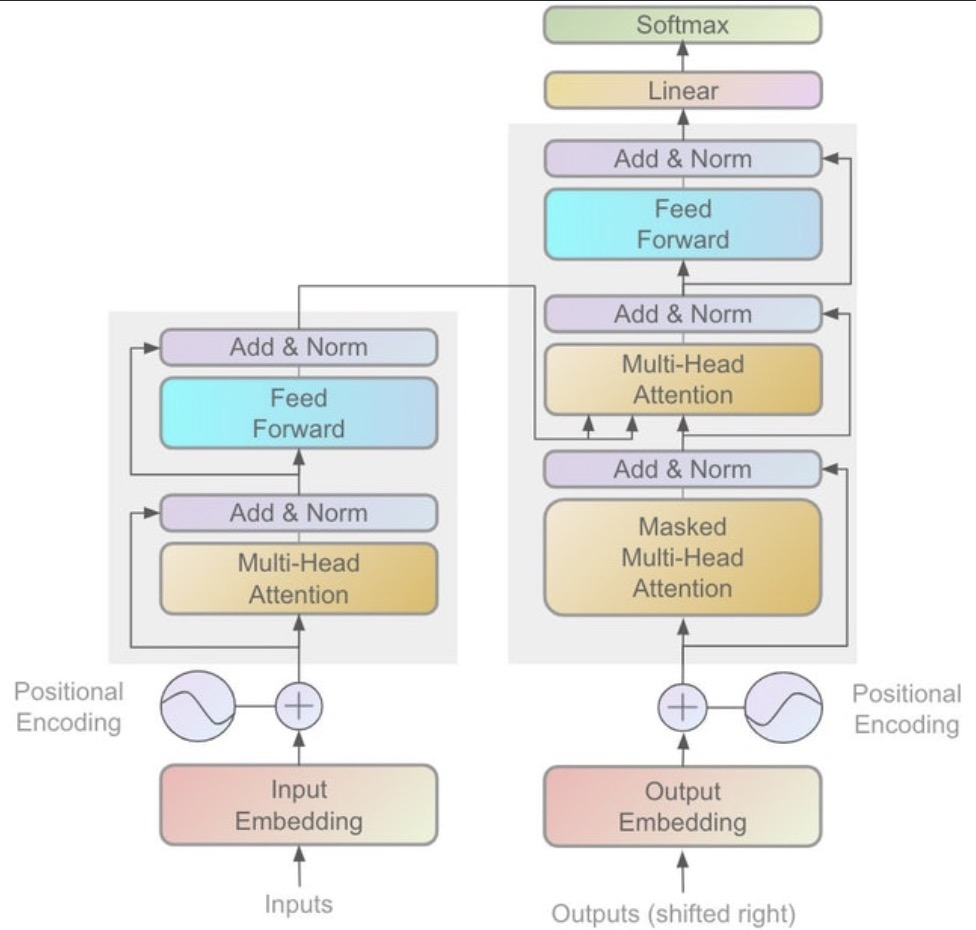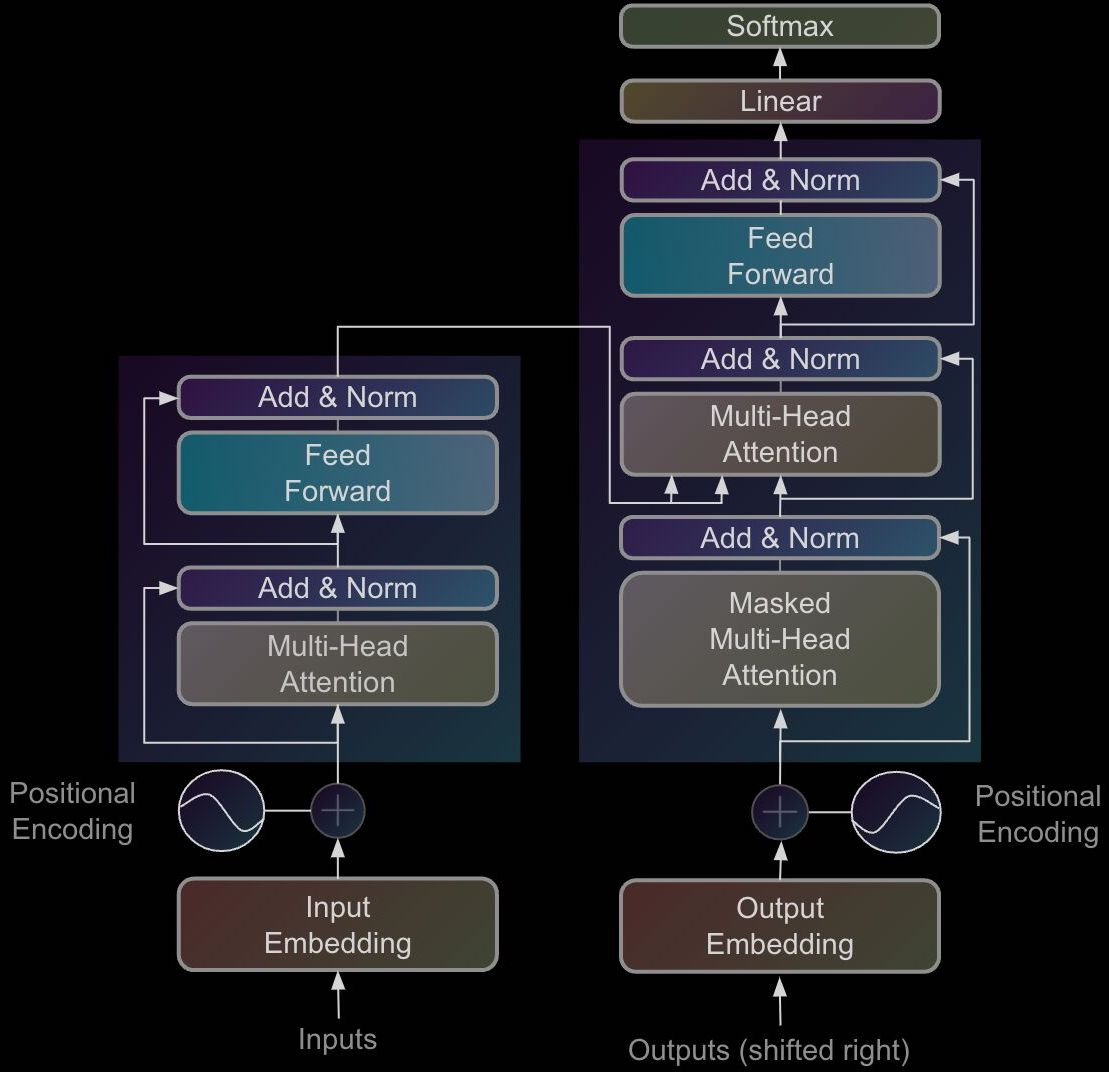• The full model architecture of the transformer – from fig. 1 and 2 in Vaswani et al. (2017) – is as follows: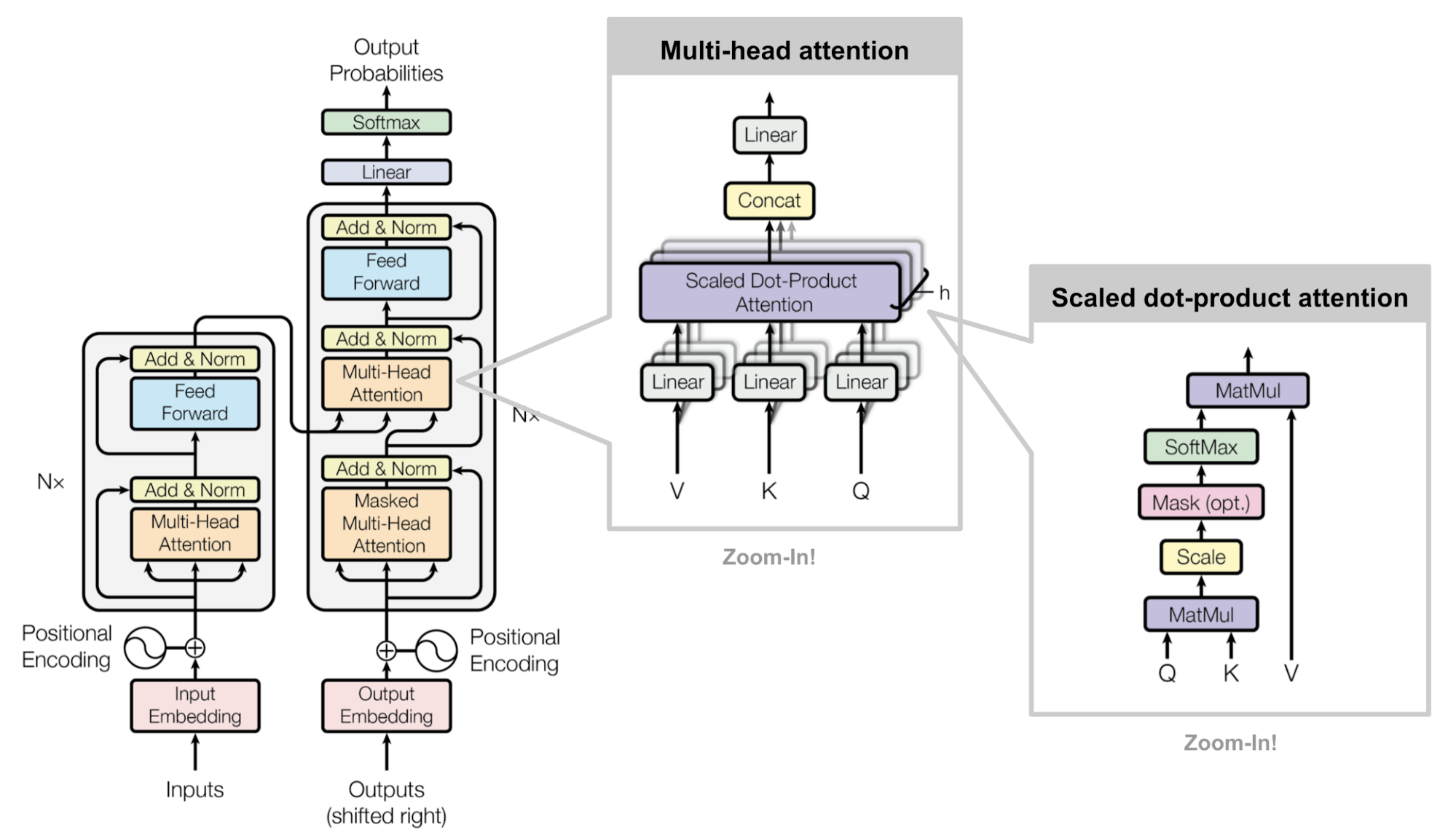• Here is an illustrated version of the overall Transformer architecture from Abdullah Al Imran: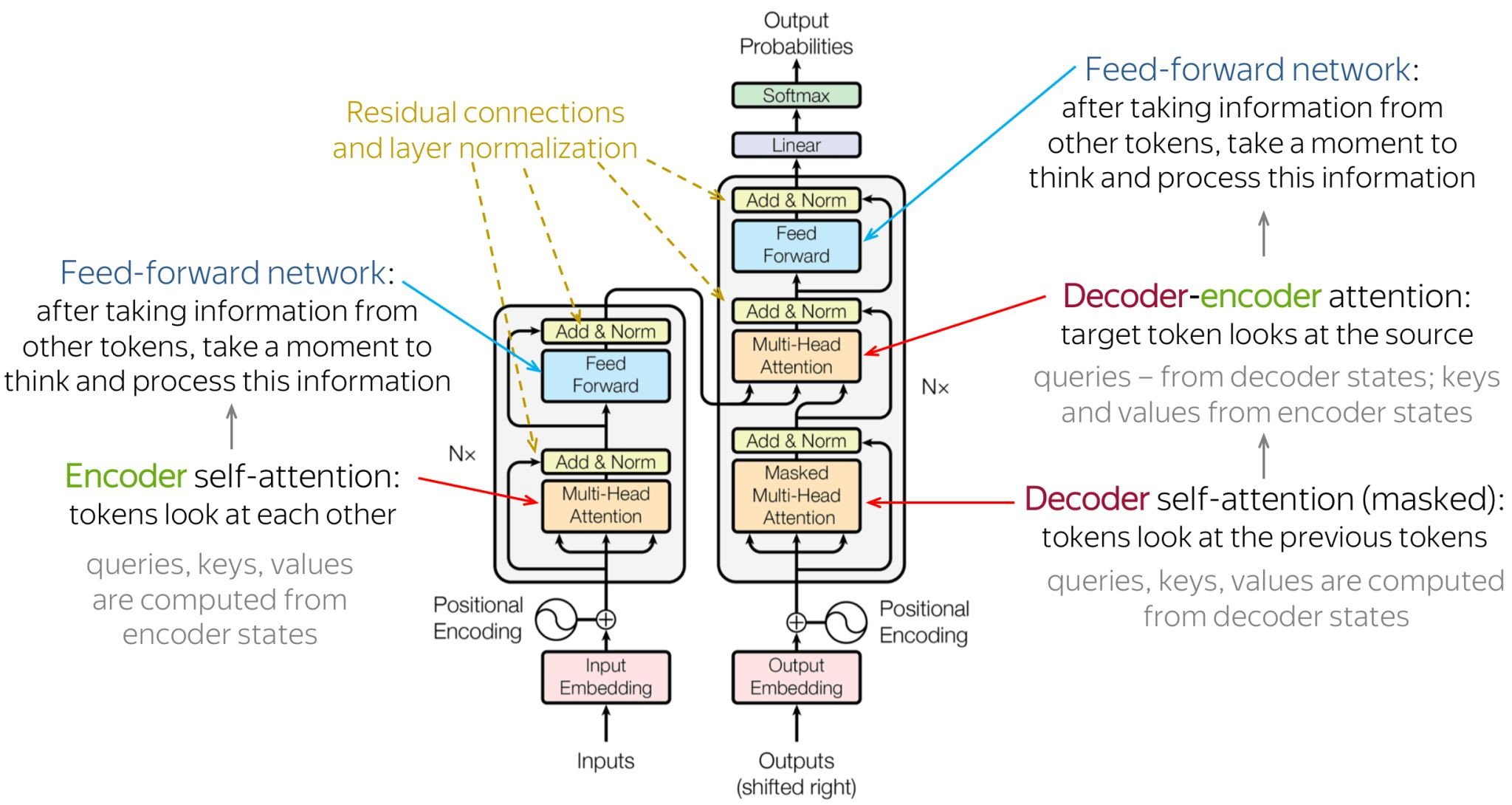• As a walk-through exercise, the following diagram (source: CS330 slides) shows an sample input sentence “Joe Biden is the US President” being fed in as input to the Transformer. The various transformations that occur as the input vector is processed are:
1. Input sequence: $$I$$ = “Joe Biden is the US President”.
2. Tokenization: $$I \in {\mid \text { vocab } \mid}^{T}$$.
3. Input embeddings lookup: $$E \in \mathbb{R}^{T \times d}$$.
4. Inputs to Transformer block: $$X \in \mathbb{R}^{T \times d}$$.
5. Obtaining three separate linear projections of input $$X$$ (queries, keys, and values): $$X_Q=X W_Q, \quad X_K=X W_K, \quad X_V=X W_V$$.
6. Calculating self-attention: $$A=\operatorname{sm}\left(X_Q X_K^{\top}\right) X_V$$ (the scaling part is missing in the figure below – you can reference the section on Types of Attention: Additive, Multiplicative (Dot-product), and Scaled for more).
• This is followed by a residual connection and LayerNorm.
7. Feed-forward (MLP) layers which perform two linear transformations/projections of the input with a ReLU activation in between: $$\operatorname{FFN}(x)=\max \left(0, x W_1+b_1\right) W_2+b_2$$
• This is followed by a residual connection and LayerNorm.
8. Output of the Transformer block: $$O \in \mathbb{R}^{T \times d}$$.
9. Project to vocabulary size at time $$t$$: $$p_\theta^t(\cdot) \in \mathbb{R}^{\mid \text {vocab } \mid}$$.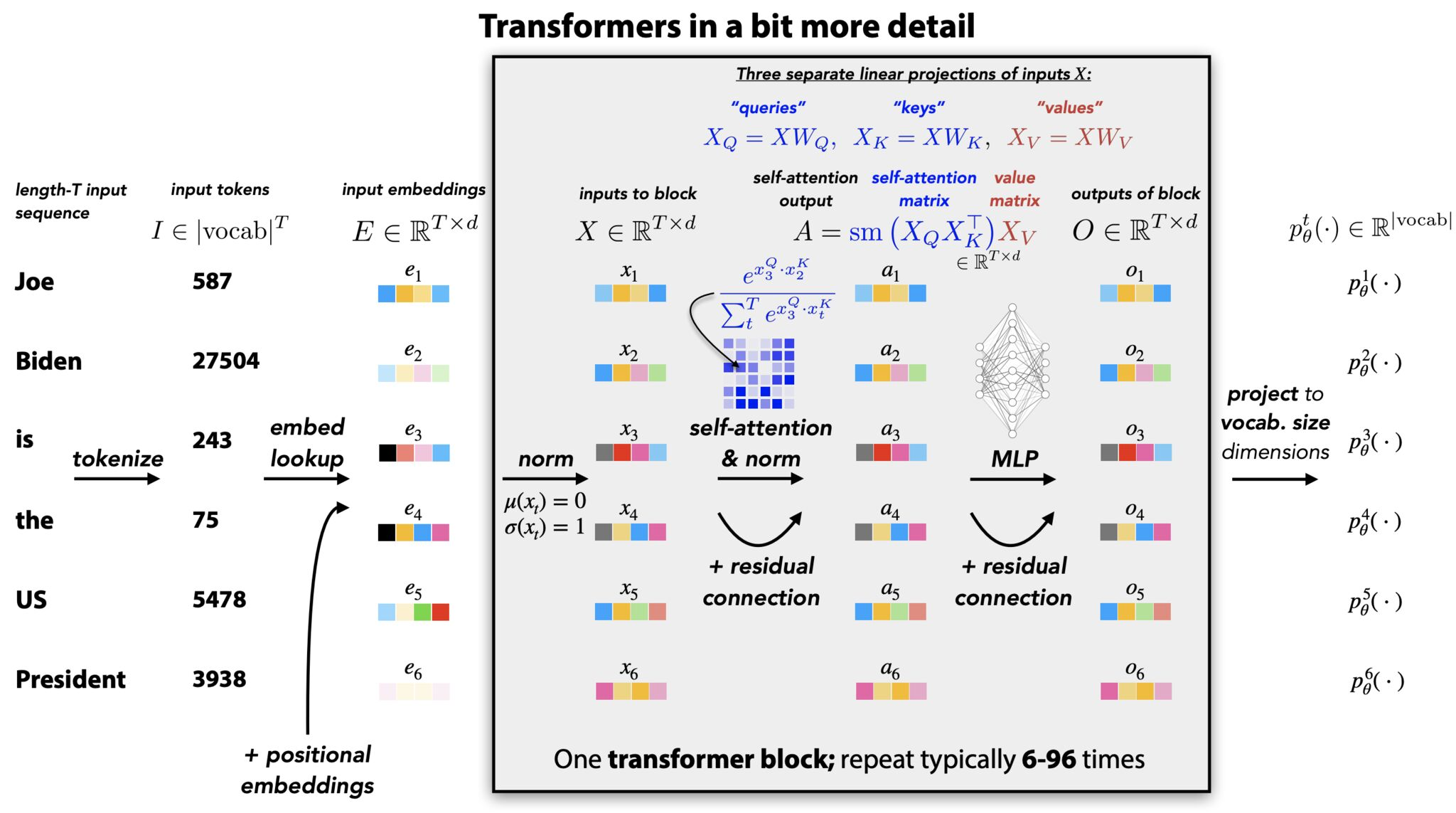#### Loss function

• The encoder and decoder are jointly trained (“end-to-end”) to minimize the cross-entropy loss between the predicted probability matrix of shape output sequence length $\times$ vocab (right before taking the argmax on the output of the softmax to ascertain the next token to output), and the output sequence length-sized output vector of token IDs as the true label.
• Effectively, the cross-entropy loss “pulls” the predicted probability of the correct class towards 1 during training. This is accomplished by calculating gradients of the loss function w.r.t. the model’s weights; with the model’s sigmoid/softmax output (in case of binary/multiclass classification) serving as the prediction (i.e., the pre-argmax output is utilized since argmax is not differentiable).

## Implementation details

### Tokenizing

• We made it all the way through the transformer! We covered it in enough detail that there should be no mysterious black boxes left. There are a few implementation details that we didn’t dig into. You would need to know about them in order to build a working version for yourself. These last few tidbits aren’t so much about how transformers work as they are about getting neural networks to behave well. The Annotated Transformer will help you fill in these gaps.

• In the section on One-hot encoding, we discussed that a vocabulary could be represented by a high dimensional one-hot vector, with one element associated with each word. In order to do this, we need to know exactly how many words we are going to be representing and what they are.

• A naïve approach is to make a list of all possible words, like we might find in Webster’s Dictionary. For the English language this will give us several tens of thousands, the exact number depending on what we choose to include or exclude. But this is an oversimplification. Most words have several forms, including plurals, possessives, and conjugations. Words can have alternative spellings. And unless your data has been very carefully cleaned, it will contain typographical errors of all sorts. This doesn’t even touch on the possibilities opened up by freeform text, neologisms, slang, jargon, and the vast universe of Unicode. An exhaustive list of all possible words would be infeasibly long.

• A reasonable fallback position would be to have individual characters serve as the building blocks, rather than words. An exhaustive list of characters is well within the capacity we have to compute. However there are a couple of problems with this. After we transform data into an embedding space, we assume the distance in that space has a semantic interpretation, that is, we assume that points that fall close together have similar meanings, and points that are far away mean something very different. That allows us to implicitly extend what we learn about one word to its immediate neighbors, an assumption we rely on for computational efficiency and from which the transformer draws some ability to generalize.

• At the individual character level, there is very little semantic content. There are a few one character words in the English language for example, but not many. Emoji are the exception to this, but they are not the primary content of most of the data sets we are looking at. That leaves us in the unfortunate position of having an unhelpful embedding space.

• It might still be possible to work around this theoretically, if we could look at rich enough combinations of characters to build up semantically useful sequences like words, words stems, or word pairs. Unfortunately, the features that transformers create internally behave more like a collection of input pairs than an ordered set of inputs. That means that the representation of a word would be a collection of character pairs, without their order strongly represented. The transformer would be forced to continually work with anagrams, making its job much harder. And in fact experiments with character level representations have shown the transformers don’t perform very well with them.

### Byte pair encoding (BPE)

• Fortunately, there is an elegant solution to this called byte pair encoding, which is a simple form of data compression in which the most common pair of consecutive bytes of data is replaced with a byte that does not occur within that data. A table of the replacements is required to rebuild the original data.
• Starting with the character level representation, each character is assigned a code, its own unique byte. Then after scanning some representative data, the most common pair of bytes is grouped together and assigned a new byte, a new code. This new code is substituted back into the data, and the process is repeated.

#### Example

aaabdaaabac

• The byte pair “aa” occurs most often, so it will be replaced by a byte that is not used in the data, “Z”. Now there is the following data and replacement table:
ZabdZabac
Z=aa

• Then the process is repeated with byte pair “ab”, replacing it with Y:
ZYdZYac
Y=ab
Z=aa

• The only literal byte pair left occurs only once, and the encoding might stop here. Or the process could continue with recursive byte pair encoding, replacing “ZY” with “X”:
XdXac
X=ZY
Y=ab
Z=aa

• This data cannot be compressed further by byte pair encoding because there are no pairs of bytes that occur more than once.
• To decompress the data, simply perform the replacements in the reverse order.

#### Applying BPE to learn new, rare, and misspelled words

• Codes representing pairs of characters can be combined with codes representing other characters or pairs of characters to get new codes representing longer sequences of characters. There’s no limit to the length of character sequence a code can represent. They will grow as long as they need to in order to represent commonly repeated sequences. The cool part of byte pair encoding is that in infers which long sequences of characters to learn from the data, as opposed to dumbly representing all possible sequences. it learns to represent long words like transformer with a single byte code, but would not waste a code on an arbitrary string of similar length, such as ksowjmckder. And because it retains all the byte codes for its single character building blocks, it can still represent weird misspellings, new words, and even foreign languages.

• When you use byte pair encoding, you get to assign it a vocabulary size, ad it will keep building new codes until reaches that size. The vocabulary size needs to be big enough, that the character strings get long enough to capture the semantic content of the the text. They have to mean something. Then they will be sufficiently rich to power transformers.

• After a byte pair encoder is trained or borrowed, we can use it to pre-process out data before feeding it into the transformer. This breaks it the unbroken stream of text into a sequence of distinct chunks, (most of which are hopefully recognizable words) and provides a concise code for each one. This is the process called tokenization.

### Teacher forcing

• Similar to recurrent neural networks, the teacher forcing strategy is used for training Transformers, which uses ground truth as input, instead of model output from a prior time step as an input. For more, check out What is Teacher Forcing for Recurrent Neural Networks? and What is Teacher Forcing?
• Pros: Training with teacher forcing converges faster. At the early stages of training, the predictions of the model are very bad. If we do not use teacher forcing, the hidden states of the model will be updated by a sequence of wrong predictions, errors will accumulate, and it is difficult for the model to learn from that.
• Cons: During inference, since there is usually no ground truth available, the RNN model will need to feed its own previous prediction back to itself for the next prediction. Therefore there is a discrepancy between training and inference, and this might lead to poor model performance and instability. This is known as Exposure Bias in literature.

### Label Smoothing as a Regularizer

• During training, they employ label smoothing which penalizes the model if it gets overconfident about a particular choice. This hurts perplexity, as the model learns to be more unsure, but improves accuracy and BLEU score.
• They implement label smoothing using the KL div loss. Instead of using a one-hot target distribution, we create a distribution that has a reasonably high confidence of the correct word and the rest of the smoothing mass distributed throughout the vocabulary.

### Scaling Issues

• A key issue motivating the final Transformer architecture is that the features for words after the attention mechanism might be at different scales or magnitudes. This can be due to some words having very sharp or very distributed attention weights $$w_{i j}$$ when summing over the features of the other words. Scaling the dot-product attention by the square-root of the feature dimension helps counteract this issue.
• Additionally, at the individual feature/vector entries level, concatenating across multiple attention heads-each of which might output values at different scales-can lead to the entries of the final vector $$h_{i}^{\ell+1}$$ having a wide range of values. Following conventional ML wisdom, it seems reasonable to add a normalization layer into the pipeline. As such, Transformers overcome this issue with LayerNorm, which normalizes and learns an affine transformation at the feature level.
• Finally, the authors propose another ‘trick’ to control the scale issue: a position-wise 2-layer MLP with a special structure. After the multi-head attention, they project $$h_{i}^{\ell+1}$$ to a (absurdly) higher dimension by a learnable weight, where it undergoes the ReLU non-linearity, and is then projected back to its original dimension followed by another normalization:
$h_{i}^{\ell+1}=\mathrm{LN}\left(\mathrm{MLP}\left(\mathrm{LN}\left(h_{i}^{\ell+1}\right)\right)\right)$
• Since LayerNorm and scaled dot-products (supposedly) didn’t completely solve the highlighted scaling issues, the over-parameterized feed-forward sub-layer was utilized. In other words, the big MLP is a sort of hack to re-scale the feature vectors independently of each other. According to Jannes Muenchmeyer, the feed-forward sub-layer ensures that the Transformer is a universal approximator. Thus, projecting to a very high dimensional space, applying a non-linearity, and re-projecting to the original dimension allows the model to represent more functions than maintaining the same dimension across the hidden layer would. The final picture of a Transformer layer looks like this: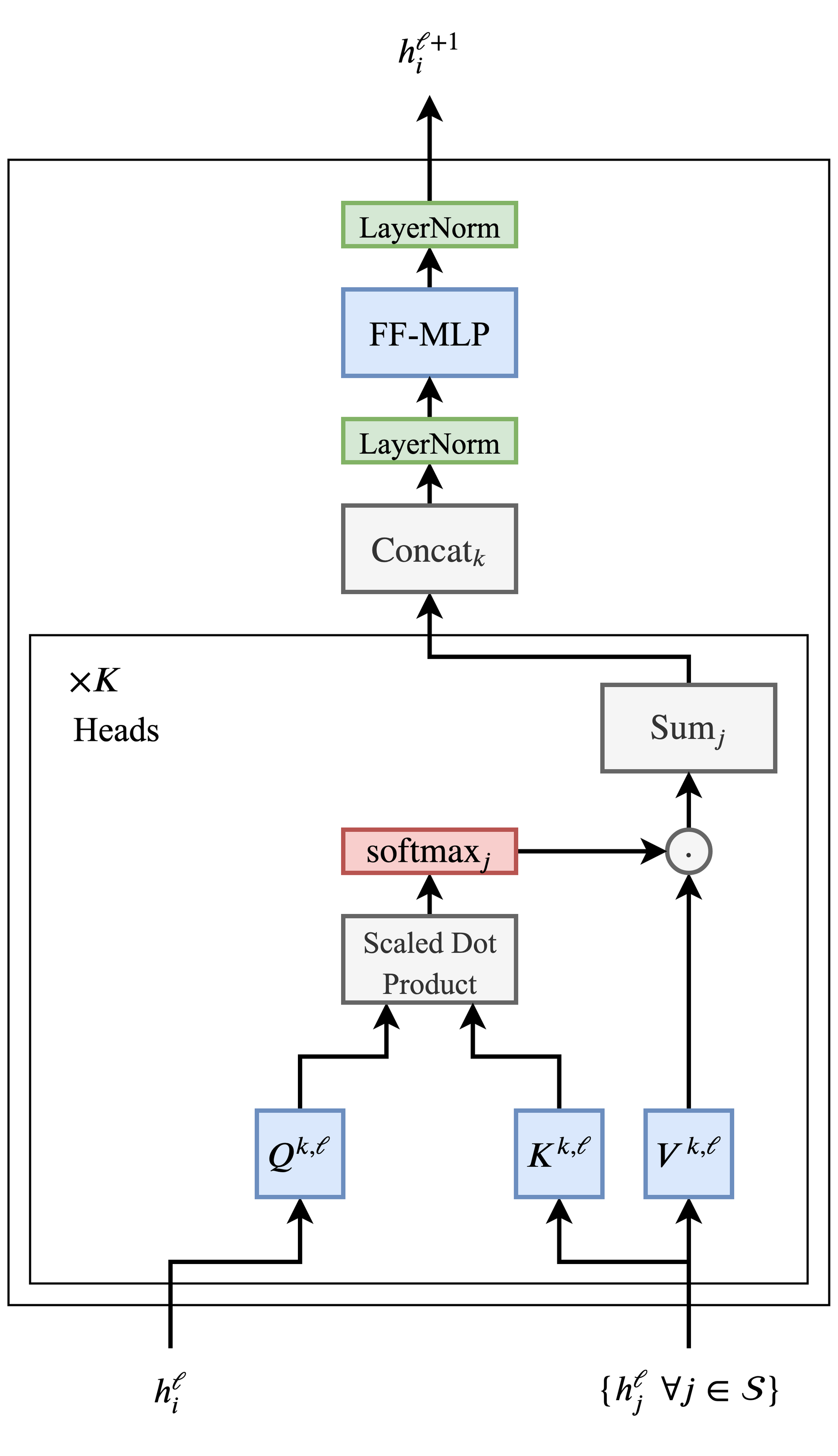• The Transformer architecture is also extremely amenable to very deep networks, enabling the NLP community to scale up in terms of both model parameters and, by extension, data. Residual connections between the inputs and outputs of each multi-head attention sub-layer and the feed-forward sub-layer are key for stacking Transformer layers (but omitted from the diagram for clarity).

## The relation between transformers and Graph Neural Networks

### GNNs build representations of graphs

• Let’s take a step away from NLP for a moment.

• Graph Neural Networks (GNNs) or Graph Convolutional Networks (GCNs) build representations of nodes and edges in graph data. They do so through neighbourhood aggregation (or message passing), where each node gathers features from its neighbours to update its representation of the local graph structure around it. Stacking several GNN layers enables the model to propagate each node’s features over the entire graph—from its neighbours to the neighbours’ neighbours, and so on.

• Take the example of this emoji social network below (source): The node features produced by the GNN can be used for predictive tasks such as identifying the most influential members or proposing potential connections.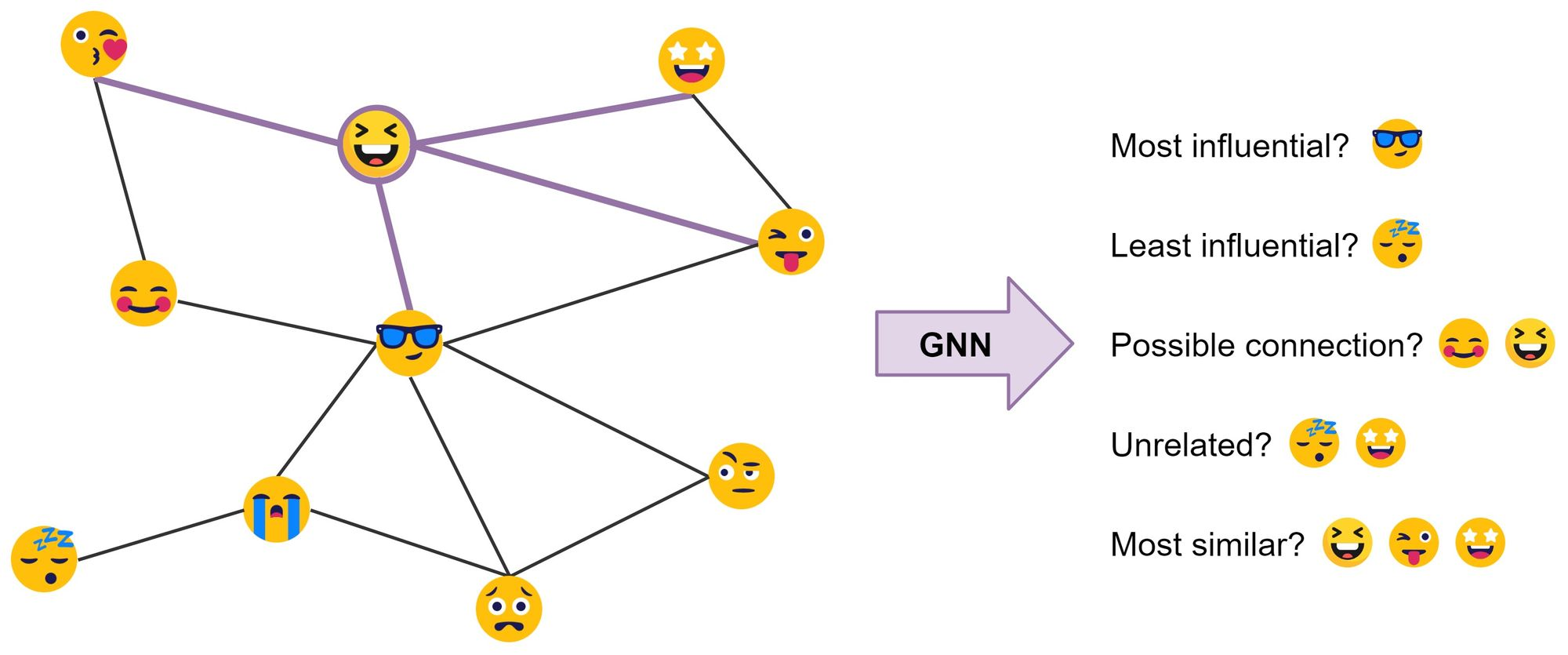• In their most basic form, GNNs update the hidden features $$h$$ of node $$i$$ (for example, 😆) at layer $$\ell$$ via a non-linear transformation of the node’s own features $$h_{i}^{\ell}$$ added to the aggregation of features $$h_{j}^{\ell}$$ from each neighbouring node $$j \in \mathcal{N}(i)$$:

$h_{i}^{\ell+1}=\sigma\left(U^{\ell} h_{i}^{\ell}+\sum_{j \in \mathcal{N}(i)}\left(V^{\ell} h_{j}^{\ell}\right)\right)$
• where $$U^{\ell}, V^{\ell}$$ are learnable weight matrices of the GNN layer and $$\sigma$$ is a non-linear function such as ReLU. In the example, (😆) {😘, 😎, 😜, 🤩}.
• The summation over the neighbourhood nodes $$j \in \mathcal{N}(i)$$ can be replaced by other input sizeinvariant aggregation functions such as simple mean/max or something more powerful, such as a weighted sum via an attention mechanism.

• Does that sound familiar? Maybe a pipeline will help make the connection (figure source):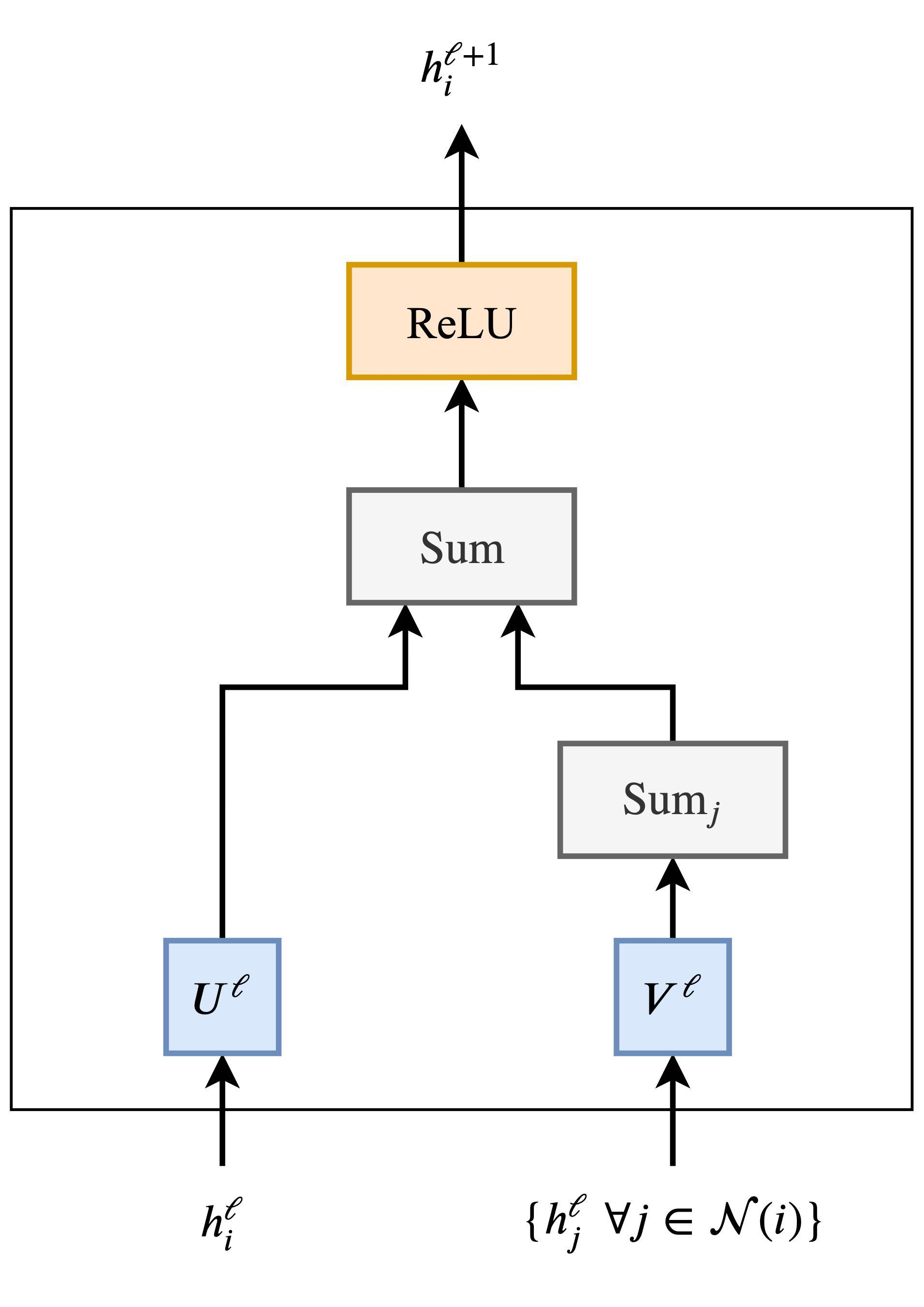• If we were to do multiple parallel heads of neighbourhood aggregation and replace summation over the neighbours $$j$$ with the attention mechanism, i.e., a weighted sum, we’d get the Graph Attention Network (GAT). Add normalization and the feed-forward MLP, and voila, we have a Graph Transformer! Transformers are thus a special case of GNNs – they are just GNNs with multi-head attention.

### Sentences are fully-connected word graphs

• To make the connection more explicit, consider a sentence as a fully-connected graph, where each word is connected to every other word. Now, we can use a GNN to build features for each node (word) in the graph (sentence), which we can then perform NLP tasks with as shown in the figure (source) below.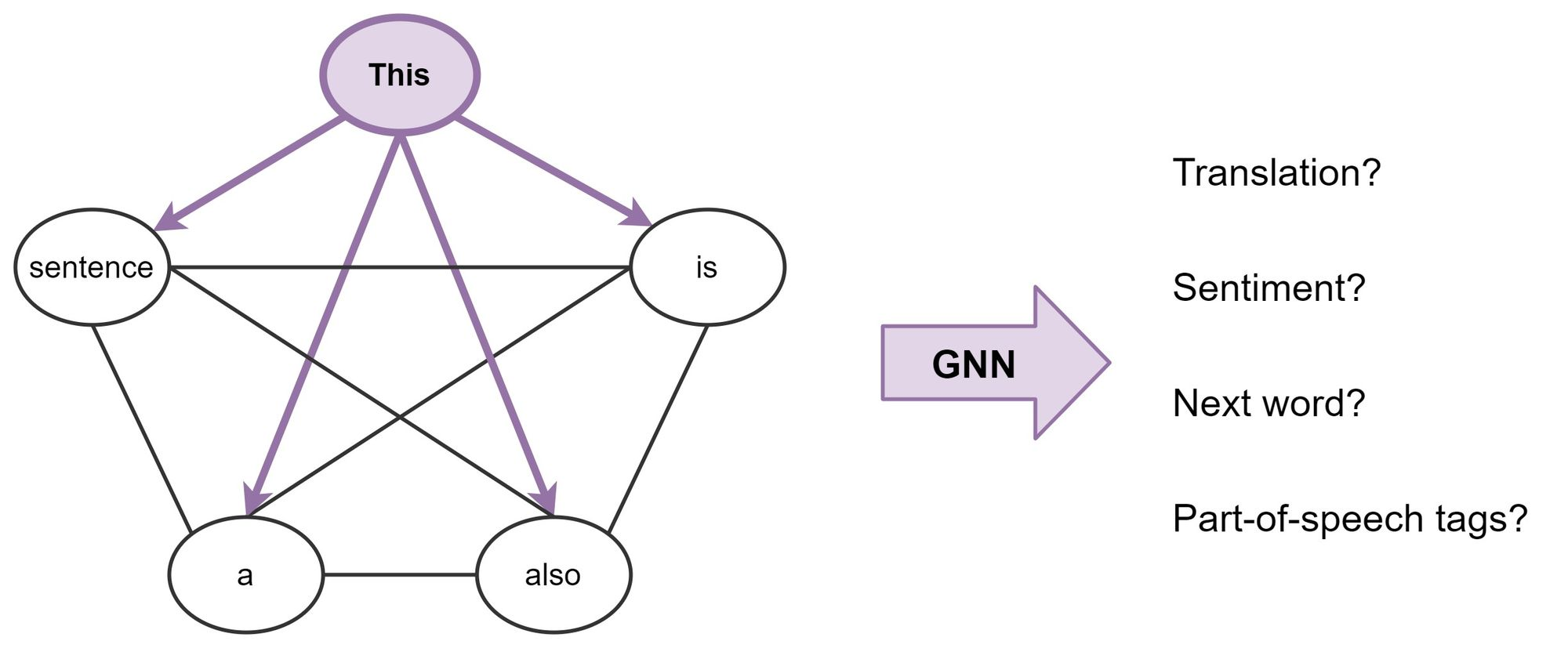• Broadly, this is what Transformers are doing: they are GNNs with multi-head attention as the neighbourhood aggregation function. Whereas standard GNNs aggregate features from their local neighbourhood nodes $$j \in \mathcal{N}(i)$$, Transformers for NLP treat the entire sentence $$\mathcal{S}$$ as the local neighbourhood, aggregating features from each word $$j \in \mathcal{S}$$ at each layer.

• Importantly, various problem-specific tricks—such as position encodings, causal/masked aggregation, learning rate schedules and extensive pre-training—are essential for the success of Transformers but seldom seem in the GNN community. At the same time, looking at Transformers from a GNN perspective could inspire us to get rid of a lot of the bells and whistles in the architecture.

### Inductive biases of transformers

• Based on the above discussion, we’ve established that transformers are indeed a special case of Graph Neural Networks (GNNs) owing to their architecture level commonalities. Relational inductive biases, deep learning, and graph networks by Battaglia et al. (2018) from DeepMind/Google, MIT and the University of Edinburgh offers a great overview of the relational inductive biases of various neural net architectures, summarized in the table below from the paper. Each neural net architecture exhibits varying degrees of relational inductive biases. Transformers fall somewhere between RNNs and GNNs in the table below (source).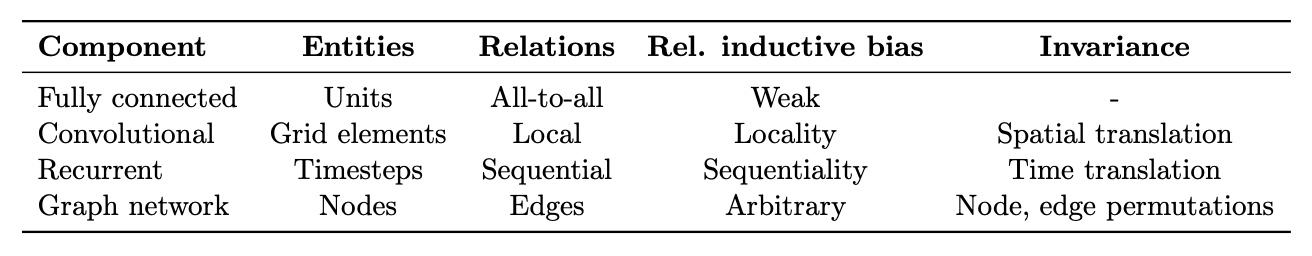## Lessons Learned

### Transformers: merging the worlds of linguistic theory and statistical NLP using fully connected graphs

• Now that we’ve established a connection between Transformers and GNNs, let’s throw some ideas around. For one, are fully-connected graphs the best input format for NLP?

• Before statistical NLP and ML, linguists like Noam Chomsky focused on developing formal theories of linguistic structure, such as syntax trees/graphs. Tree LSTMs already tried this, but maybe Transformers/GNNs are better architectures for bringing together the two worlds of linguistic theory and statistical NLP? For example, a very recent work from MILA and Stanford explores augmenting pre-trained Transformers such as BERT with syntax trees [Sachan et al., 2020. The figure below from Wikipedia: Syntactic Structures shows a tree diagram of the sentence “Colorless green ideas sleep furiously”: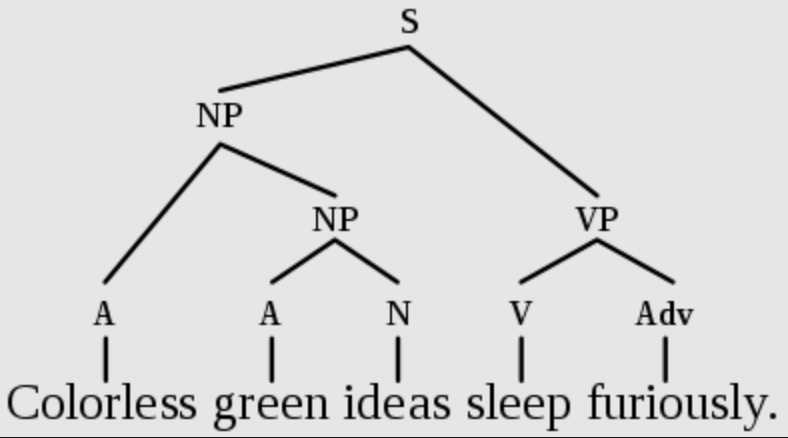### Long term dependencies

• Another issue with fully-connected graphs is that they make learning very long-term dependencies between words difficult. This is simply due to how the number of edges in the graph scales quadratically with the number of nodes, i.e., in an $$n$$ word sentence, a Transformer/GNN would be doing computations over $$n^{2}$$ pairs of words. Things get out of hand for very large $$n$$.

• The NLP community’s perspective on the long sequences and dependencies problem is interesting: making the attention mechanism sparse or adaptive in terms of input size, adding recurrence or compression into each layer, and using Locality Sensitive Hashing for efficient attention are all promising new ideas for better transformers. See Maddison May’s excellent survey on long-term context in Transformers for more details.

• It would be interesting to see ideas from the GNN community thrown into the mix, e.g., Binary Partitioning for sentence graph sparsification seems like another exciting approach. BP-Transformers recursively sub-divide sentences into two until they can construct a hierarchical binary tree from the sentence tokens. This structural inductive bias helps the model process longer text sequences in a memory-efficient manner. The following figure from Ye et al. (2019) shows binary partitioning for sentence graph sparsification.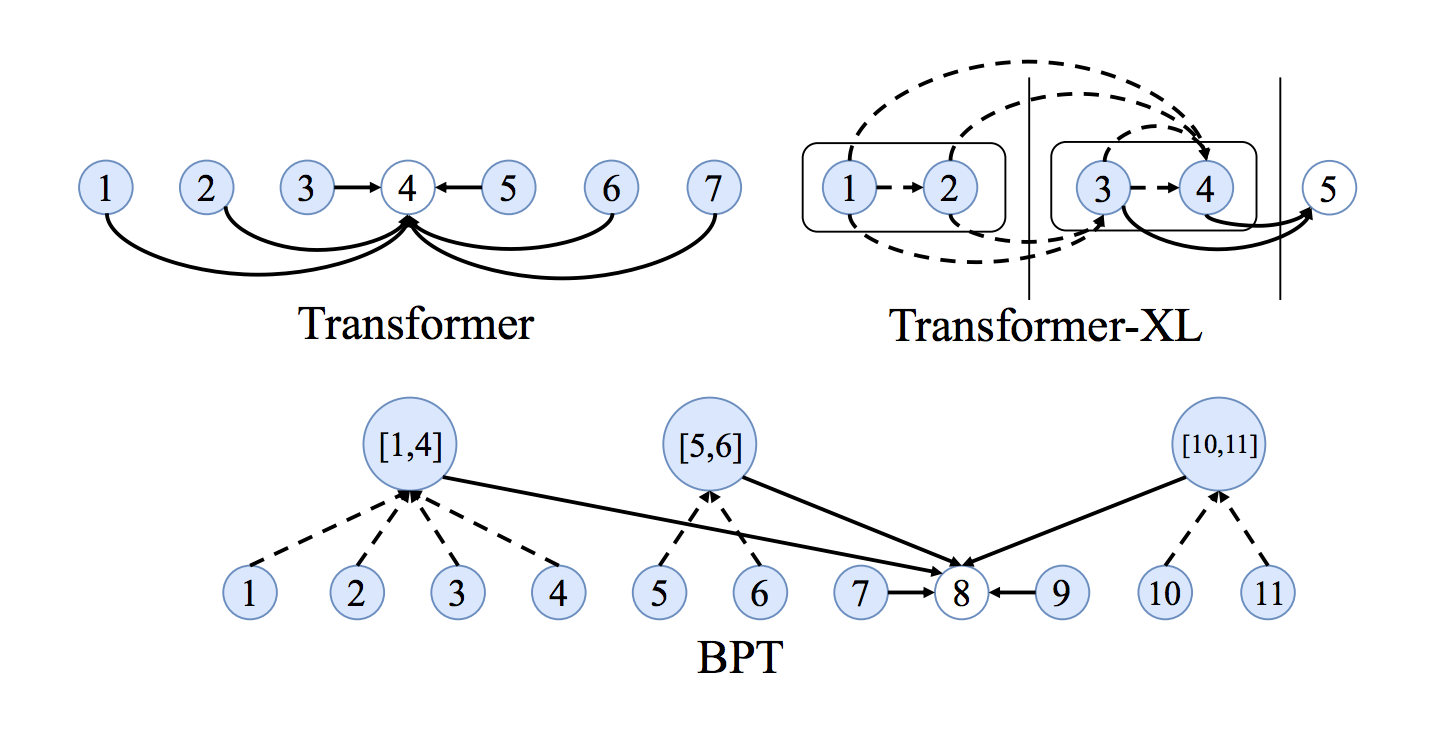### Are Transformers learning neural syntax?

• There have been several interesting papers from the NLP community on what Transformers might be learning. The basic premise is that performing attention on all word pairs in a sentence – with the purpose of identifying which pairs are the most interesting – enables Transformers to learn something like a task-specific syntax.
• Different heads in the multi-head attention might also be ‘looking’ at different syntactic properties, as shown in the figure (source) below.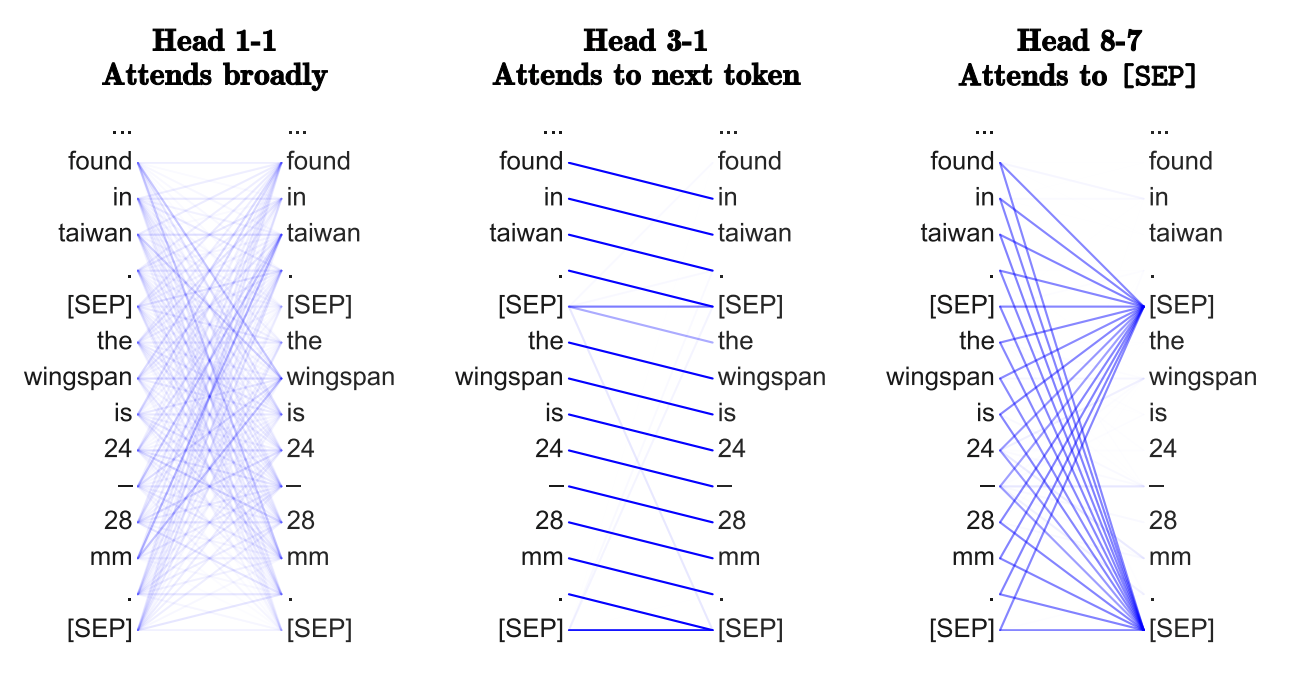### Benefits of Transformers compared to RNNs/GRUs/LSTMs

• The Transformer can learn longer-range dependencies than RNNs and its variants such as GRUs and LSTMs.
• The biggest benefit, however, comes from how the Transformer lends itself to parallelization. Unlike an RNN which processes a word at each time step, a key property of the Transformer is that the word at each position flows through its own path in the encoder. There are dependencies between these paths in the self-attention layer (since the self-attention layer computes how important each other word in the input sequence is to this word). However, once the self-attention output is generated, the feed-forward layer does not have those dependencies, and thus the various paths can be executed in parallel while flowing through the feed-forward layer. This is an especially useful trait in case of the Transformer encoder which can process each input word in parallel with other words after the self-attention layer. This feature, is however, not of great importance for the decoder since it generates one word at a time and thus does not utilize parallel word paths.

### What would we like to fix about the transformer? / Drawbacks of Transformers

• The biggest drawback of the Transformer architecture is the quadratic computational complexity with respect to both the number of tokens ($$n$$) and the embedding size ($$d$$). This means that as sequences get longer, the time and computational resources needed for training increase significantly. A detailed discourse on this and a couple of secondary drawbacks are as below.
1. Quadratic time and space complexity of the attention layer:
• Transformers use what’s known as self-attention, where each token in a sequence attends to all other tokens (including itself). This implies that the runtime of the Transformer architecture is quadratic in the length of the input sequence, which means it can be slow when processing long documents or taking characters as inputs. If you have a sequence of $$n$$ tokens, you’ll essentially have to compute attention scores for each pair of tokens, resulting in $$n^2$$ (quadratic) computations. In other words, computing all pairs of interactions (i.e., attention over all word-pairs) during self-attention means our computation grows quadratically with the sequence length, i.e., $$O(T^2 d)$$, where $$T$$ is the sequence length, and $$d$$ is the dimensionality.
• In a graph context, self-attention mandates that the number of edges in the graph to scale quadratically with the number of nodes, i.e., in an $$n$$ word sentence, a Transformer would be doing computations over $$n^{2}$$ pairs of words. Note that for recurrent models, it only grew linearly.
• This implies a large parameter count (implying high memory footprint) and thus, high computational complexity.
• Say, $$d = 1000$$. So, for a single (shortish) sentence, $$T \leq 30 \Rightarrow T^{2} \leq 900 \Rightarrow T^2 d \approx 900K$$. Note that in practice, we set a bound such as $$T = 512$$. Imagine working on long documents with $$T \geq 10,000$$?!
• High compute requirements has a negative impact on power and battery life requirements, especially for portable device targets.
• Similarly, for storing these attention scores, you’d need space that scales with $$n^2$$, leading to a quadratic space complexity.
• This becomes problematic for very long sequences as both the computation time and memory usage grow quickly, limiting the practical use of standard transformers for lengthy inputs.
• Overall, a transformer requires higher computational power (and thus, lower battery life) and memory footprint compared to its conventional counterparts.
• Wouldn’t it be nice for Transformers if we didn’t have to compute pair-wise interactions between each word pair in the sentence? Recent studies such as the following show that decent performance levels can be achieved without computing interactions between all word-pairs (such as by approximating pair-wise attention).
2. Quadratic time complexity of linear layers w.r.t. embedding size $$d$$:
• In Transformers, after calculating the attention scores, the result is passed through linear layers, which have weights that scale with the dimension of the embeddings. If your token is represented by an embedding of size $$d$$, and if $$d$$ is greater than $$n$$ (the number of tokens), then the computation associated with these linear layers can also be demanding.
• The complexity arises because for each token, you’re doing operations in a $$d$$-dimensional space. For densely connected layers, if $$d$$ grows, the number of parameters and hence computations grows quadratically.
3. Positional Sinusoidal Embedding:
• Transformers, in their original design, do not inherently understand the order of tokens (i.e., they don’t recognize sequences). To address this, positional information is added to the token embeddings.
• The original Transformer model (by Vaswani et al.) proposed using sinusoidal functions to generate these positional embeddings. This method allows models to theoretically handle sequences of any length (since sinusoids are periodic and continuous), but it might not be the most efficient or effective way to capture positional information, especially for very long sequences or specialized tasks. Hence, it’s often considered a limitation or area of improvement, leading to newer positional encoding methods like Rotary Positional Embeddings (RoPE).
4. Data appetite of Transformers vs. sample-efficient architectures:
• Furthermore, compared to CNNs, the sample complexity (i.e., data appetite) of transformers is obscenely high. CNNs are still sample efficient, which makes them great candidates for low-resource tasks. This is especially true for image/video generation tasks where an exceptionally large amount of data is needed, even for CNN architectures (and thus implies that Transformer architectures would have a ridiculously high data requirement). For example, the recent CLIP architecture by Radford et al. was trained with CNN-based ResNets as vision backbones (and not a ViT-like transformer architecture).
• Put simply, while Transformers do offer accuracy lifts once their data requirement is satisfied, CNNs offer a way to deliver reasonable performance in tasks where the amount of data available is not exceptionally high. Both architectures thus have their use-cases.

### Why is training Transformers so hard?

• Reading new Transformer papers makes me feel that training these models requires something akin to black magic when determining the best learning rate schedule, warmup strategy and decay settings. This could simply be because the models are so huge and the NLP tasks studied are so challenging.
• But recent results suggest that it could also be due to the specific permutation of normalization and residual connections within the architecture.

• In the field of NLP, Transformers have already established themselves as the numero uno architectural choice or the de facto standard for a plethora of NLP tasks.
• Likewise, in the field of vision, an updated version of ViT was second only to a newer approach that combines CNNs with transformers on the ImageNet image classification task at the start of 2022. CNNs without transformers, the longtime champs, barely reached the top 10!
• It is quite likely that transformers or hybrid derivatives thereof (combining concepts of self-attention with say convolutions) will be the leading architectures of choice in the near future, especially if functional metrics (such as accuracy) are the sole optimization metrics. However, along other axes such as data, computational complexity, power/battery life, and memory footprint, transformers are currently not the best choice – which the above section on What Would We Like to Fix about the Transformer? / Drawbacks of Transformers expands on.
• Could Transformers benefit from ditching attention, altogether? Yann Dauphin and collaborators’ recent work suggests an alternative ConvNet architecture. Transformers, too, might ultimately be doing something similar to ConvNets!

## Choosing the right language model for your NLP use-case: key takeaways

• Some key takeaways for LLM selection and deployment:
1. When evaluating potential models, be clear about where you are in your AI journey:
• In the beginning, it might be a good idea to experiment with LLMs deployed via cloud APIs.
• Once you have found product-market fit, consider hosting and maintaining your model on your side to have more control and further sharpen model performance to your application.
2. To align with your downstream task, your AI team should create a short list of models based on the following criteria:
• Alignment between the pre-training objective and downstream task: consider auto-encoding for NLU and autoregression for NLG. The figure below shows the best LLMs depending on the NLP use-case (image source):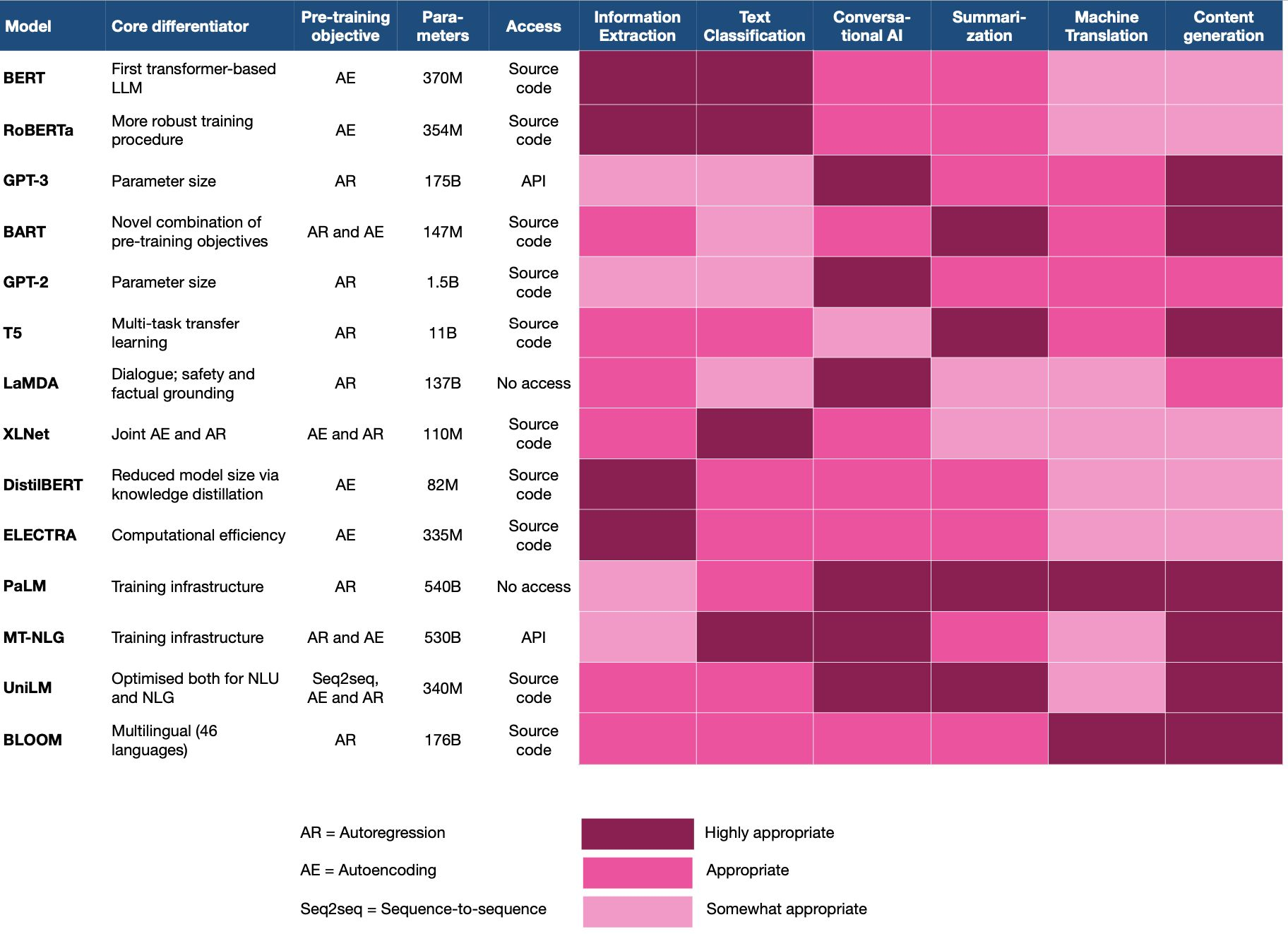3. The short-listed models should be then tested against your real-world task and dataset to get a first feeling for the performance.
4. In most cases, you are likely to achieve better quality with dedicated fine-tuning. However, consider few/zero-shot learning if you don’t have the internal tech skills or budget for fine-tuning, or if you need to cover a large number of tasks.
5. LLM innovations and trends are short-lived. When using language models, keep an eye on their lifecycle and the overall activity in the LLM landscape and watch out for opportunities to step up your game.

## Transformers Learning Recipe

• Transformers have accelerated the development of new techniques and models for natural language processing (NLP) tasks. While it has mostly been used for NLP tasks, it is now seeing heavy adoption in other areas such as computer vision and reinforcement learning. That makes it one of the most important modern concepts to understand and be able to apply.
• A lot of machine learning and NLP students and practitioners are keen on learning about transformers. Therefore, this recipe of resources and study materials should be helpful to help guide students interested in learning about the world of Transformers.
• To dive deep into the Transformer architecture from an NLP perspective, here’s a few links to better understand and implement transformer models from scratch.

### The Illustrated Transformer

• Jay Alammar’s illustrated explanations are exceptional. Once you get that high-level understanding of transformers, going through The Illustrated Transformer is recommend for its detailed and illustrated explanation of transformers: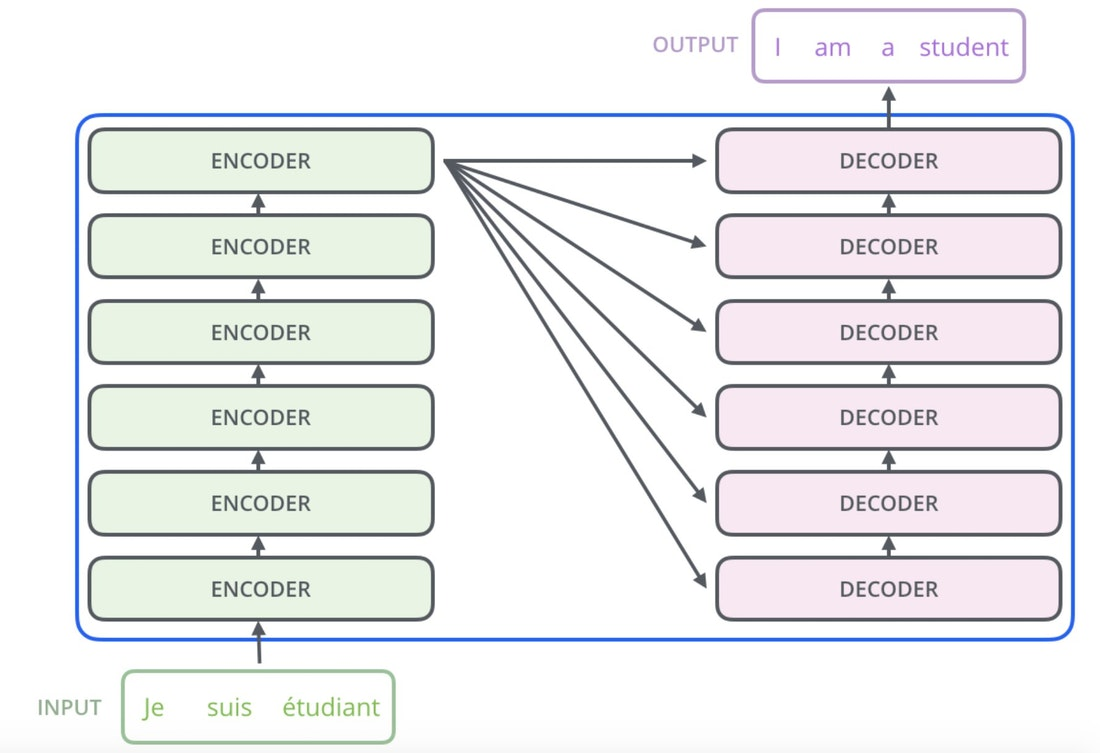### Technical Summary

• At this point, you may be looking for a technical summary and overview of transformers. Lilian Weng’s The Transformer Family is a gem and provides concise technical explanations/summaries: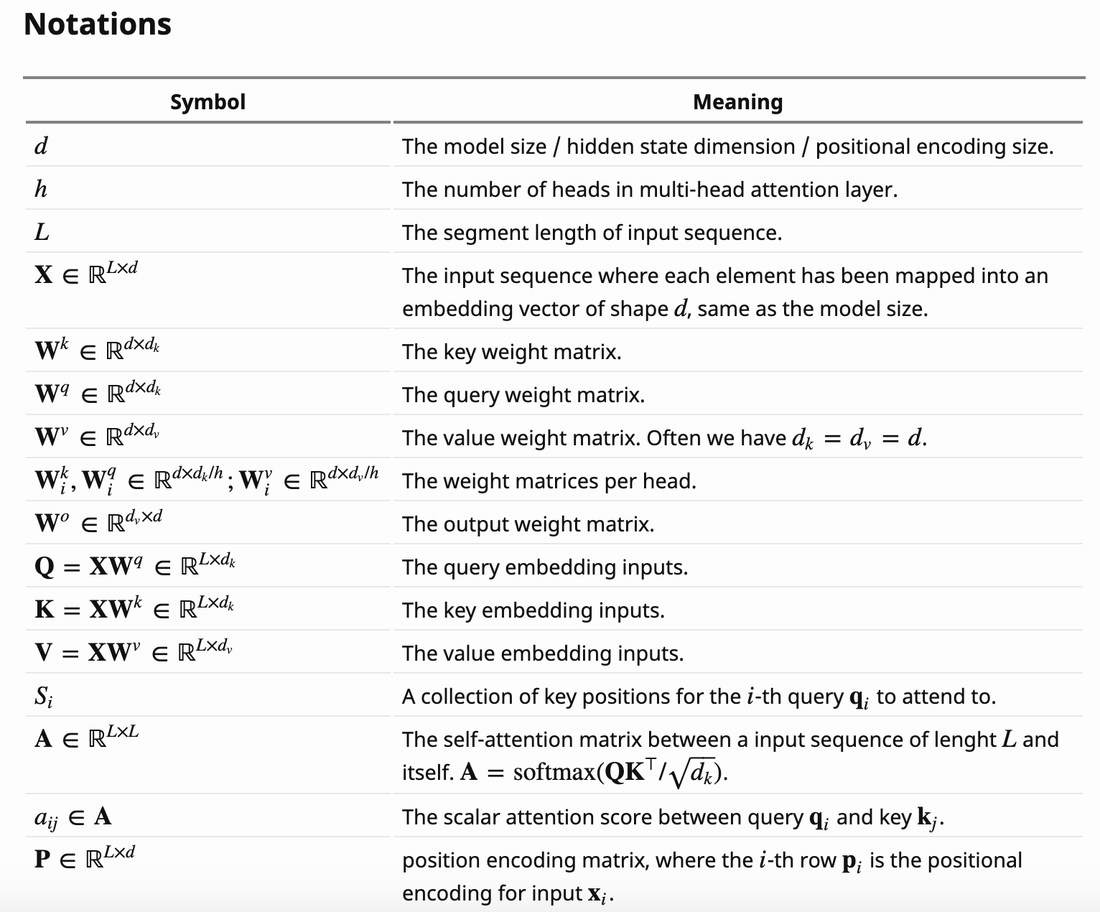### Implementation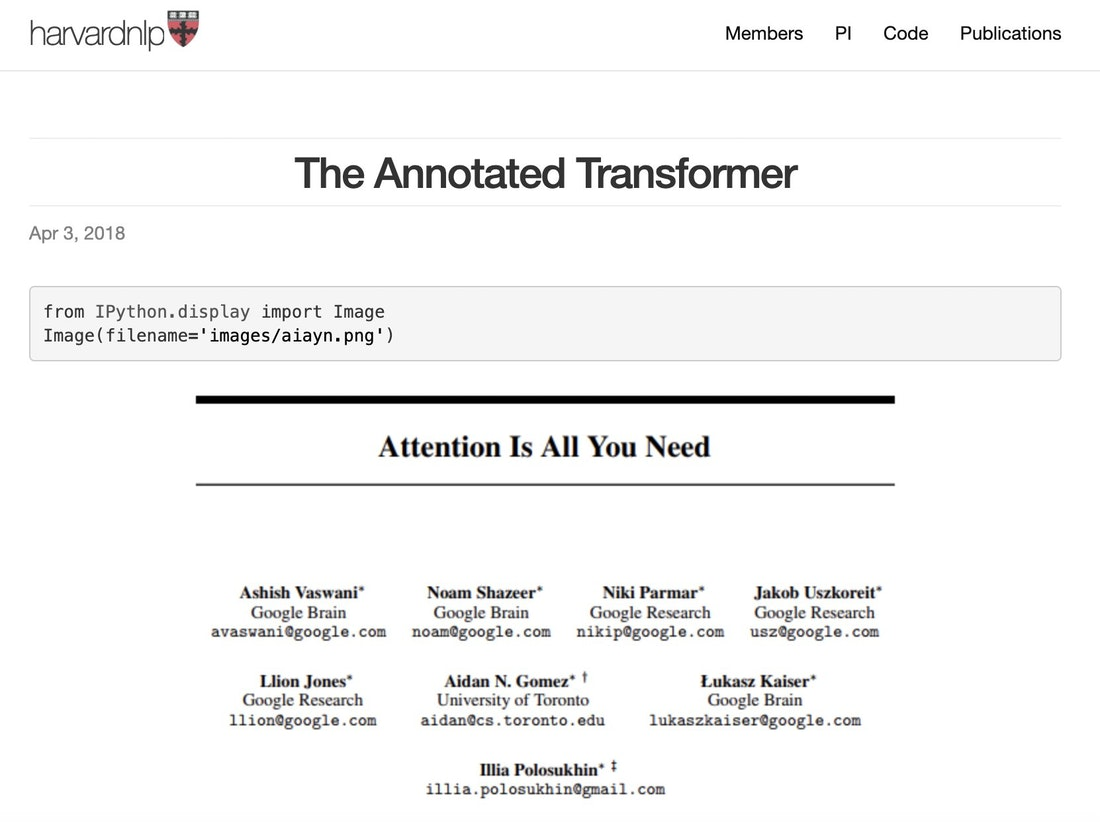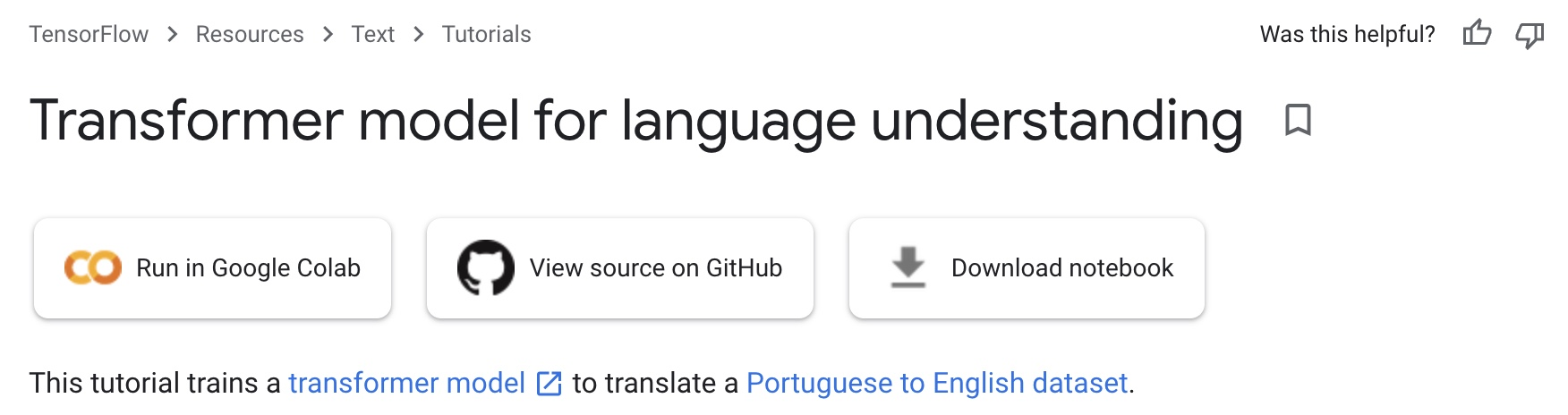### Attention Is All You Need

• This paper by Vaswani et al. introduced the Transformer architecture. Read it after you have a high-level understanding and want to get into the details. Pay attention to other references in the paper for diving deep.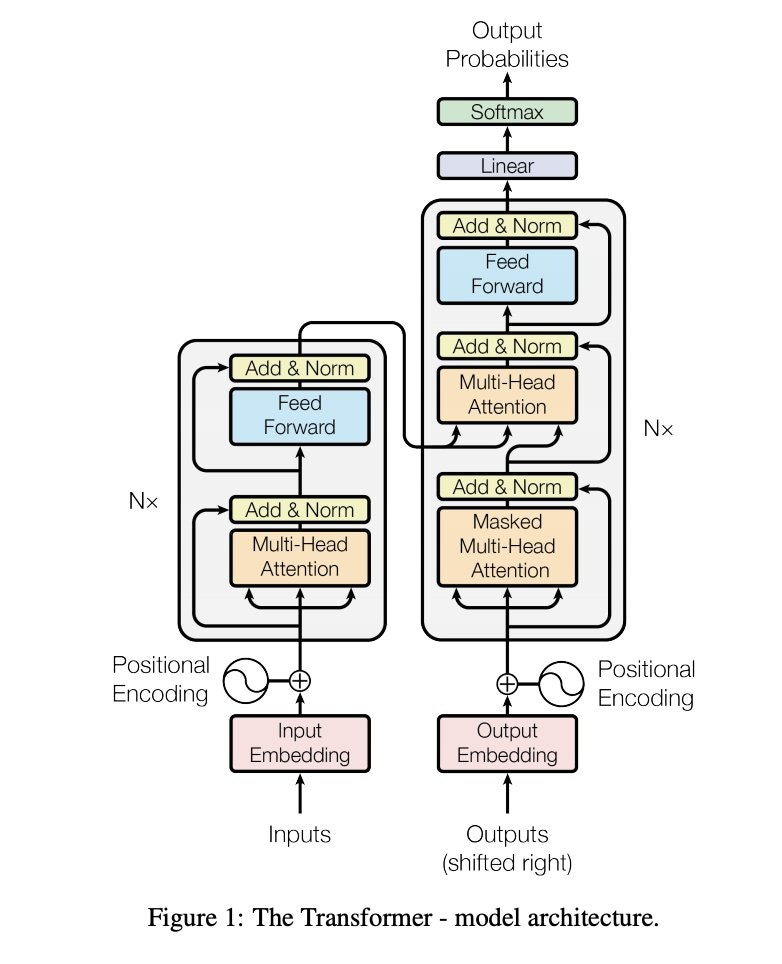### Applying Transformers

• After some time studying and understanding the theory behind transformers, you may be interested in applying them to different NLP projects or research. At this time, your best bet is the Transformers library by HuggingFace.
• The Hugging Face Team has also published a new book on NLP with Transformers, so you might want to check that out as well.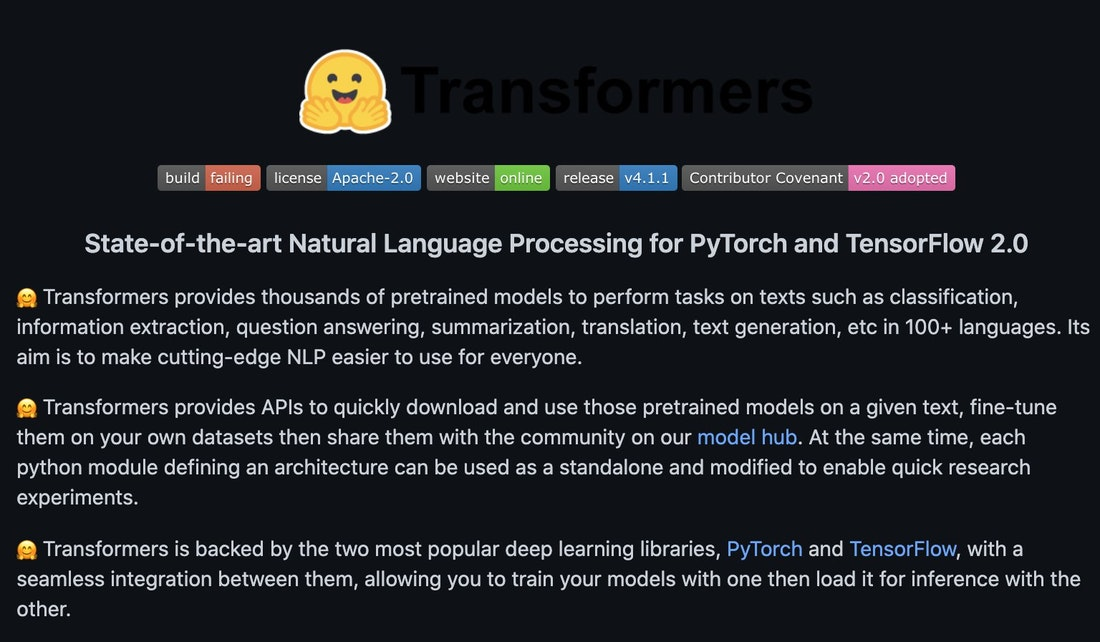@article{Chadha2020DistilledTransformers,